# Iterative visualizations with ggplot2: no more copy-pasting

Are you tired of copy-pasting some chunks of your code over and over again? I am, too. Let’s dig into how we can improve our workflow with a bit of tidy evaluation and writing our own functions to avoid copy-pasting.

## Tidy (non-standard) evaluation

• How code is being quoted
• How code is being unquoted

# data masking: access columns indirectly
# regular (standard) evaluation
data[data$ATC == "N06A" & data$gender_text == "women" & data$year == 1999, ][1:10, ]  ## # A tibble: 10 × 20 ## ATC year sector region gender age number_of_people patients_per_1000_in… ## <chr> <dbl> <chr> <chr> <chr> <dbl> <dbl> <dbl> ## 1 N06A 1999 0 0 2 0 0 0.120 ## 2 N06A 1999 0 0 2 11 25 7.05 ## 3 N06A 1999 0 0 2 21 638 25.7 ## 4 N06A 1999 0 0 2 31 1357 44.7 ## 5 N06A 1999 0 0 2 41 2159 70.1 ## 6 N06A 1999 0 0 2 51 3070 83.8 ## 7 N06A 1999 0 0 2 61 2556 101. ## 8 N06A 1999 0 0 2 71 2543 136. ## 9 N06A 1999 0 0 2 81 2565 183. ## 10 N06A 1999 0 0 2 91 817 210. ## # … with 12 more variables: turnover <dbl>, regional_grant_paid <dbl>, ## # quantity_sold_1000_units <dbl>, ## # quantity_sold_units_per_unit_1000_inhabitants_per_day <dbl>, ## # percentage_of_sales_in_the_primary_sector <chr>, region_text <chr>, ## # gender_text <chr>, age_cats <chr>, age_cat <fct>, ## # denominator_per_year <dbl>, numerator <dbl>, denominator <dbl>  # tidy: with masking .data[[var]] --> var data %>% filter(ATC == "N06A", gender_text == "women", year == 1999) %>% slice(1:10)  ## # A tibble: 10 × 20 ## ATC year sector region gender age number_of_people patients_per_1000_in… ## <chr> <dbl> <chr> <chr> <chr> <dbl> <dbl> <dbl> ## 1 N06A 1999 0 0 2 0 0 0.120 ## 2 N06A 1999 0 0 2 11 25 7.05 ## 3 N06A 1999 0 0 2 21 638 25.7 ## 4 N06A 1999 0 0 2 31 1357 44.7 ## 5 N06A 1999 0 0 2 41 2159 70.1 ## 6 N06A 1999 0 0 2 51 3070 83.8 ## 7 N06A 1999 0 0 2 61 2556 101. ## 8 N06A 1999 0 0 2 71 2543 136. ## 9 N06A 1999 0 0 2 81 2565 183. ## 10 N06A 1999 0 0 2 91 817 210. ## # … with 12 more variables: turnover <dbl>, regional_grant_paid <dbl>, ## # quantity_sold_1000_units <dbl>, ## # quantity_sold_units_per_unit_1000_inhabitants_per_day <dbl>, ## # percentage_of_sales_in_the_primary_sector <chr>, region_text <chr>, ## # gender_text <chr>, age_cats <chr>, age_cat <fct>, ## # denominator_per_year <dbl>, numerator <dbl>, denominator <dbl>  # coding means processing the expressions # quoting: delaying the code execution behind the scenes # expr() turns things into symbols # sym() turns strings into symbols x <- c(2, 2, 4, 4) mean(x, na.rm = TRUE)  ##  3  expr(mean(x, na.rm = TRUE))  ## mean(x, na.rm = TRUE)  expr(x)  ## x  sym("x")  ## x  # many things: exprs() is useful interactively to make a list of expressions rlang::exprs(x = x * 15, y = d / 3, z = f ^ 2)  ##$x
## x * 15
##
## $y ## d/3 ## ##$z
## f^2

# shorthand for
# list(x = expr(x * 15), y = expr(d / 3), z = expr(f ^ 2))

# base version of expr() is quote()
quote(1+2)

## 1 + 2

# unquoting: used when it's time to process the quoted expression
# the unquote operator !! (pronounced bang-bang)

xx <- expr(x + x)
yy <- expr(y + y)

expr(xx / yy)

## xx/yy

expr(!!xx / !!yy)

## (x + x)/(y + y)

# !!!, called “unquote-splice”, unquote many arguments
manyexpr <- rlang::exprs(1, a + 3, -b/10)

manyexpr[]

##  1

manyexpr[]

## a + 3

manyexpr[]

## -b/10

expr(f(!!! manyexpr))

## f(1, a + 3, -b/10)

expr(f(!!manyexpr[]) + yy)

## f(1) + yy


# Visualization

# make a plot of Antidepressants (ATC:N06A) utilization rates in men & women separately 30-50 in 1999-2019, DK

data %>%
filter(region == "0", str_detect(ATC, "^N06A$"), age_cat %in% c("(30,40]", "(40,50]", "(50,60]")) %>% mutate( label = if_else(year == 1999 | year == 2009 | year == 2019, as.character(sprintf("%1.1f", round(patients_per_1000_inhabitants, digits = 1))), NA_character_) ) %>% ggplot(aes(x = year, y = patients_per_1000_inhabitants, color = gender)) + geom_path() + facet_grid(cols = vars(gender_text), rows = vars(age_cat), scales = "fixed", drop = T) + theme_light(base_size = 12) + # start y scale at 0 expand_limits(y = 0) + scale_x_continuous(breaks = c(seq(1999, 2019, 10))) + ggrepel::geom_label_repel(aes(label = label), na.rm = TRUE, nudge_x = 0.1, direction = "y", segment.size = 0.1, segment.colour = "black", show.legend = F) + scale_color_manual(values = wes_palette(name = "GrandBudapest1", type = "discrete")) + theme(plot.caption = element_text(hjust = 0, size = 10), legend.position = "none", panel.spacing = unit(0.8, "cm")) + labs(y = "Patients\nper 1,000 women in the population", title = paste0("Antidepressants utilization in DK"), subtitle = "by age categories", caption = "Source: medstat.dk\n@evpatora")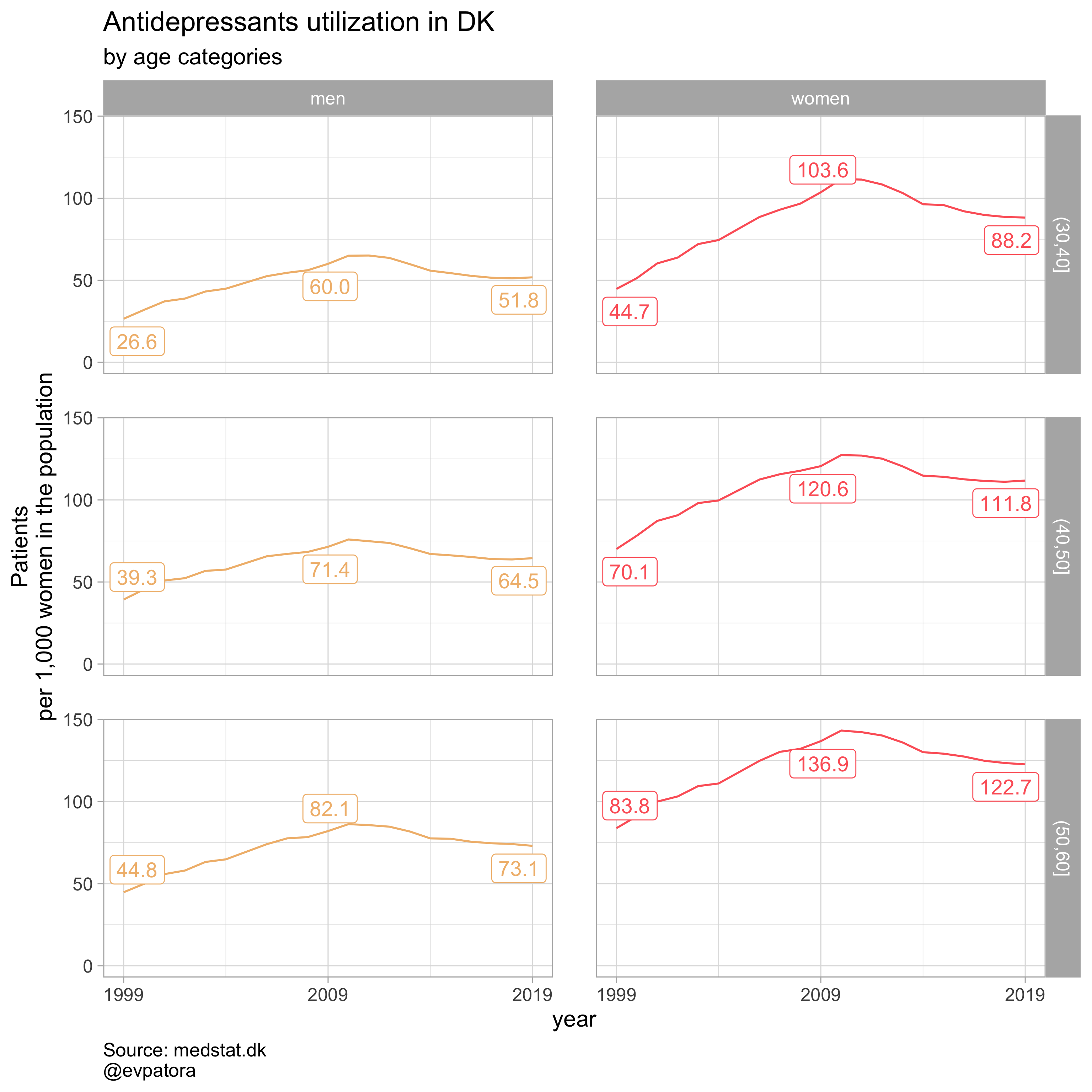List of the drugs of interest regex_antidepress <- "^N06A$"
regex_antipsych <- "^N05A$" regex_anxiolyt <- "^N05B$"
regex_sedat <- "^N05C\$"


## Writing ggplot2 wrapper function

In tidyverse instead of quoting and unquoting in 2 separate steps, it can be combined in 1 step with {{ “curly-curly” operator. I take the plotting function above and wrap it inside my custom function. ATC column becomes {{ atc }}, a “curly-curly” operator embraced column, which I as a user will specify when using my custom function. Same logic is applied to data column age_cat, which becomes {{ age_var }}; year column, which becomes {{ year_var }}, etc. Once I worked with all the columns I need to use to produce the wanted graphics and minding that this function may be useful for the same or similar datasets with the columns named differently, I test my custom function.

plot_utilization <- function(.my_data, drug_regex, atc, age_var, year_var, rate_var, sex_var, title){

.my_data %>%  # use data pronoun to make the function pipe-able
# filter non-missing values on utilization rates
filter(! is.na({{ rate_var }})) %>%
# filter needed ATC code and age age categories "(30,40]", "(40,50]", "(50,60]"
filter(str_detect({{ atc }}, drug_regex), {{ age_var }} %in% c("(30,40]", "(40,50]", "(50,60]"))  %>%
mutate(
label = if_else({{ year_var }} == 1999 | {{ year_var }} == 2009 | {{ year_var }} == 2019, as.character(sprintf("%1.1f", round({{ rate_var }}, digits = 1))), NA_character_)
) %>%
ggplot(aes(x = {{ year_var }}, y = {{ rate_var }}, color = {{ sex_var }})) +
geom_path() +
facet_grid(cols = vars({{ sex_var }}), rows = vars({{ age_var }}), scales = "fixed", drop = T) +
theme_light(base_size = 12) +
scale_x_continuous(breaks = c(seq(1999, 2019, 10))) +
expand_limits(y = 0) +
ggrepel::geom_label_repel(aes(label = label), na.rm = TRUE, nudge_x = 0.1, direction = "y", segment.size = 0.1, segment.colour = "black", show.legend = F) +
theme(plot.caption = element_text(hjust = 0, size = 10),
legend.position = "none",
panel.spacing = unit(0.8, "cm")) +
scale_color_manual(values = wes_palette(name = "GrandBudapest1", type = "discrete")) +
labs(y = "Patients\nper 1,000 women in the population", title = paste0(title, " utilization in DK"), subtitle = "by age categories", caption = "Source: medstat.dk\n@evpatora")
}

# testing the custom function
data %>%
# I only want the country-level graph, hence region == 0
filter(region == "0") %>%
# . is my data pronoun
plot_utilization(., atc = ATC, drug_regex = regex_antipsych, age_var = age_cat, year_var = year, rate_var = patients_per_1000_inhabitants, sex_var = gender_text, title = "Antipsychotics")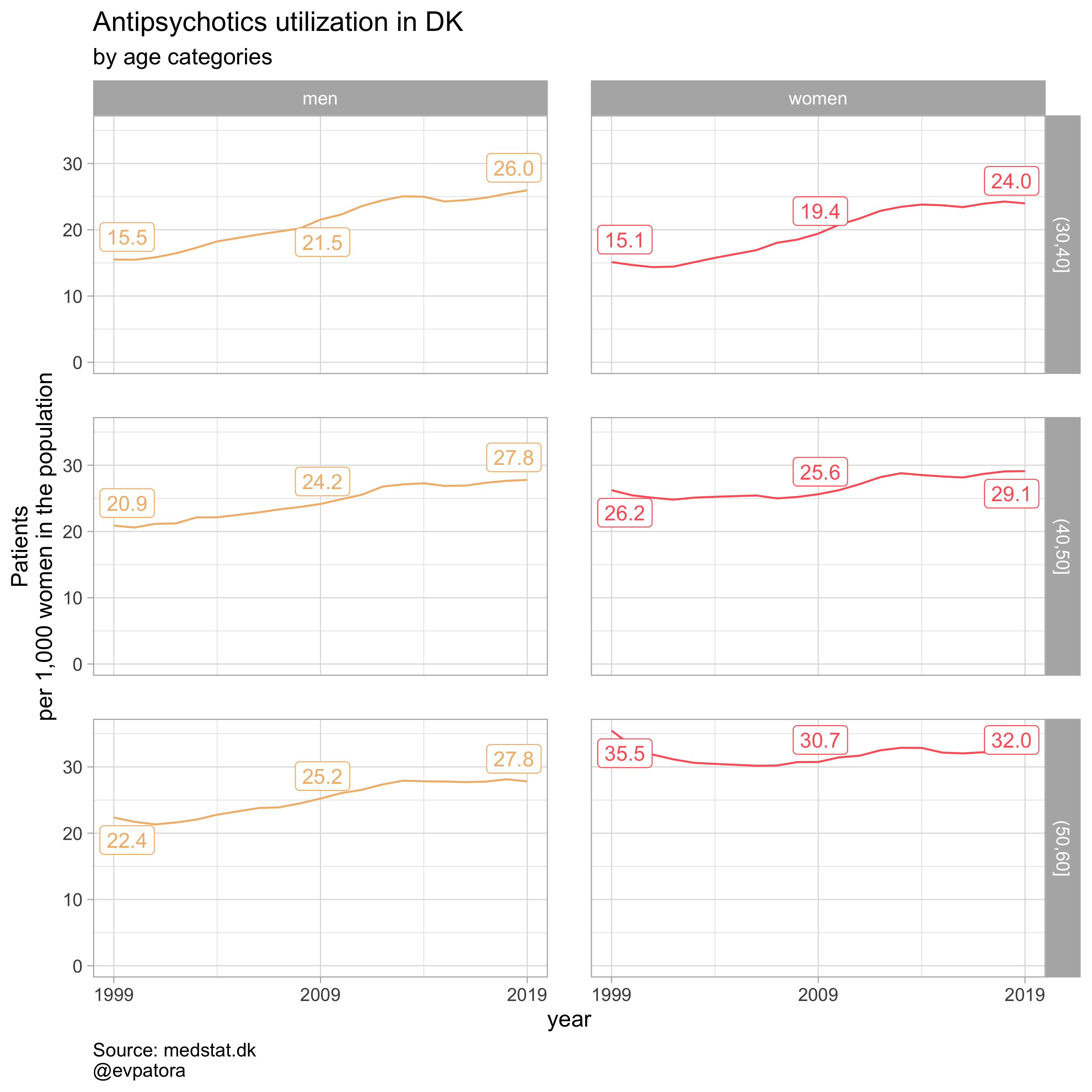## Customizing ggplot2 wrapper function

### Adding filtering

Say, I am now only interested in learning the drug utilization rates on the country-level, therefore I want to skip filtering data every time before I run my custom function. I can incorporate this step directly into my custom function.

plot_utilization_region <- function(.my_data, drug_regex, atc, age_var, year_var, rate_var, sex_var, title, region_var = region){
.my_data %>%
# filter non-missing values on utilization rates
filter(! is.na({{ rate_var }})) %>%
# add filtering the region into my custom function minding that the column can be named differently by me or other users of this function, hence region column becomes {{ region_var }}
filter({{ region_var }} == "0", str_detect({{ atc }}, drug_regex), {{ age_var }} %in% c("(30,40]", "(40,50]", "(50,60]"))  %>%
mutate(
label = if_else({{ year_var }} == 1999 | {{ year_var }} == 2009 | {{ year_var }} == 2019, as.character(sprintf("%1.1f", round({{ rate_var }}, digits = 1))), NA_character_)
) %>%
ggplot(aes(x = {{ year_var }}, y = {{ rate_var }}, color = {{ sex_var }})) +
geom_path() +
facet_grid(cols = vars({{ sex_var }}), rows = vars({{ age_var }}), scales = "fixed", drop = T) +
theme_light(base_size = 12) +
scale_x_continuous(breaks = c(seq(1999, 2019, 10))) +
ggrepel::geom_label_repel(aes(label = label), na.rm = TRUE, nudge_x = 0.1, direction = "y", segment.size = 0.1, segment.colour = "black", show.legend = F) +
expand_limits(y = 0) +
scale_color_manual(values = wes_palette(name = "GrandBudapest1", type = "discrete")) +
theme(plot.caption = element_text(hjust = 0, size = 10),
legend.position = "none",
panel.spacing = unit(0.8, "cm")) +
labs(y = "Patients\nper 1,000 women in the population", title = paste0(title, " utilization in DK"), subtitle = "by age categories", caption = "Source: medstat.dk\n@evpatora")
}

# prepare the list to iterate along
list_regex <- list(regex_antidepress, regex_antipsych, regex_anxiolyt, regex_sedat)
list_title <- list("Antidepressants", "Antipsychotics", "Anxiolytics", "Sedatives")

# iteration
list_plots <- map2(list_regex, list_title,
~plot_utilization_region(.my_data = data, atc = ATC, drug_regex = ..1,
age_var = age_cat, year_var = year, rate_var = patients_per_1000_inhabitants,
sex_var = gender_text, title = ..2))

list_plots

## []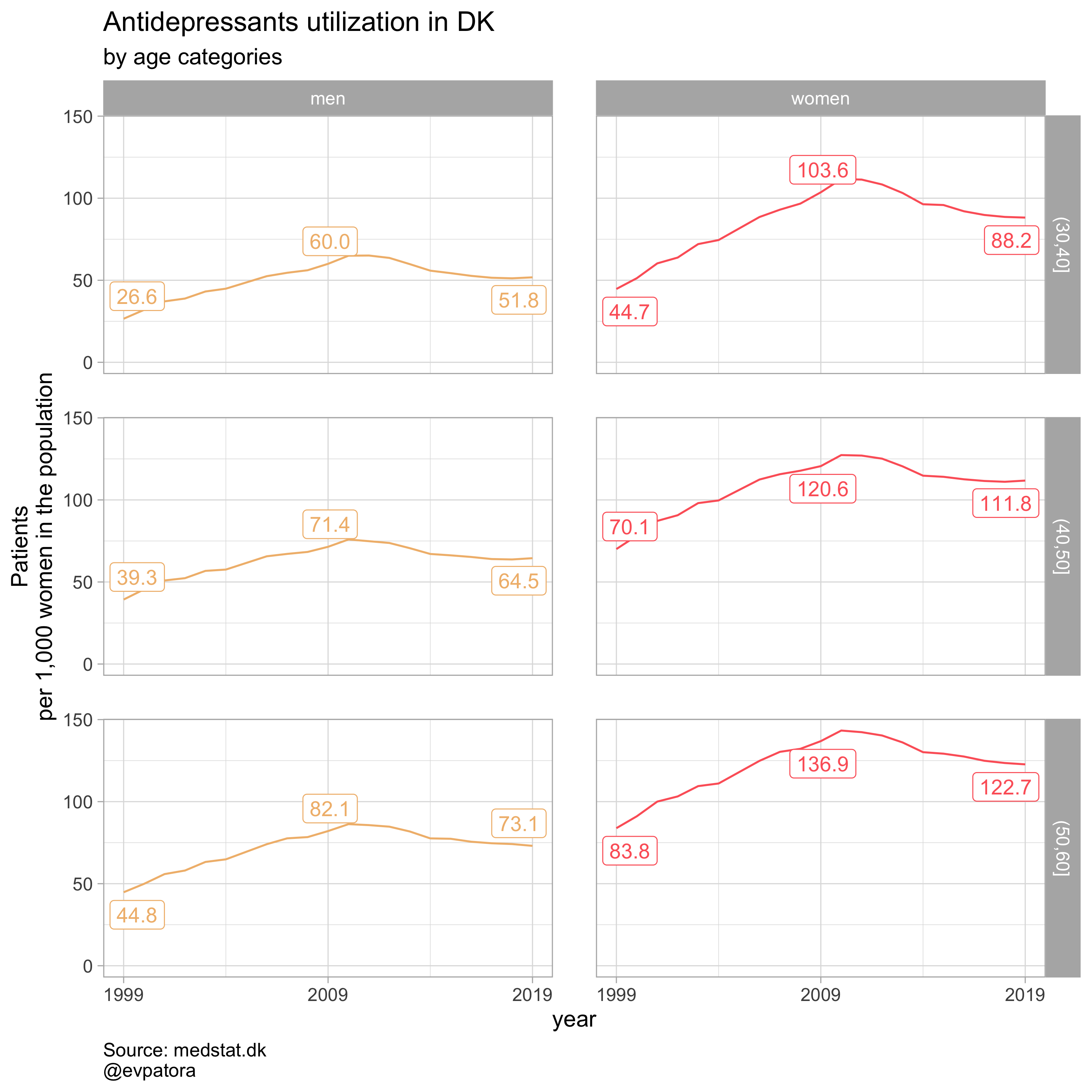##
## []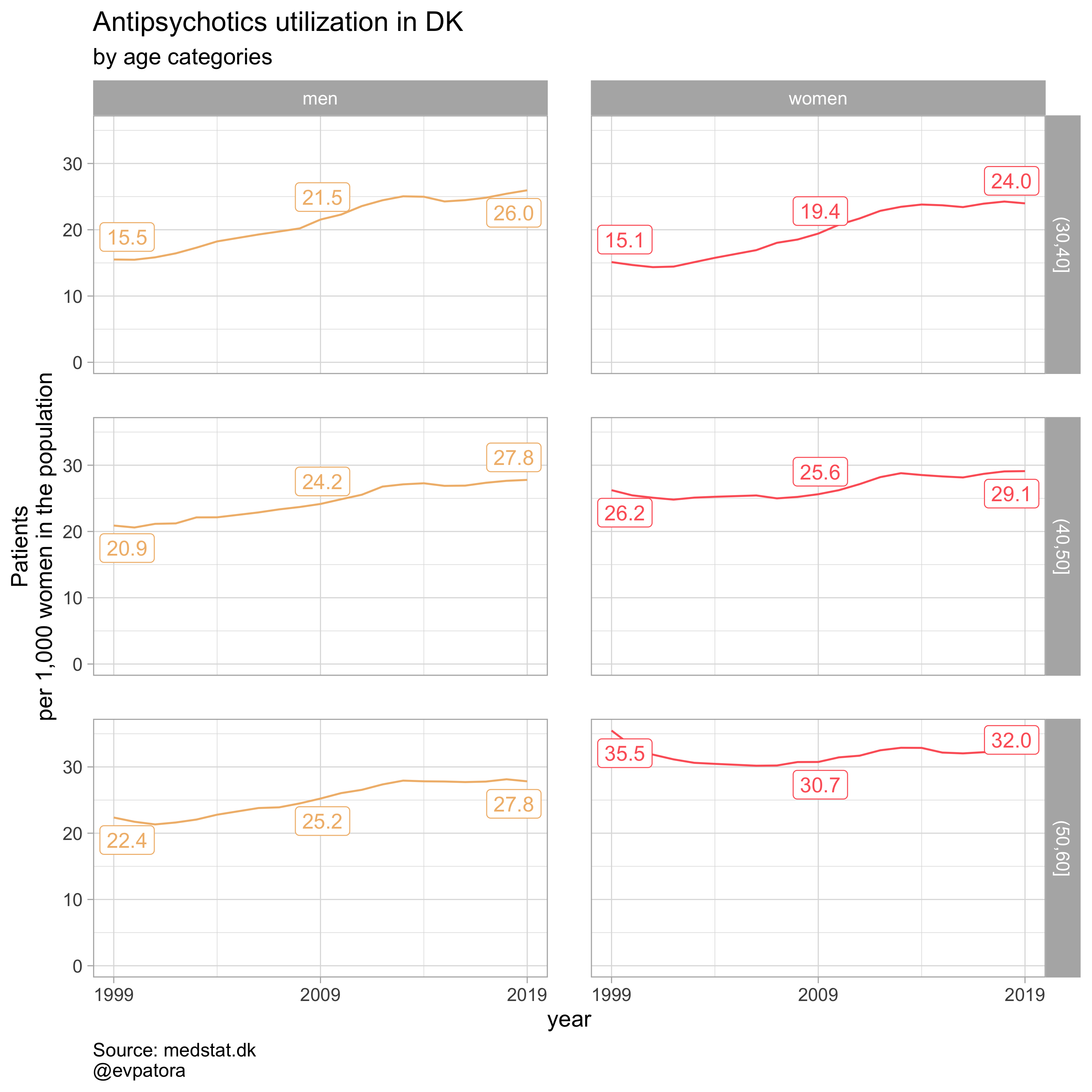##
## []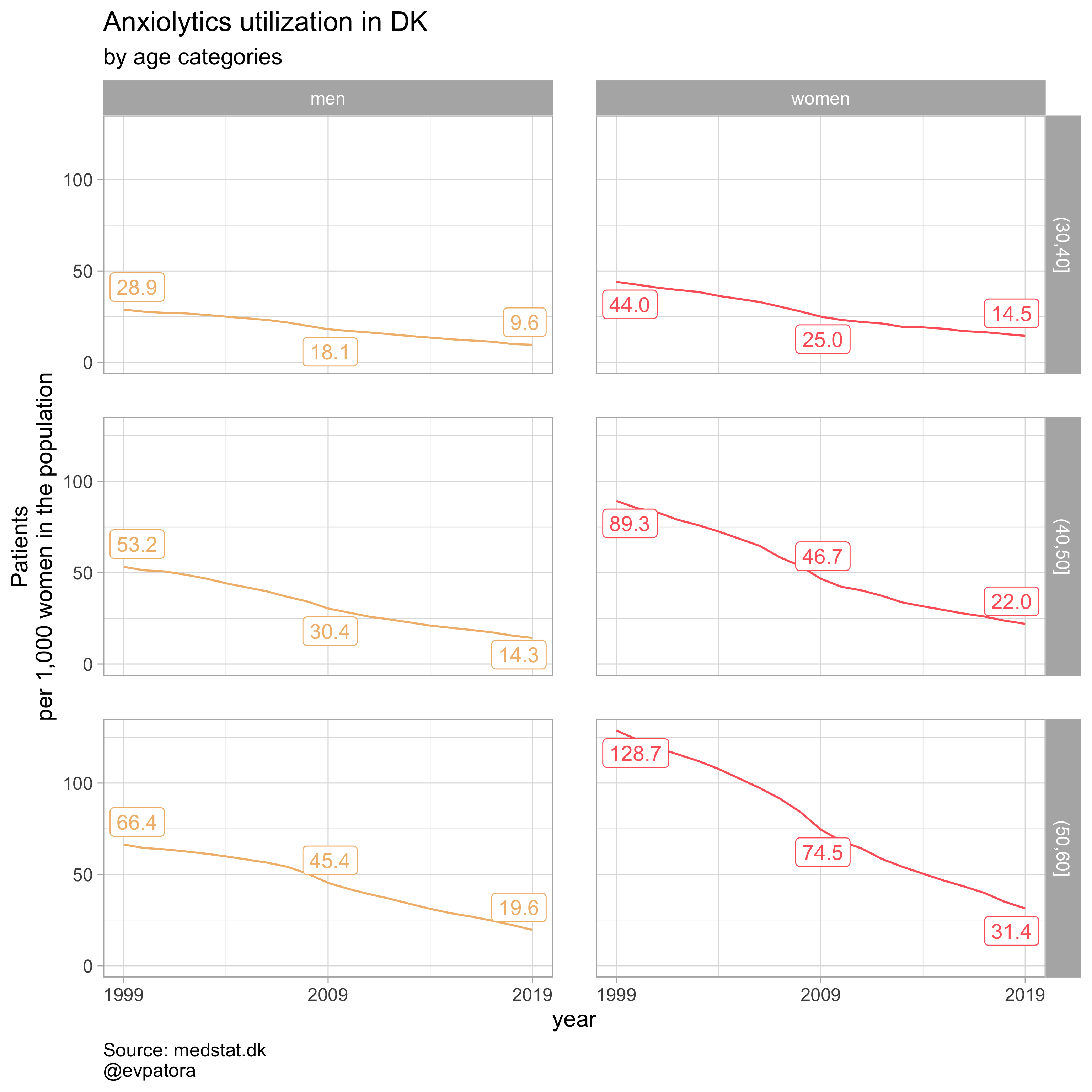##
## []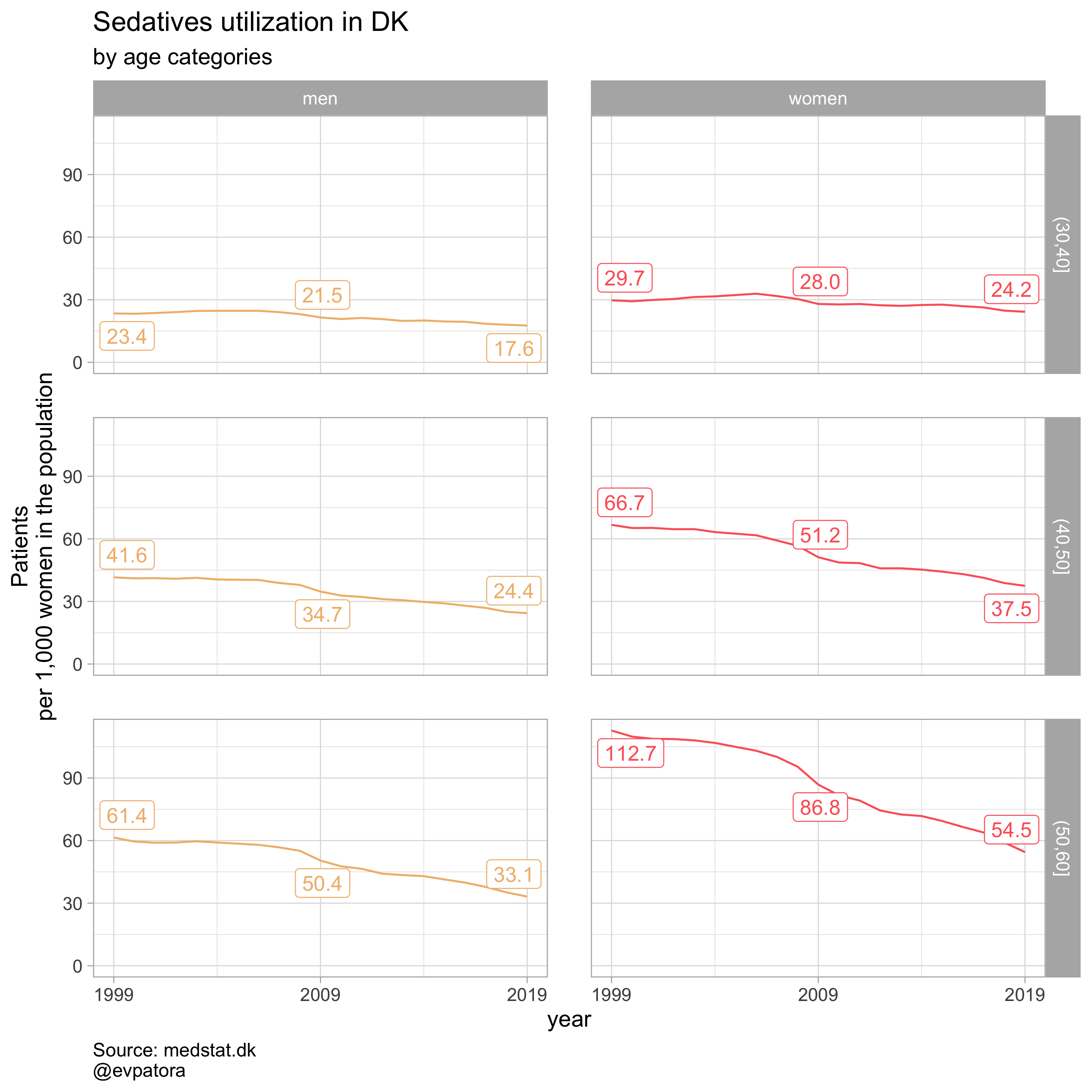# can save all plots with one line
walk2(.x = list_plots, .y = list_title, ~ggsave(filename = paste0(Sys.Date(), "-", .y, ".pdf"),
plot = .x, path = getwd(), device = cairo_pdf,
width = 297, height = 210, units = "mm"))


### Controling more than one variable in the custom function

Before we get to incorporate several more features to our customized plotting function, I want to include one intermediate step, where I will allow filtering the region column within the custom function. This will make it easier to plot a series of visualization for each of regions in addition to country-wide drug utilization rates in men and women. Note that I no longer select on specific age categories in this custom function as before.

#  selected region, now all ages
plot_utilization <- function(.my_data, drug_regex, atc, age_var, year_var, rate_var, sex_var, title, region_var, region_setting = "0"){

.my_data %>%
# I add the region_setting argument to my custom function, whose default would be a country level stats region_setting = "0"
filter({{ region_var }} == region_setting, str_detect({{ atc }}, drug_regex)) %>%
# make label for the year 2019
mutate(label = if_else({{ year_var }} == 2019, as.character(age_cat), NA_character_)) %>%
ggplot(aes(x = {{ year_var }}, y = {{ rate_var }}, color = {{ age_var }})) + # color now indicates age and not sex as before
geom_path() +
facet_grid(cols = vars({{ sex_var }}), scales = "fixed", drop = T) +
theme_light(base_size = 12) +
scale_x_continuous(limits = c(1999, 2025), breaks = c(seq(1999, 2019, 10))) +
expand_limits(y = 0) +
scale_color_manual(values = wes_palette(name = "GrandBudapest1", type = "continuous", n = 10)) +
ggrepel::geom_label_repel(aes(label = label), na.rm = TRUE, nudge_x = 4, direction = "y", segment.size = 0.1, segment.colour = "black", show.legend = F) +
theme(plot.caption = element_text(hjust = 0, size = 10),
legend.position = "none",
panel.spacing = unit(0.8, "cm")) +
labs(y = "Patients\nper 1,000 women in the population", title = paste0(title, " utilization in DK"), subtitle = "by age categories", caption = "Source: medstat.dk\n@evpatora")
}

# prepare the list to iterate along
list_regex <- list(regex_antidepress, regex_antipsych, regex_anxiolyt, regex_sedat)
list_title <- list("Antidepressants", "Antipsychotics", "Anxiolytics", "Sedatives")

# iteration
list_plots <- map2(list_regex, list_title,
~plot_utilization(.my_data = data, atc = ATC, drug_regex = ..1,
age_var = age_cat, year_var = year, rate_var = patients_per_1000_inhabitants,
sex_var = gender_text, title = ..2, region_var = region))

list_plots

## []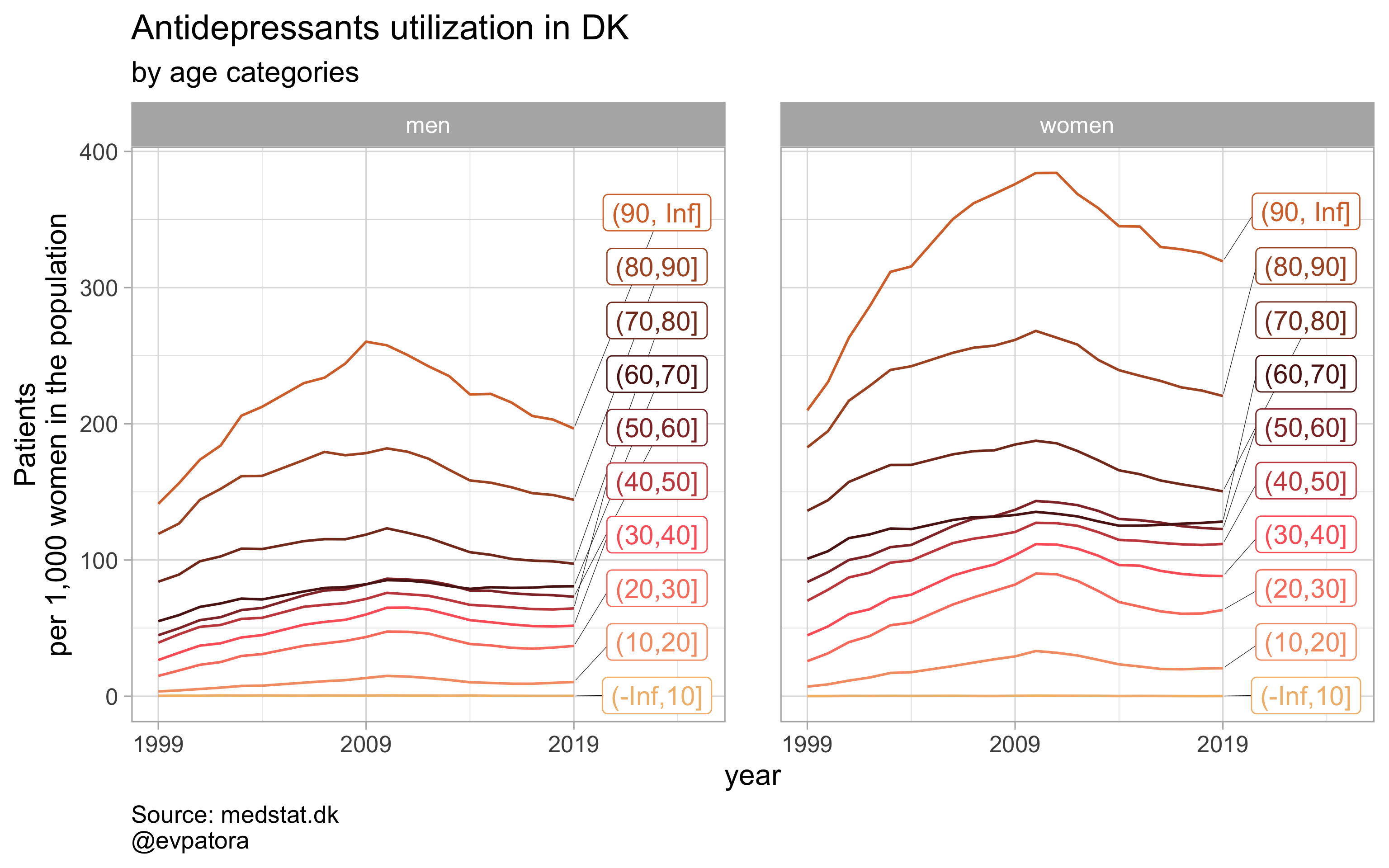##
## []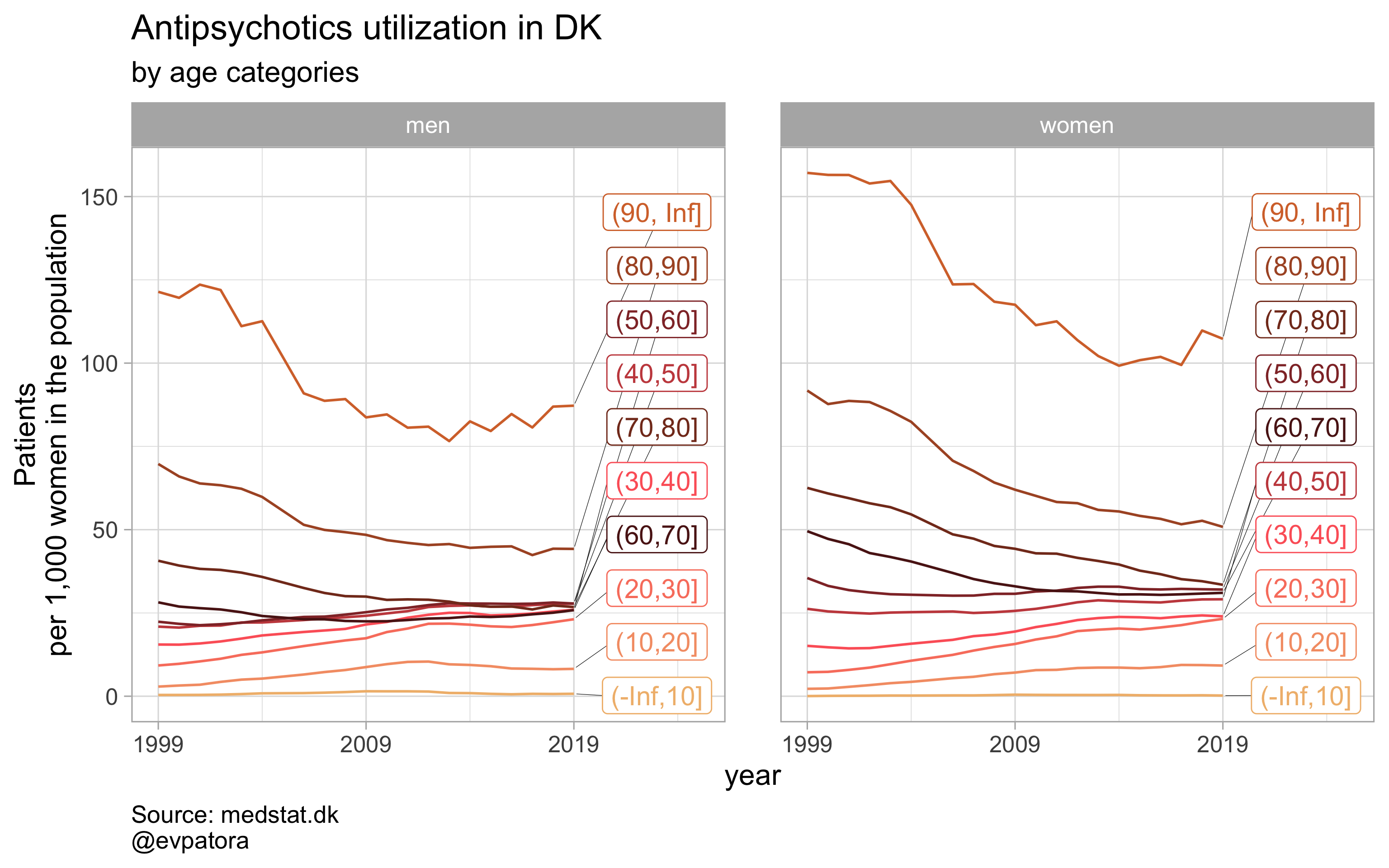##
## []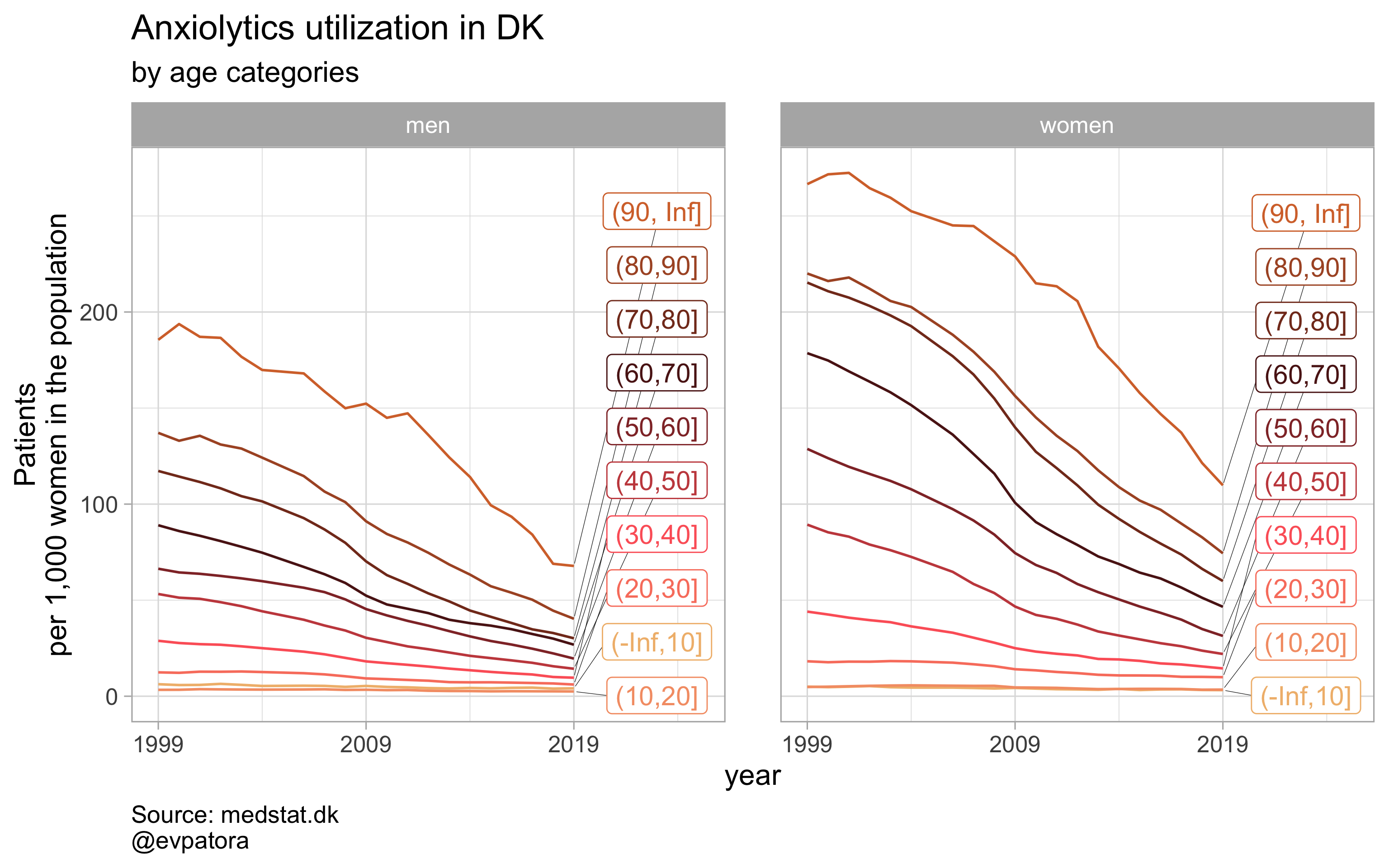##
## []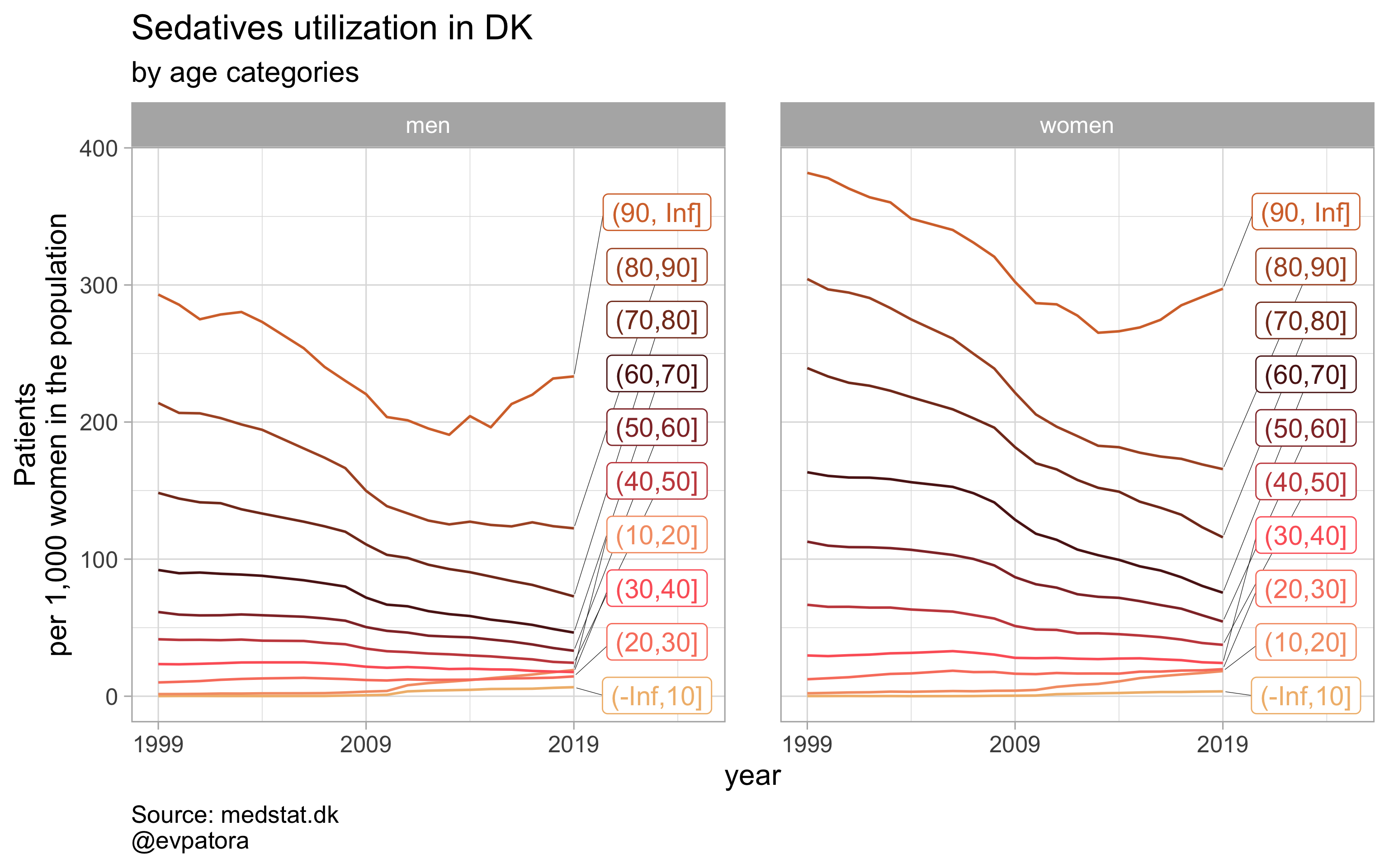# save all graphs with one line
walk2(.x = list_plots, .y = list_title, ~ggsave(filename = paste0(Sys.Date(), "-", .y, "_Syddanmark.pdf"),
plot = .x, path = getwd(), device = cairo_pdf,
width = 297, height = 210, units = "mm"))


### Finalize customization based on your needs

Now, I want to have control over age groups to be visualized. I will add a new argument age_setting into my custom function to implement this. Depending on what is my final goal, I can make a finalized function:

1. For the visualization of selected drugs utilization rates in women vs men by age category explicitly (as many facets as selected age groups) or
2. For visualization of selected drugs utilization rates in women vs men by age category implicitly (faceting data for women vs men and making no facets for age categories).
3. For visualization of selected drugs utilization rates in women vs men by age category and by region on the same graph

### Option 1

NB! Some regions seem to miss data and in options 1 and 2 I do not explicitly exclude such regions

# for all regions & for selected age categories
plot_utilization <- function(.my_data, drug_regex, atc, age_var, year_var, rate_var, sex_var, title, region_var, region_setting = "0", age_numeric, age_setting){

.my_data %>%
filter({{ age_numeric }} %in% age_setting, {{ region_var }} == region_setting, str_detect({{ atc }}, drug_regex)) %>%
mutate(
label = if_else({{ year_var }} == 1999 | {{ year_var }} == 2009 | {{ year_var }} == 2019, as.character(sprintf("%1.1f", round({{ rate_var }}, digits = 1))), NA_character_)
) %>%
ggplot(aes(x = {{ year_var }}, y = {{ rate_var }}, color = {{ sex_var }})) +
geom_path() +
facet_grid(cols = vars({{ sex_var }}), rows = vars({{ age_var }}), scales = "free", drop = T) +
theme_light(base_size = 12) +
scale_x_continuous(breaks = c(seq(1999, 2019, 10))) +
expand_limits(y = 0) +
ggrepel::geom_label_repel(aes(label = label), na.rm = TRUE, nudge_x = 0.1, direction = "y", segment.size = 0.1, segment.colour = "black", show.legend = F) +
theme(plot.caption = element_text(hjust = 0, size = 10),
legend.position = "none",
panel.spacing = unit(0.8, "cm")) +
scale_color_manual(values = wes_palette(name = "GrandBudapest1", type = "discrete")) +
theme(plot.caption = element_text(hjust = 0, size = 10),
legend.position = "none",
panel.spacing = unit(0.8, "cm")) +
labs(y = "Patients\nper 1,000 women in the population", title = paste0(title, " utilization in DK"), subtitle = "by age categories", caption = "Source: medstat.dk\n@evpatora\n")
}

# 4 drugs for 5 regions = 20 elements
list_region <- list(1:5) %>% map(~as.character(.x)) %>% rep(times = 4) %>% flatten()
list_regex <- list(regex_antidepress, regex_antipsych, regex_anxiolyt, regex_sedat) %>% rep(each = 5)
list_drug_name <- list("Antidepressants", "Antipsychotics", "Anxiolytics", "Sedatives") %>% rep(each = 5)
list_title <- map2(list_region, list_drug_name, ~paste0("Region=", .x, ", ", .y))

# iteration
list_plots <- pmap(.l = list(list_regex, list_title, list_region),
.f = ~plot_utilization(.my_data = data, atc = ATC, drug_regex = ..1,
age_var = age_cat, year_var = year, rate_var = patients_per_1000_inhabitants,
sex_var = gender_text, title = ..2, region_var = region, region_setting = ..3,
age_numeric = age, age_setting = 10:60))

# see some results
list_plots

## []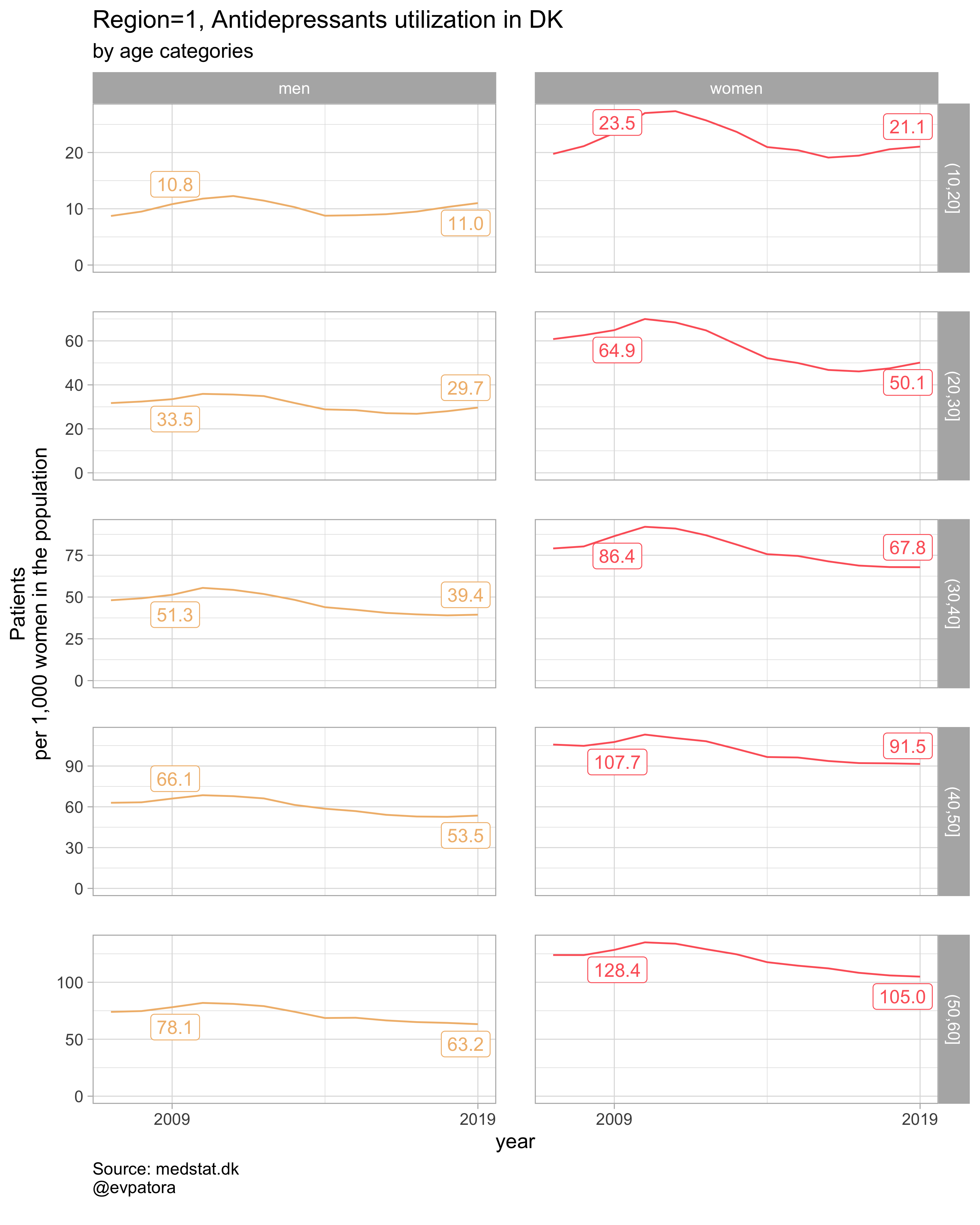##
## []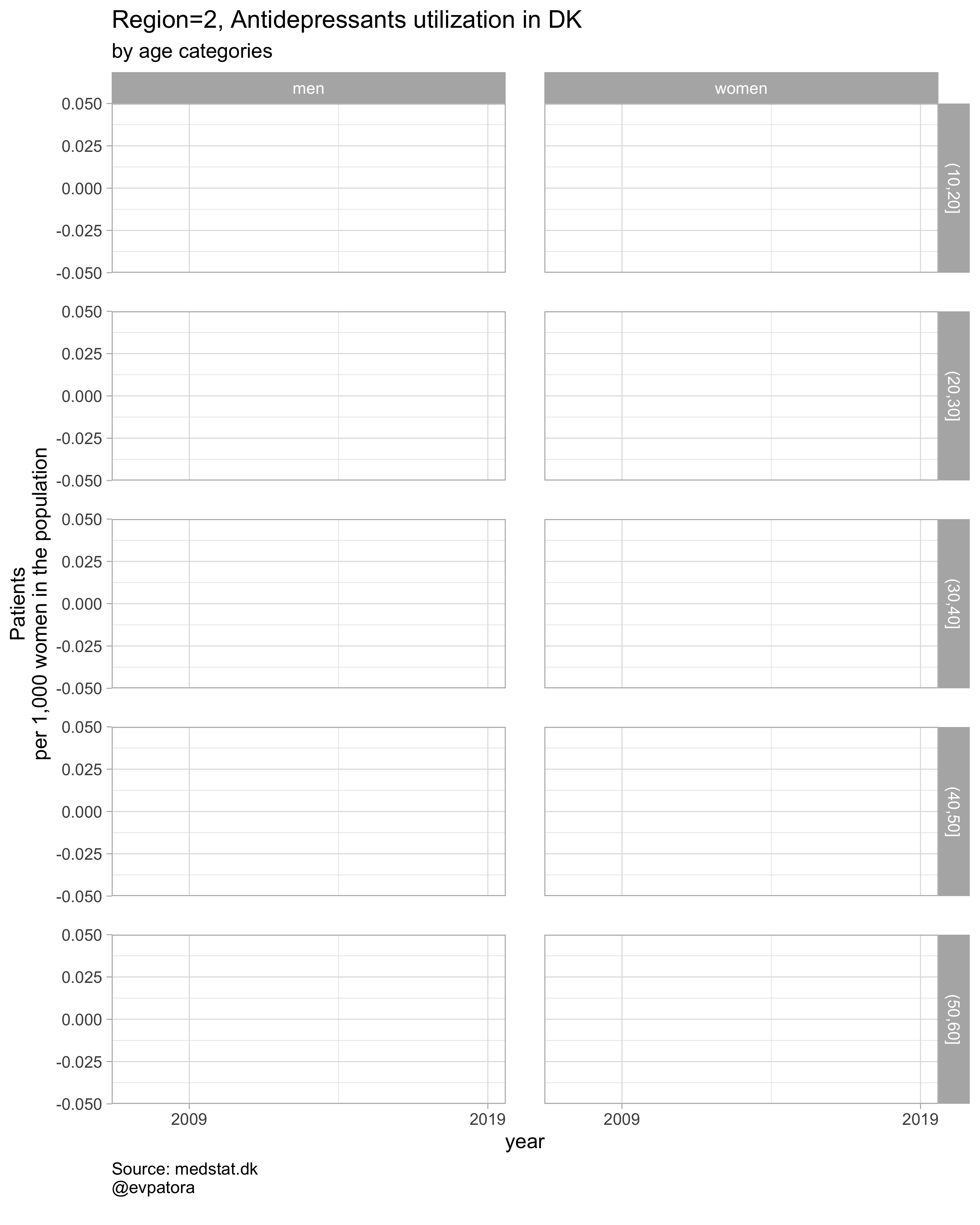##
## []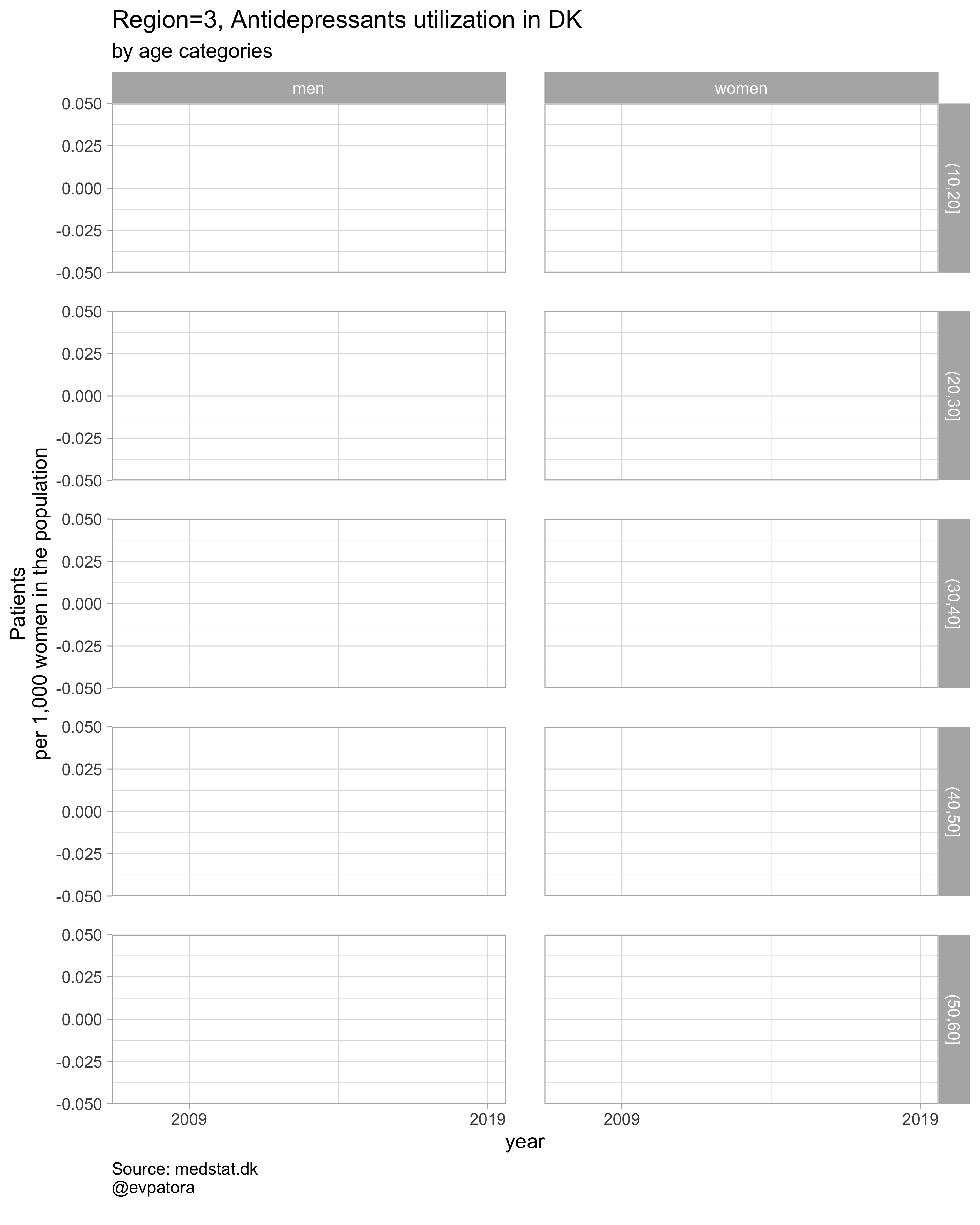##
## []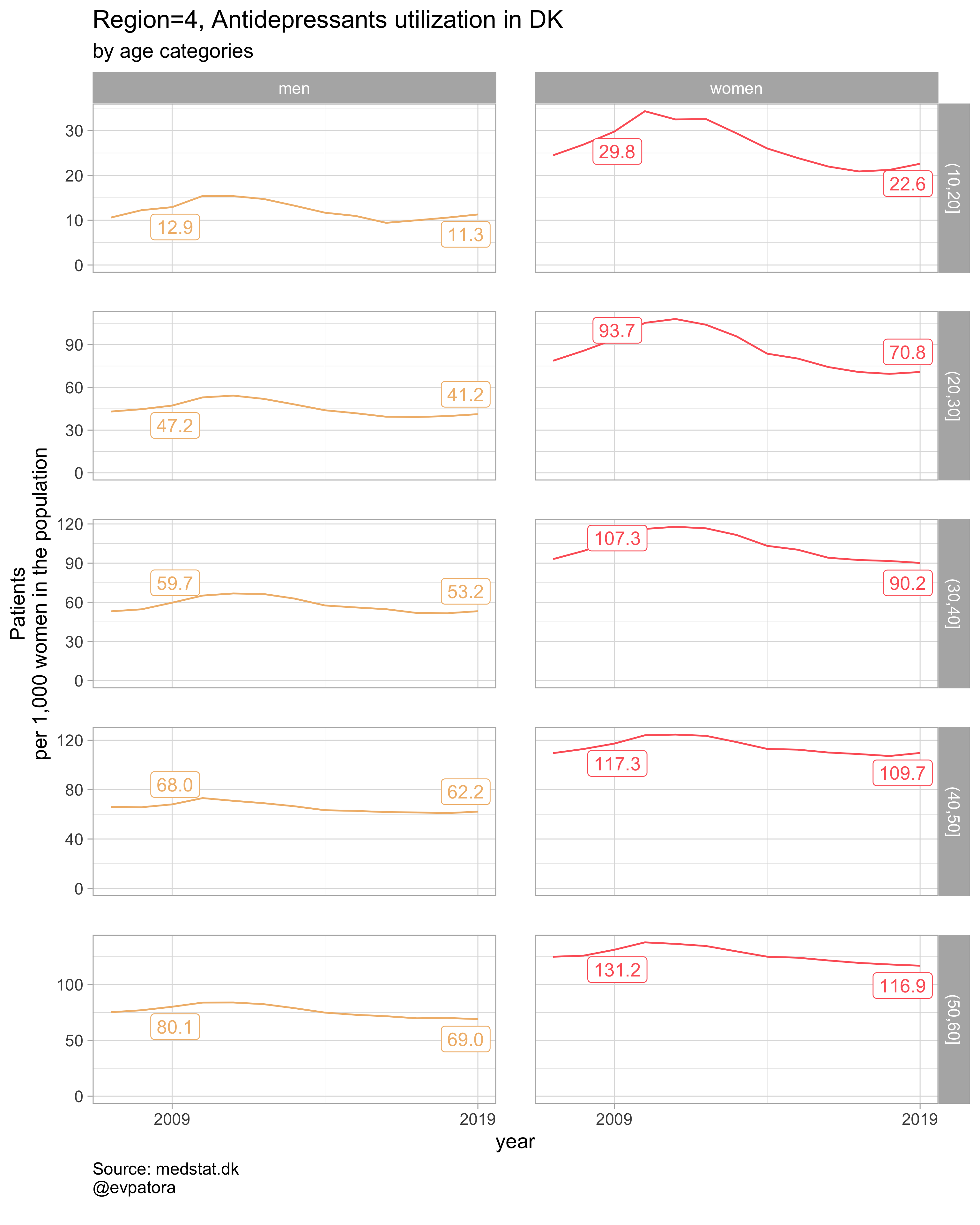##
## []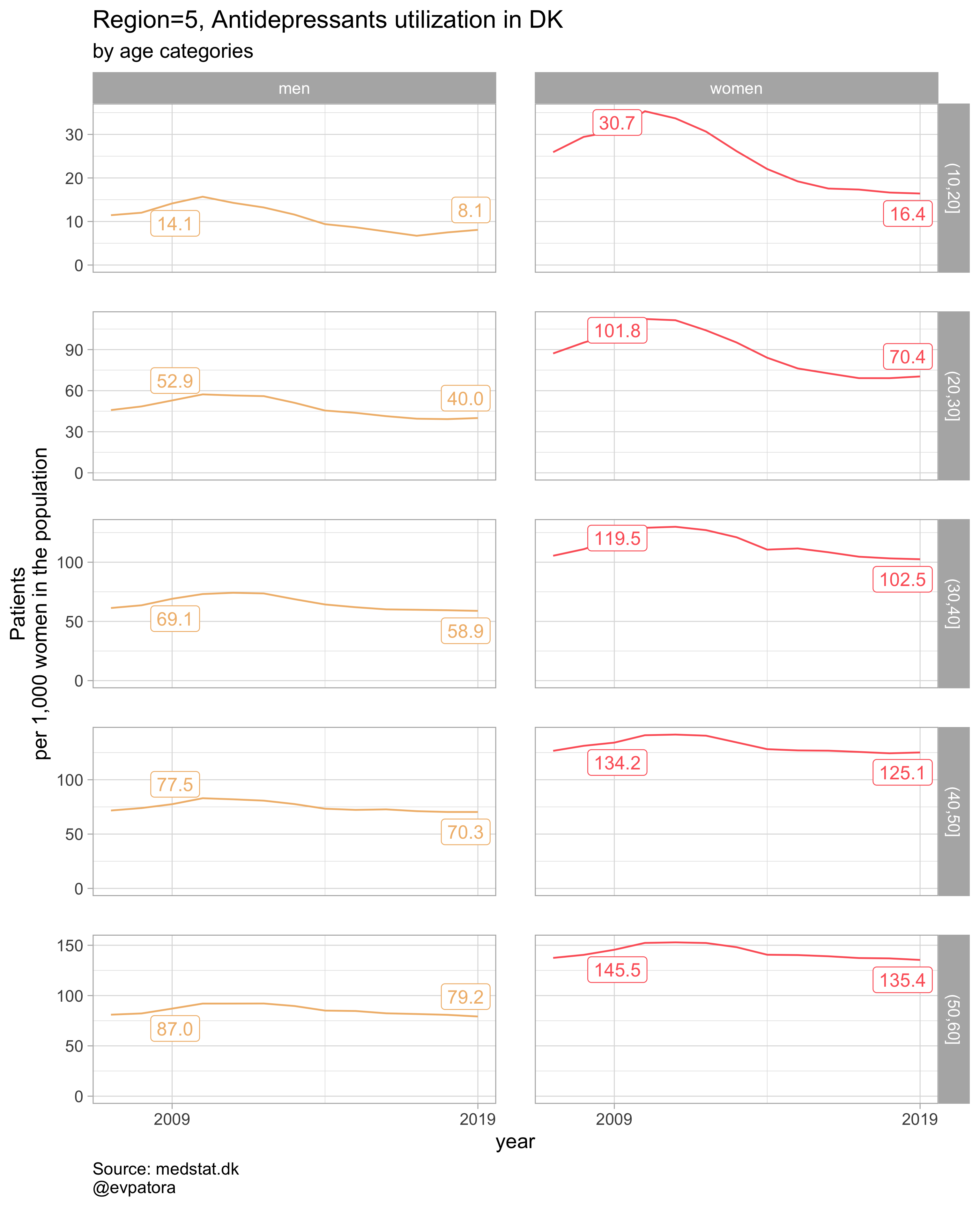##
## []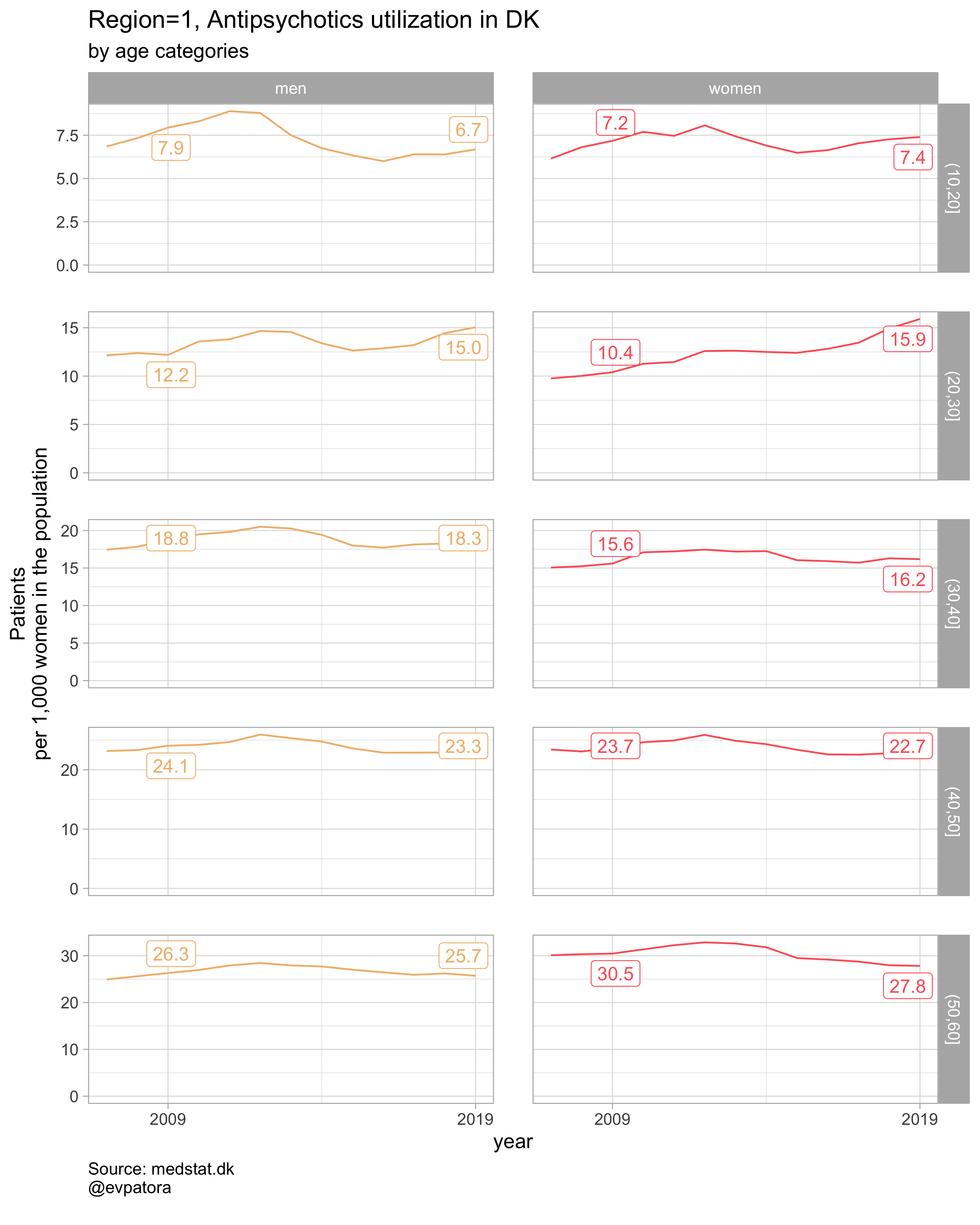##
## []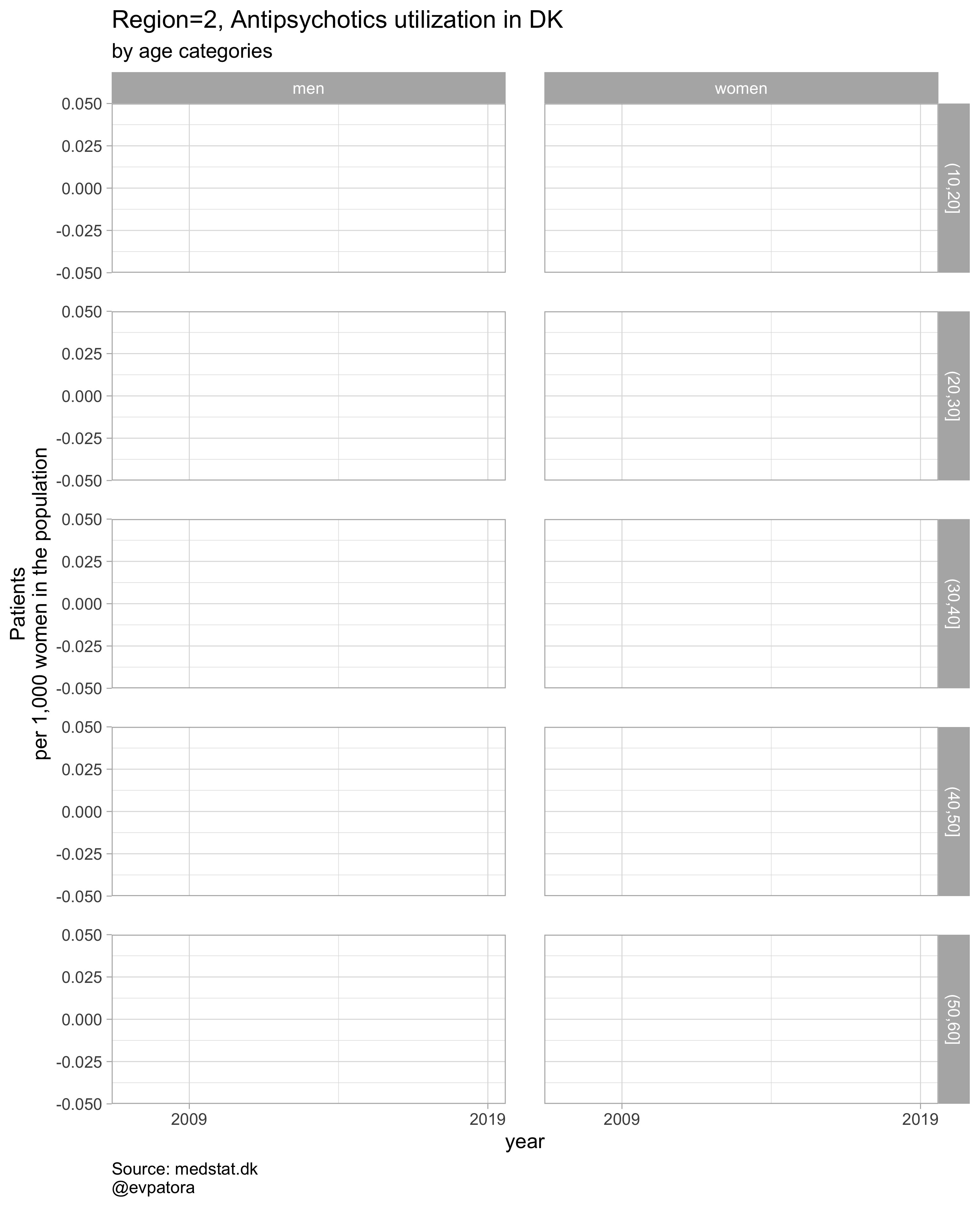##
## []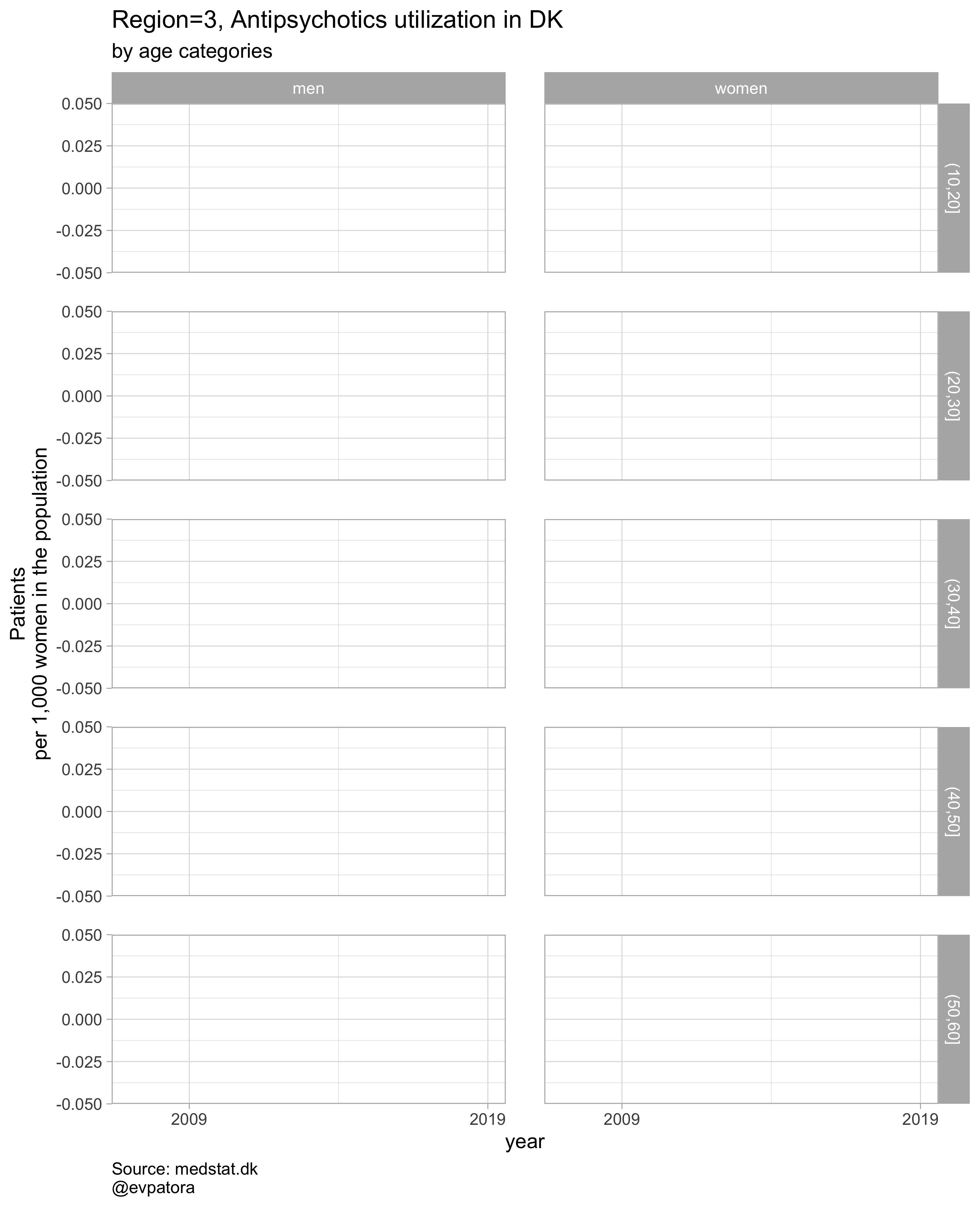##
## []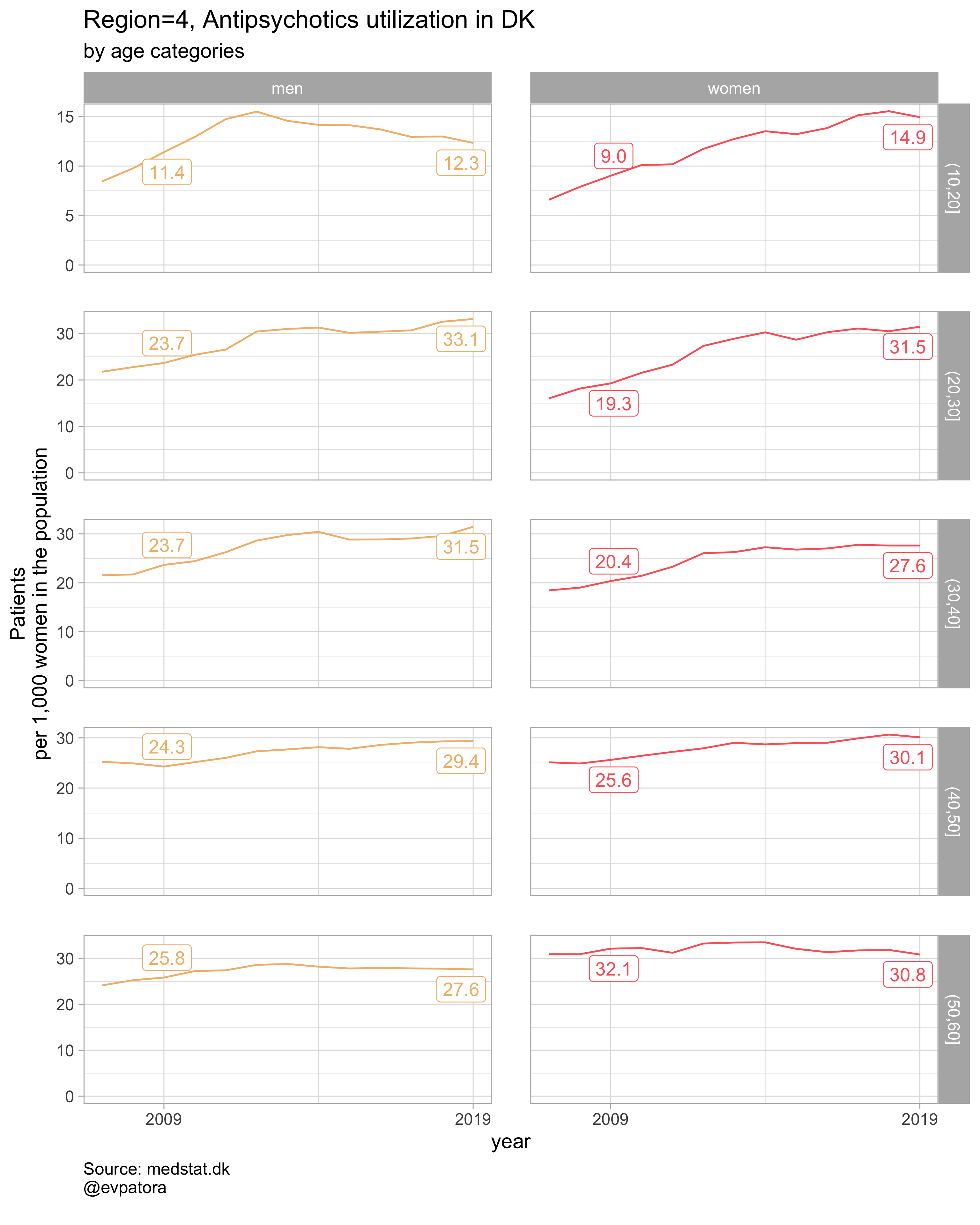##
## []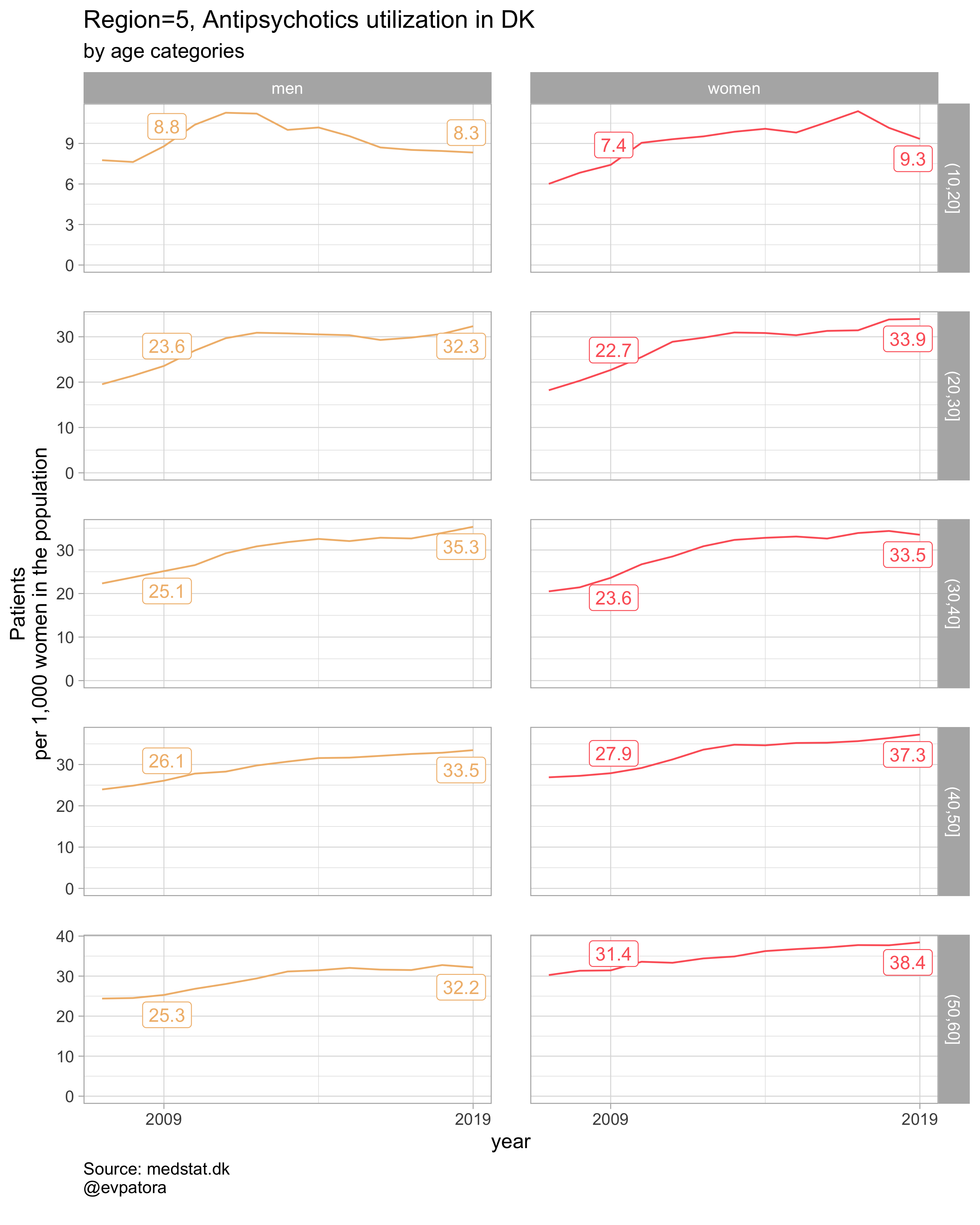##
## []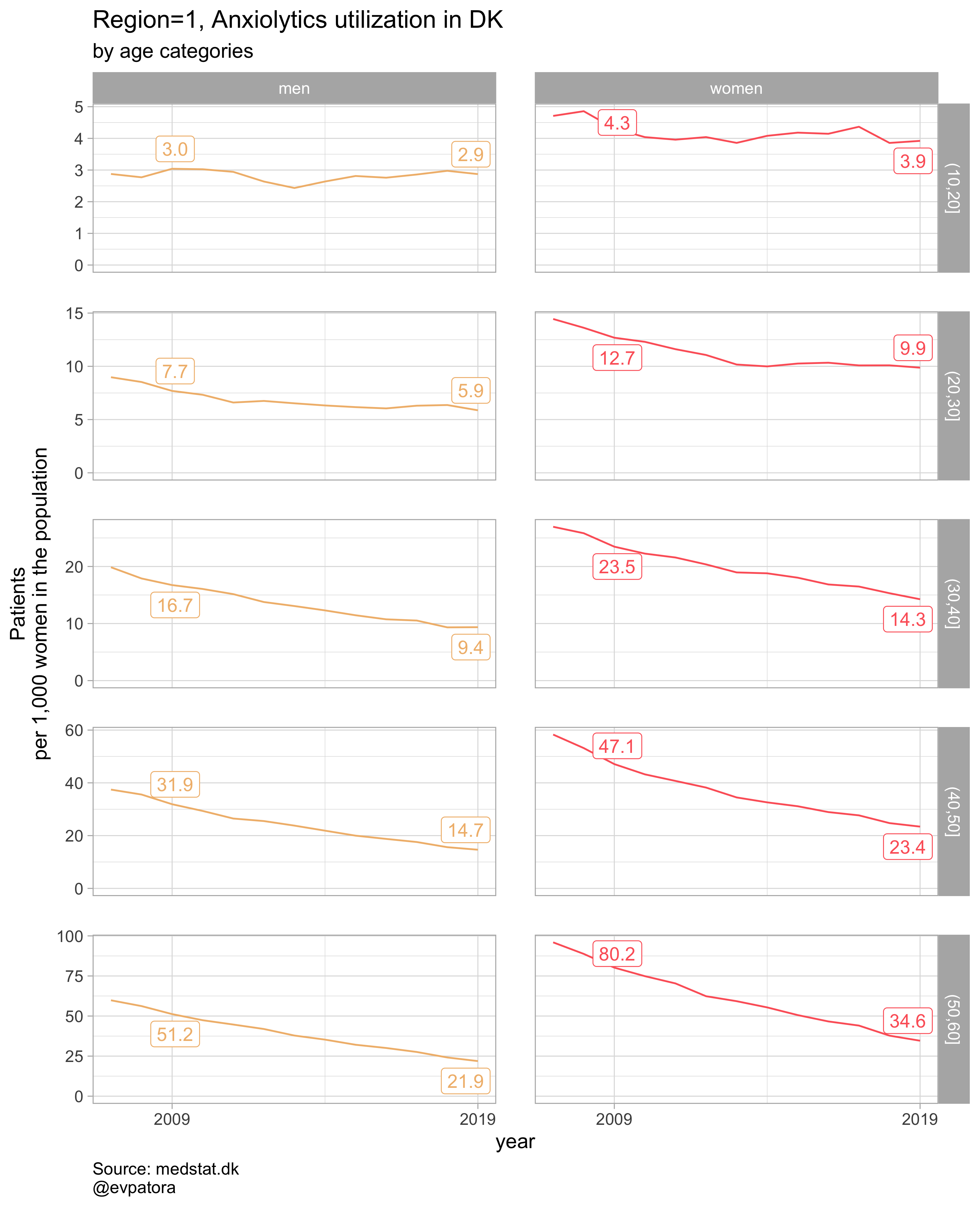##
## []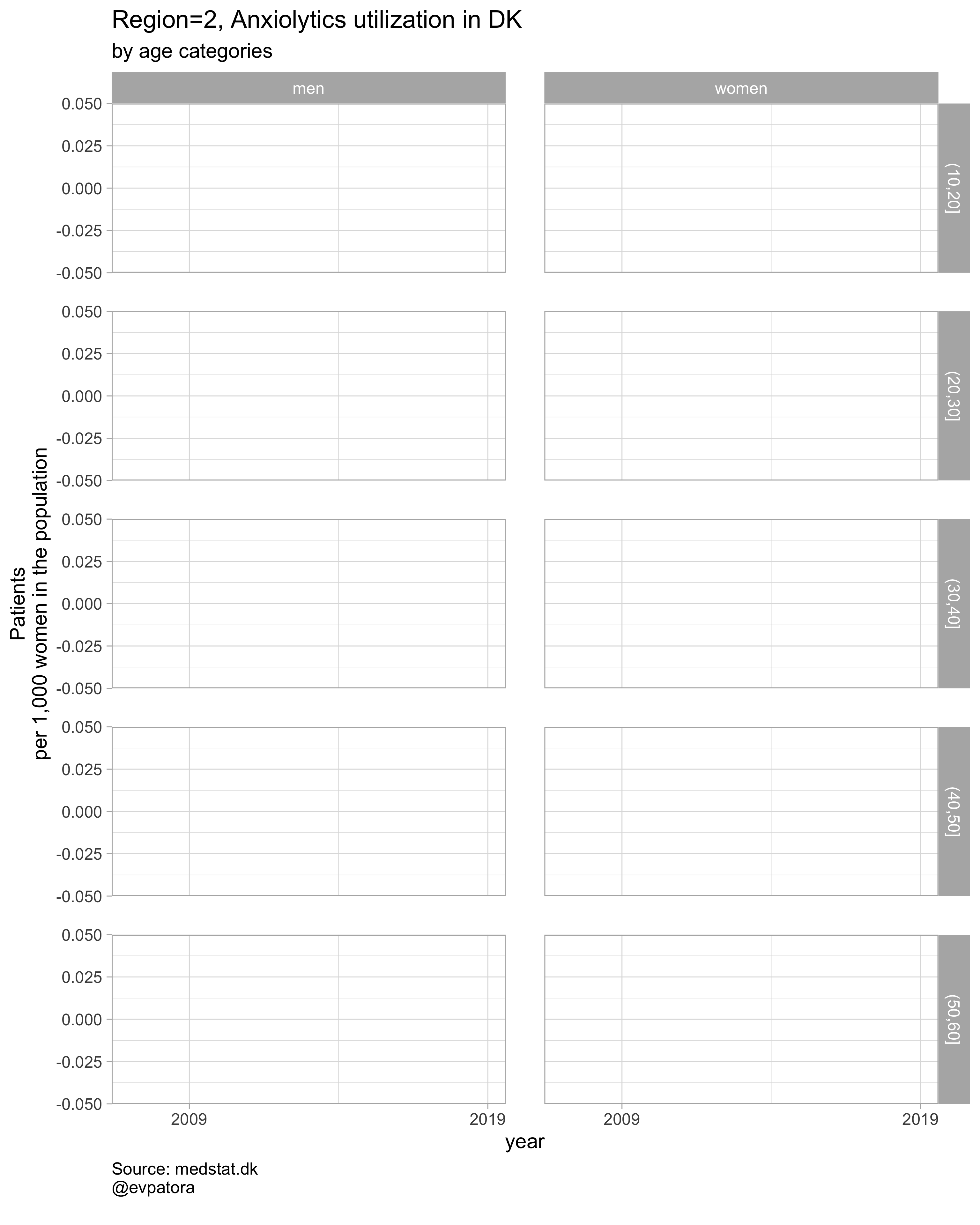##
## []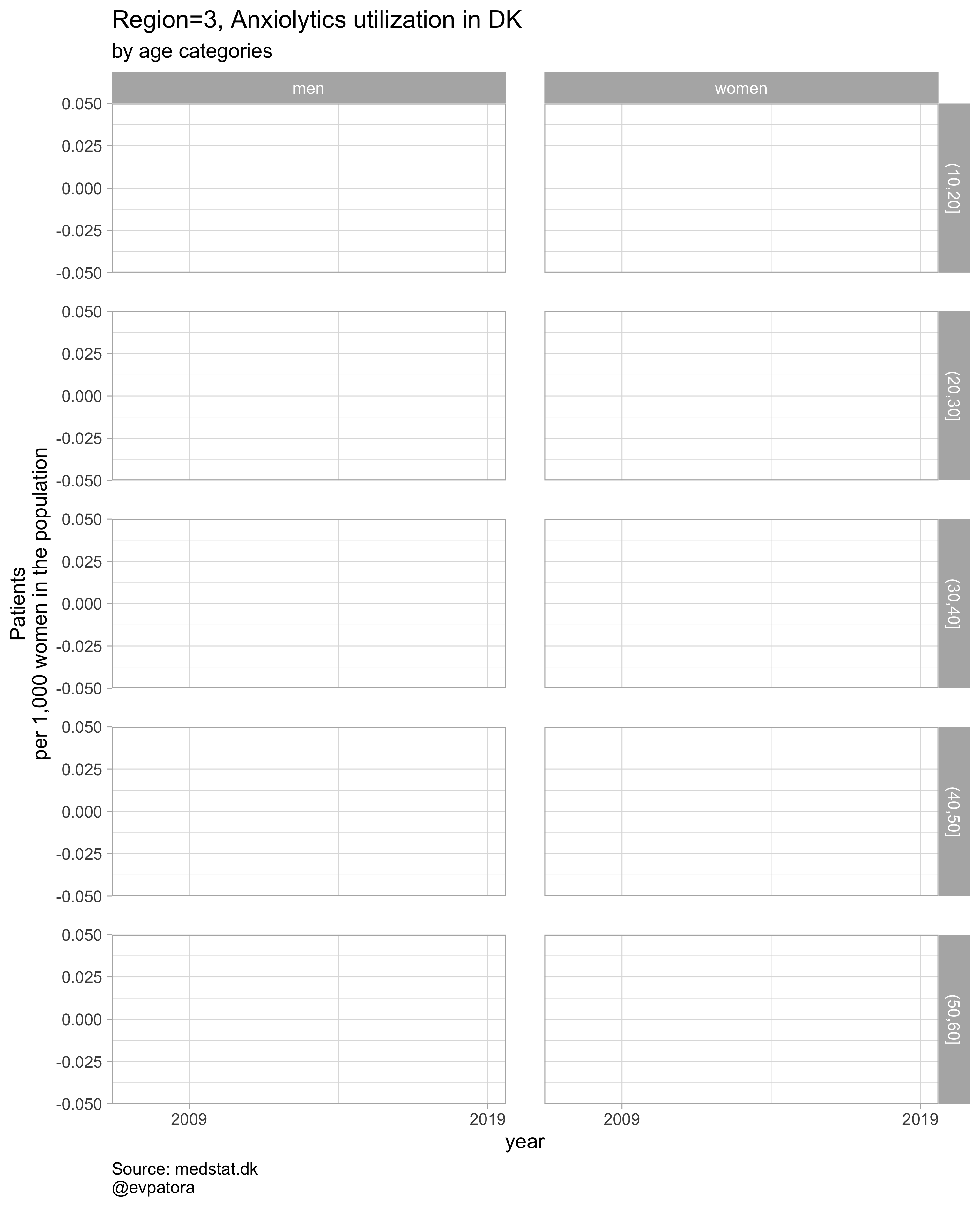##
## []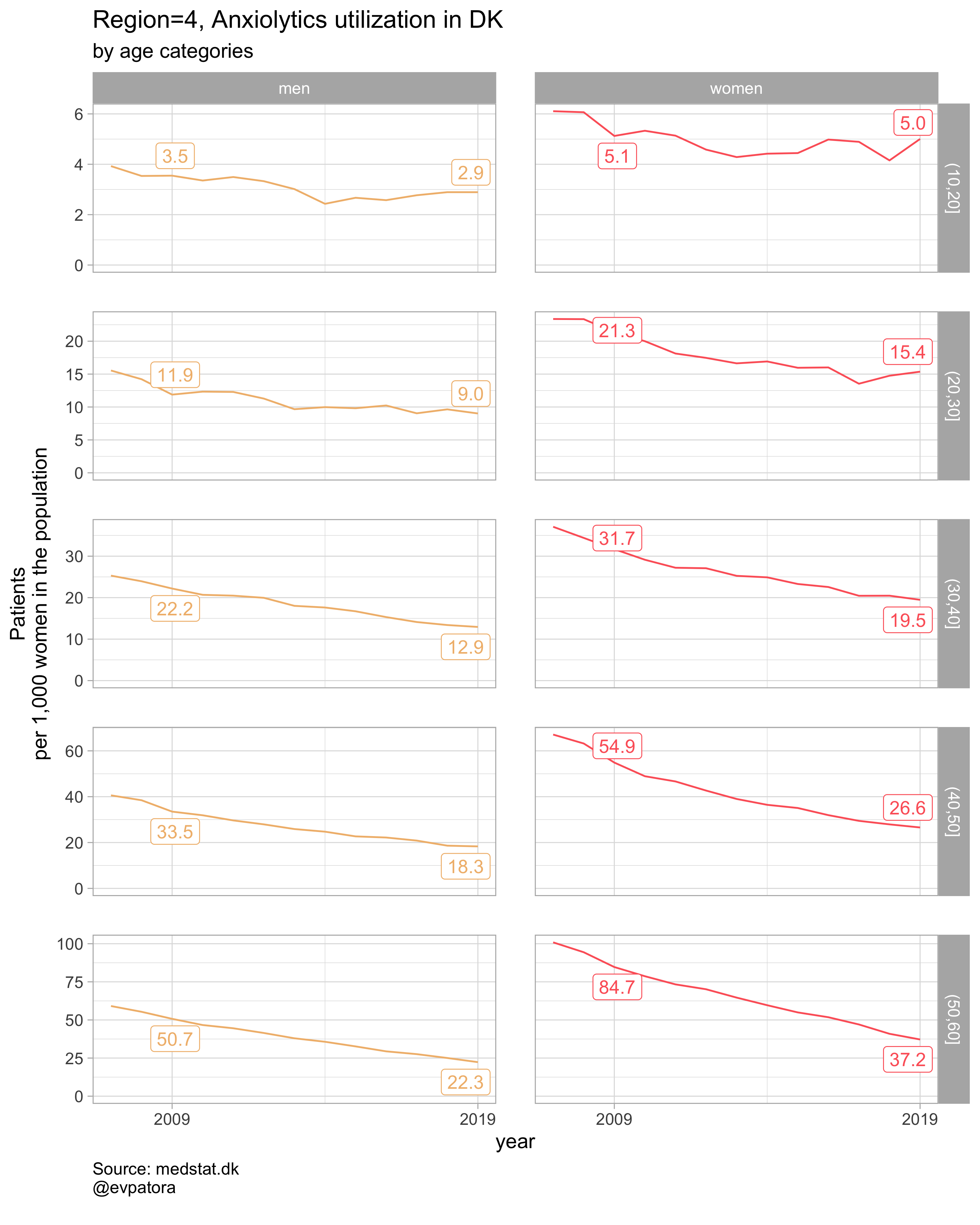##
## []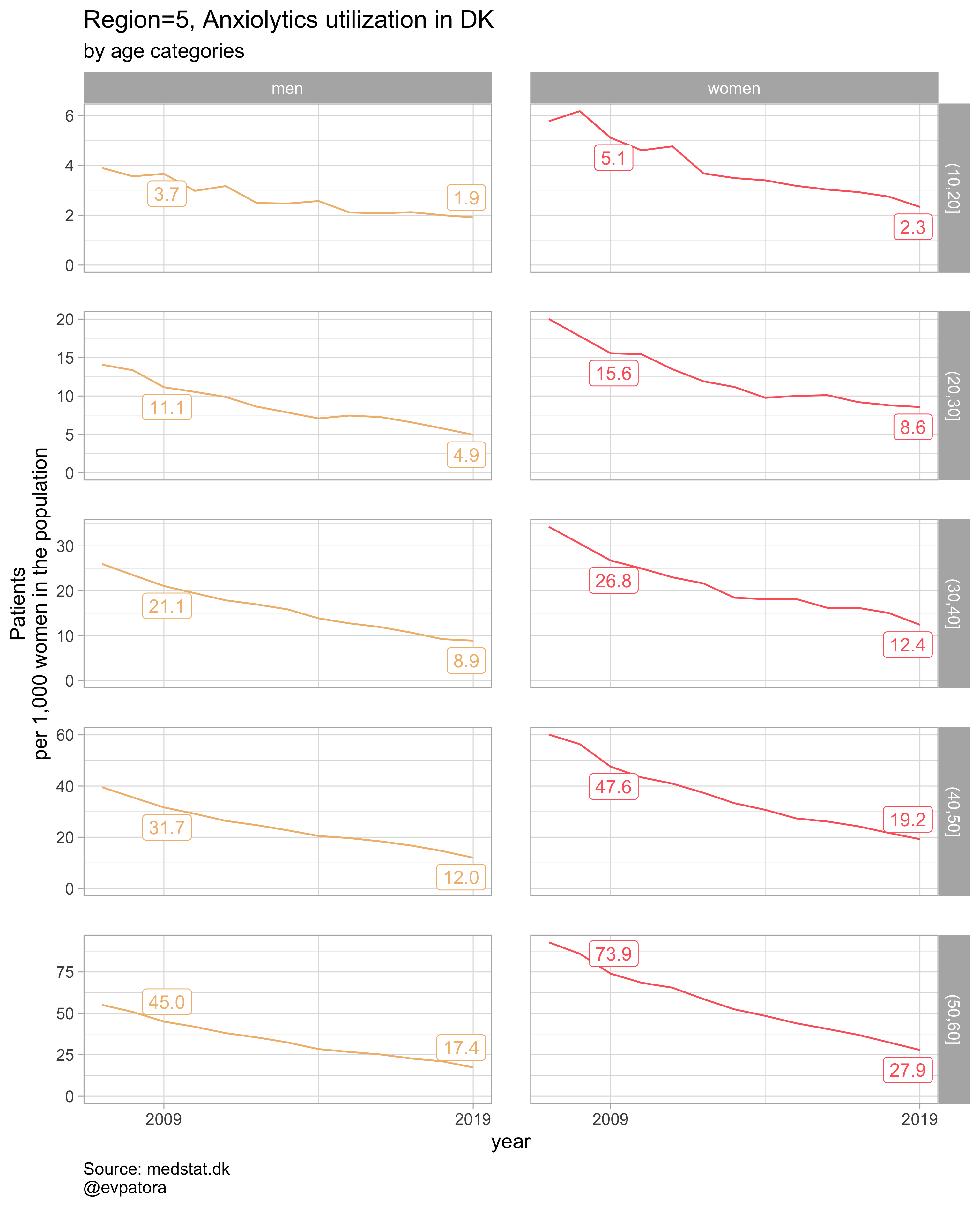##
## []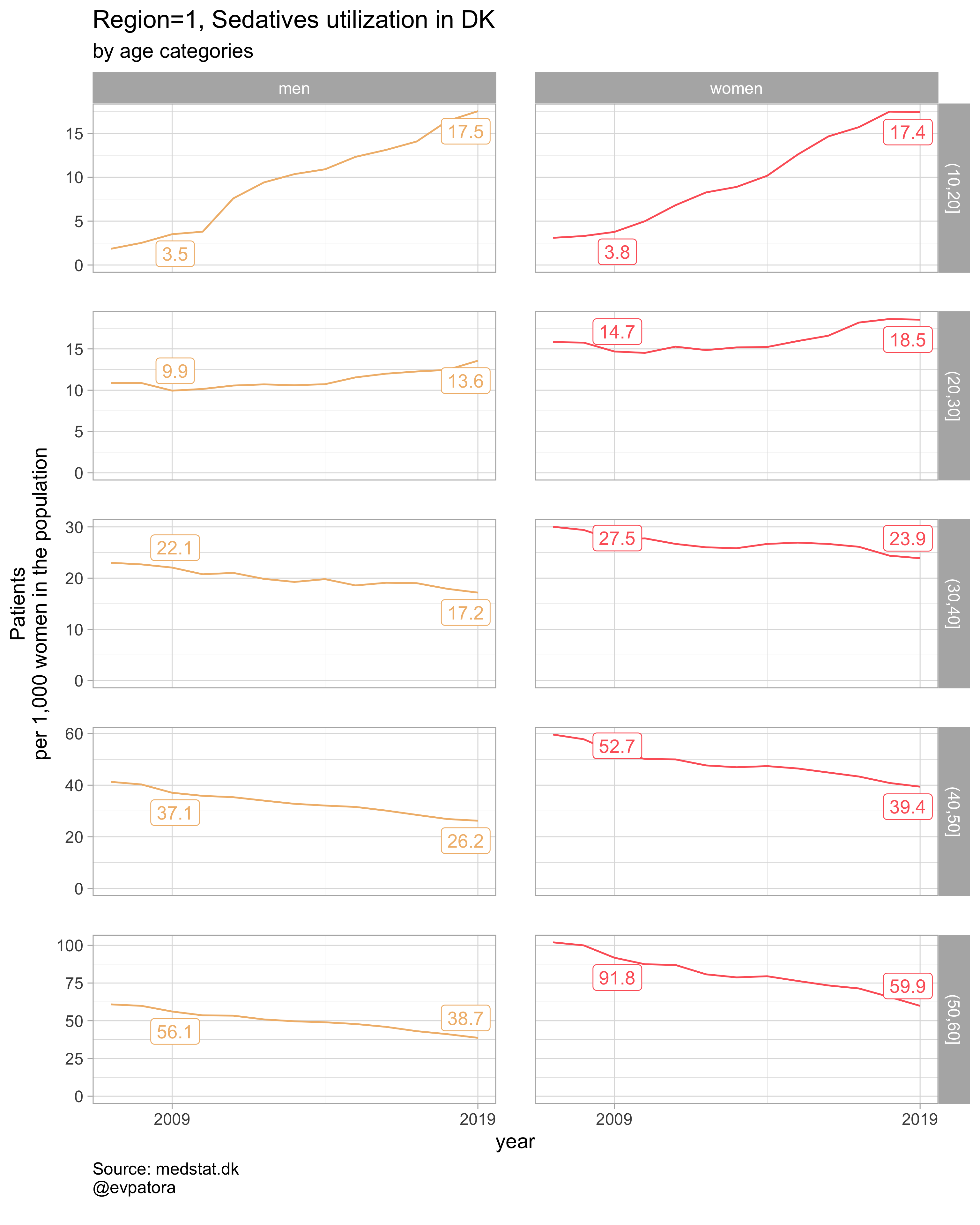##
## []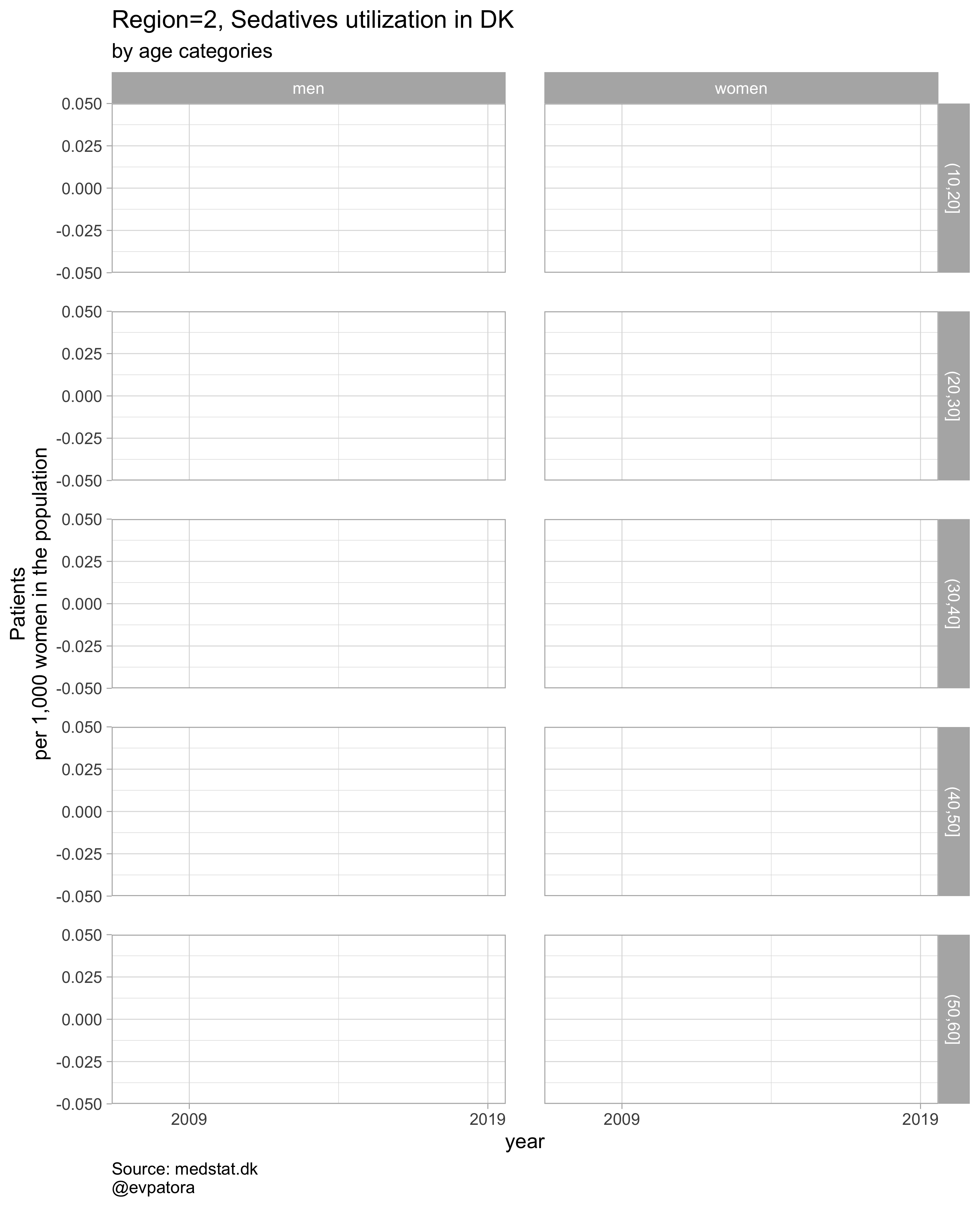##
## []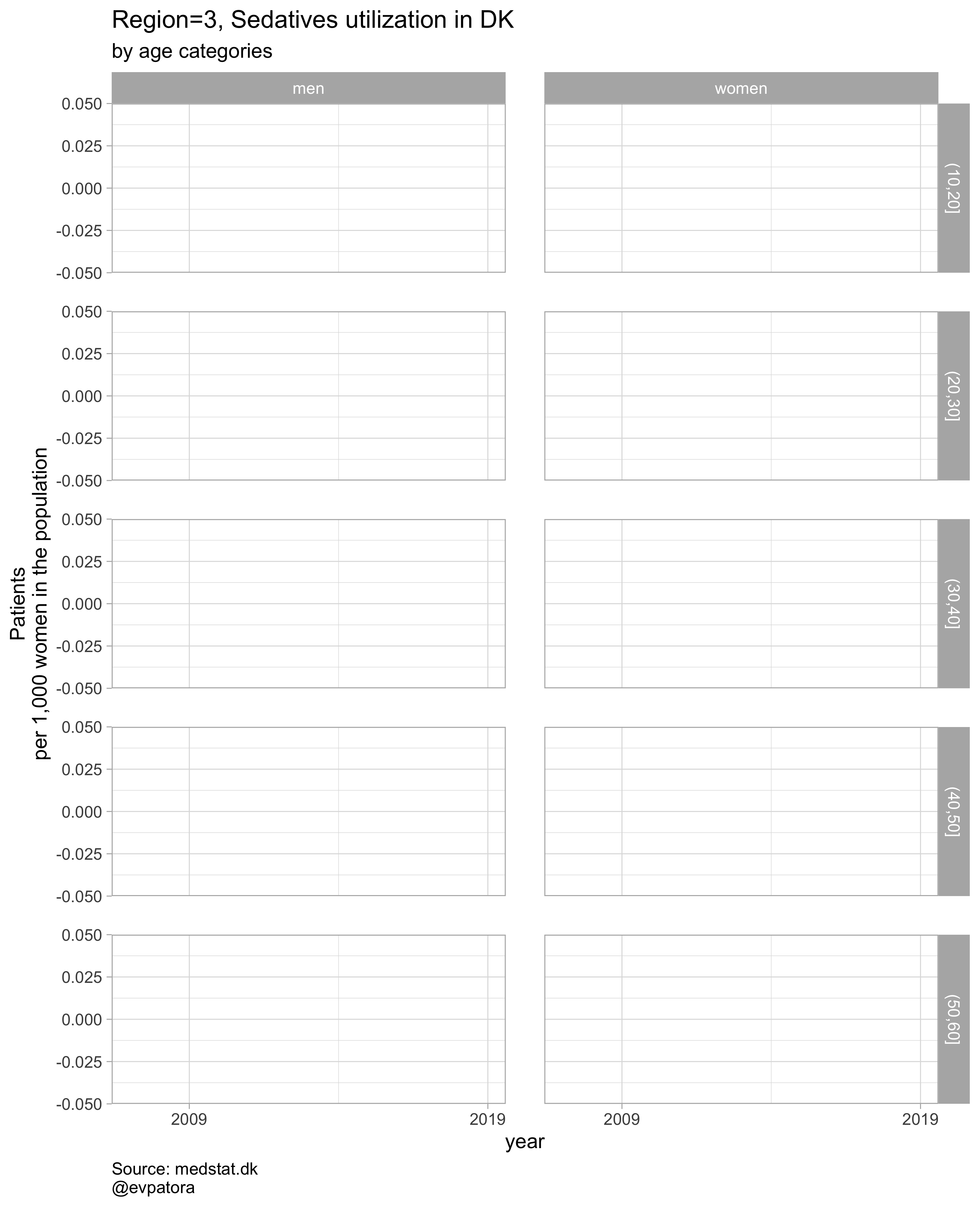##
## []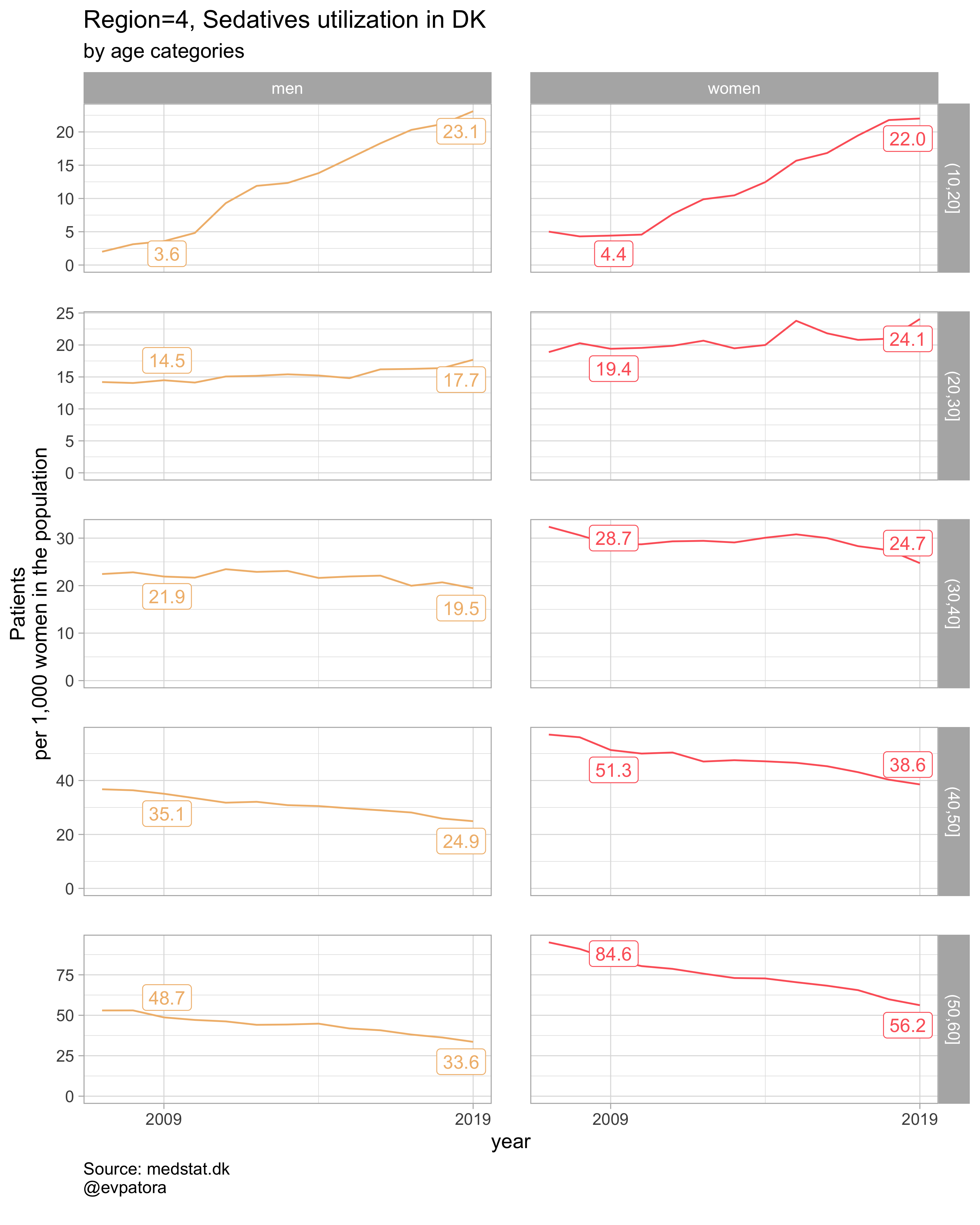##
## []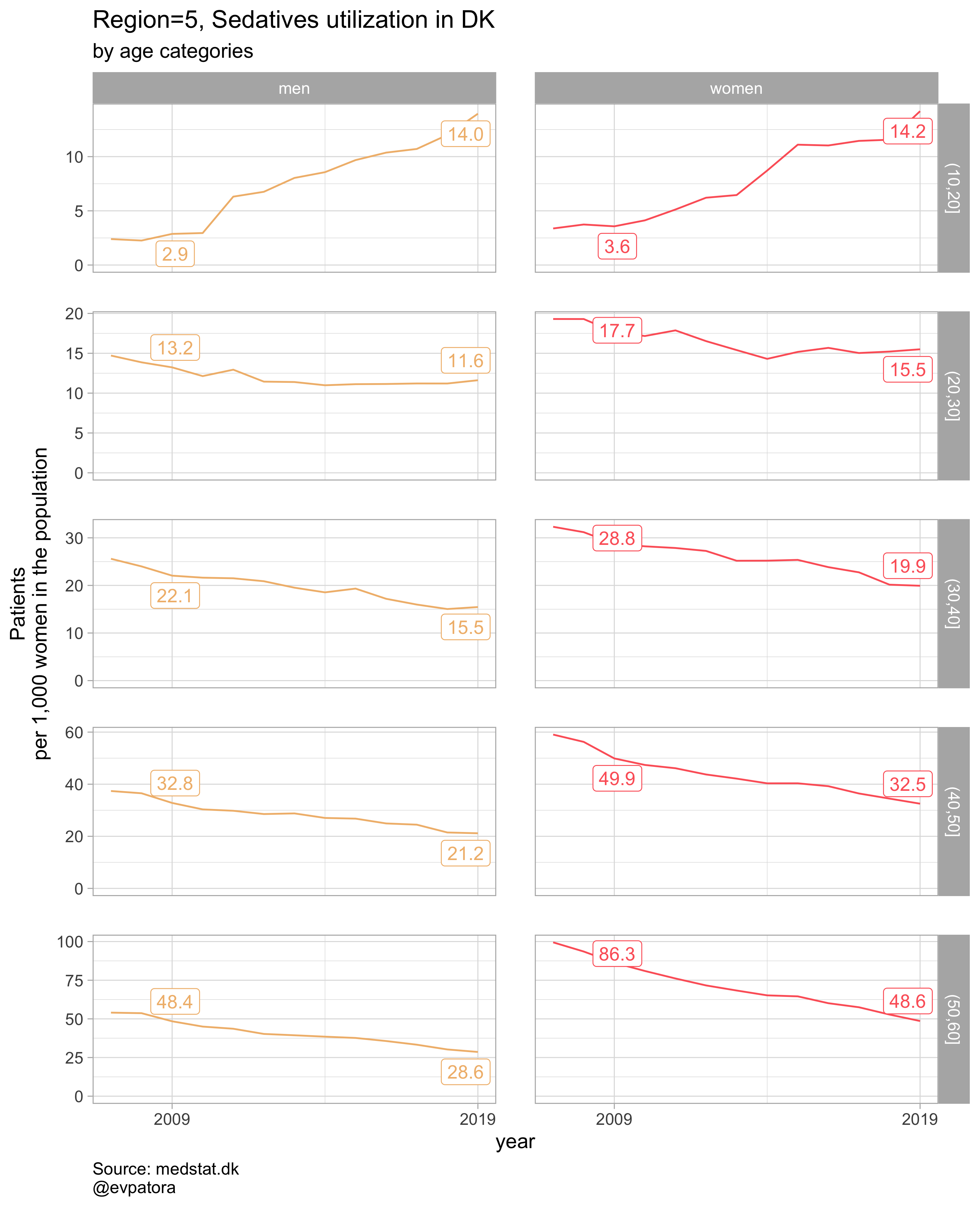# save all plots with one line: each saved plot has the name of the parameters plotted (medication & region)
walk2(.x = list_plots, .y = list_title, ~ggsave(filename = paste0(Sys.Date(), "-", .y, ".pdf"),
plot = .x, path = getwd(), device = cairo_pdf,
width = 297, height = 210, units = "mm"))


### Option 2

# for all regions & for selected age categories no age facets
plot_utilization <- function(.my_data, drug_regex, atc, age_var, year_var, rate_var, sex_var, title, region_var, region_setting = "0", age_numeric, age_setting, n = 5){
.my_data %>%
filter({{ age_numeric }} %in% age_setting, {{ region_var }} == region_setting, str_detect({{ atc }}, drug_regex)) %>%
# make label for the year 2019
mutate(label = if_else({{ year_var }} == 2019, as.character(age_cat), NA_character_)) %>%
ggplot(aes(x = {{ year_var }}, y = {{ rate_var }}, color = {{ age_var }})) +
geom_path() +
facet_grid(cols = vars({{ sex_var }}), scales = "free", drop = T) +
theme_light(base_size = 12) +
scale_x_continuous(limits = c(1999, 2025), breaks = c(seq(1999, 2019, 10))) +
expand_limits(y = 0) +
scale_color_manual(values = wes_palette(name = "GrandBudapest1", type = "continuous", n = n)) +
ggrepel::geom_label_repel(aes(label = label), na.rm = TRUE, nudge_x = 4, direction = "y", segment.size = 0.1, segment.colour = "black", show.legend = F) +
theme(plot.caption = element_text(hjust = 0, size = 10),
legend.position = "none",
panel.spacing = unit(0.8, "cm")) +
labs(y = "Patients\nper 1,000 women in the population", title = paste0(title, " utilization in DK"), subtitle = "by age categories", caption = "Source: medstat.dk\n@evpatora")
}

# 4 drugs for 5 regions = 20 elements
list_region <- list(1:5) %>% map(~as.character(.x)) %>% rep(times = 4) %>% flatten() # this is not pretty, but it works :)
list_regex <- list(regex_antidepress, regex_antipsych, regex_anxiolyt, regex_sedat) %>% rep(each = 5)
list_drug_name <- list("Antidepressants", "Antipsychotics", "Anxiolytics", "Sedatives") %>% rep(each = 5)
list_title <- map2(list_region, list_drug_name, ~paste0("Region=", .x, ", ", .y))

# iteration
list_plots <- pmap(.l = list(list_regex, list_title, list_region),
.f = ~plot_utilization(.my_data = data, atc = ATC, drug_regex = ..1, # list_regex
age_var = age_cat, year_var = year, rate_var = patients_per_1000_inhabitants,
sex_var = gender_text, title = ..2, region_var = region, # list_title
region_setting = ..3, age_numeric = age, age_setting = 10:60)) # list_region

# see some results
list_plots

## []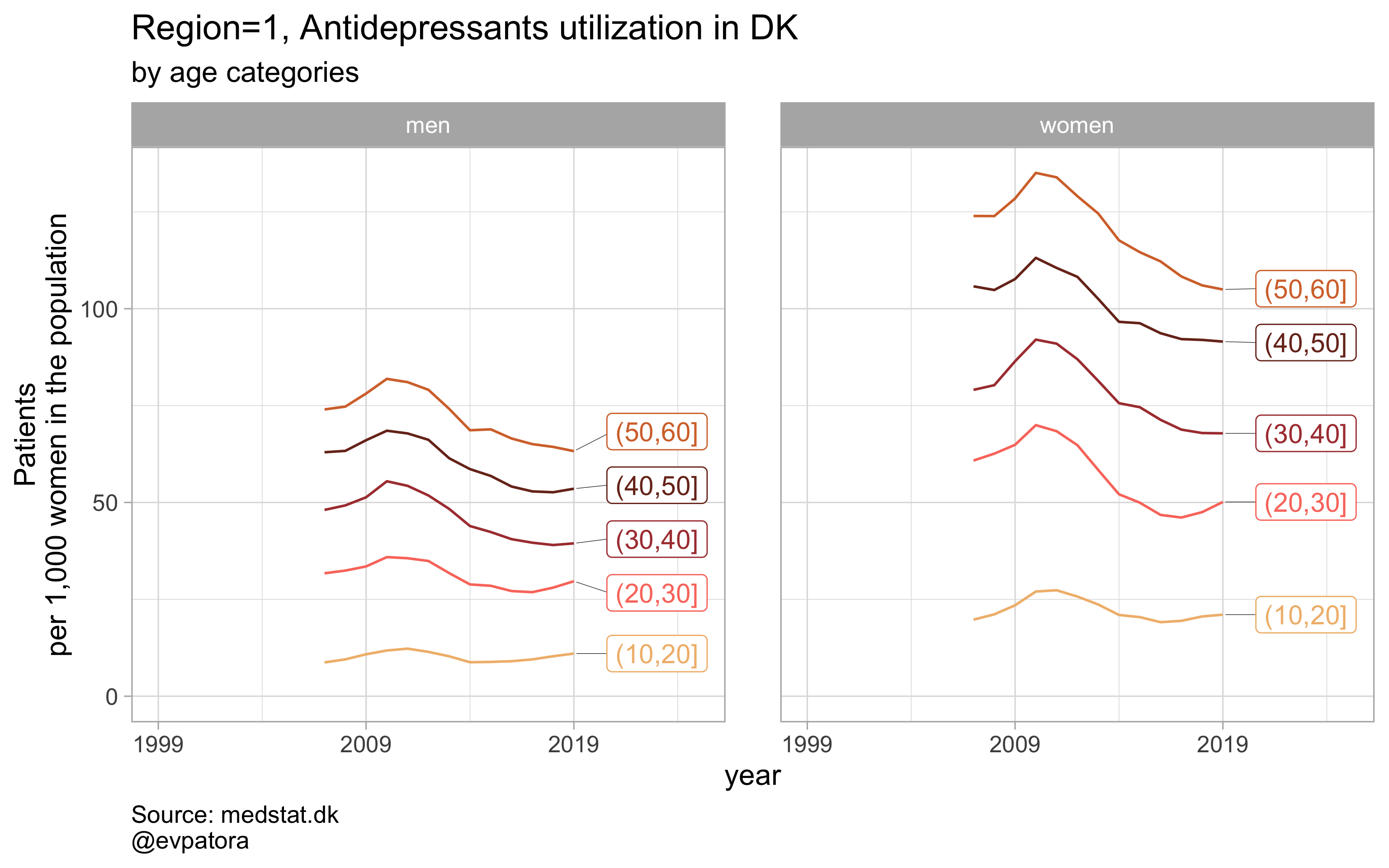##
## []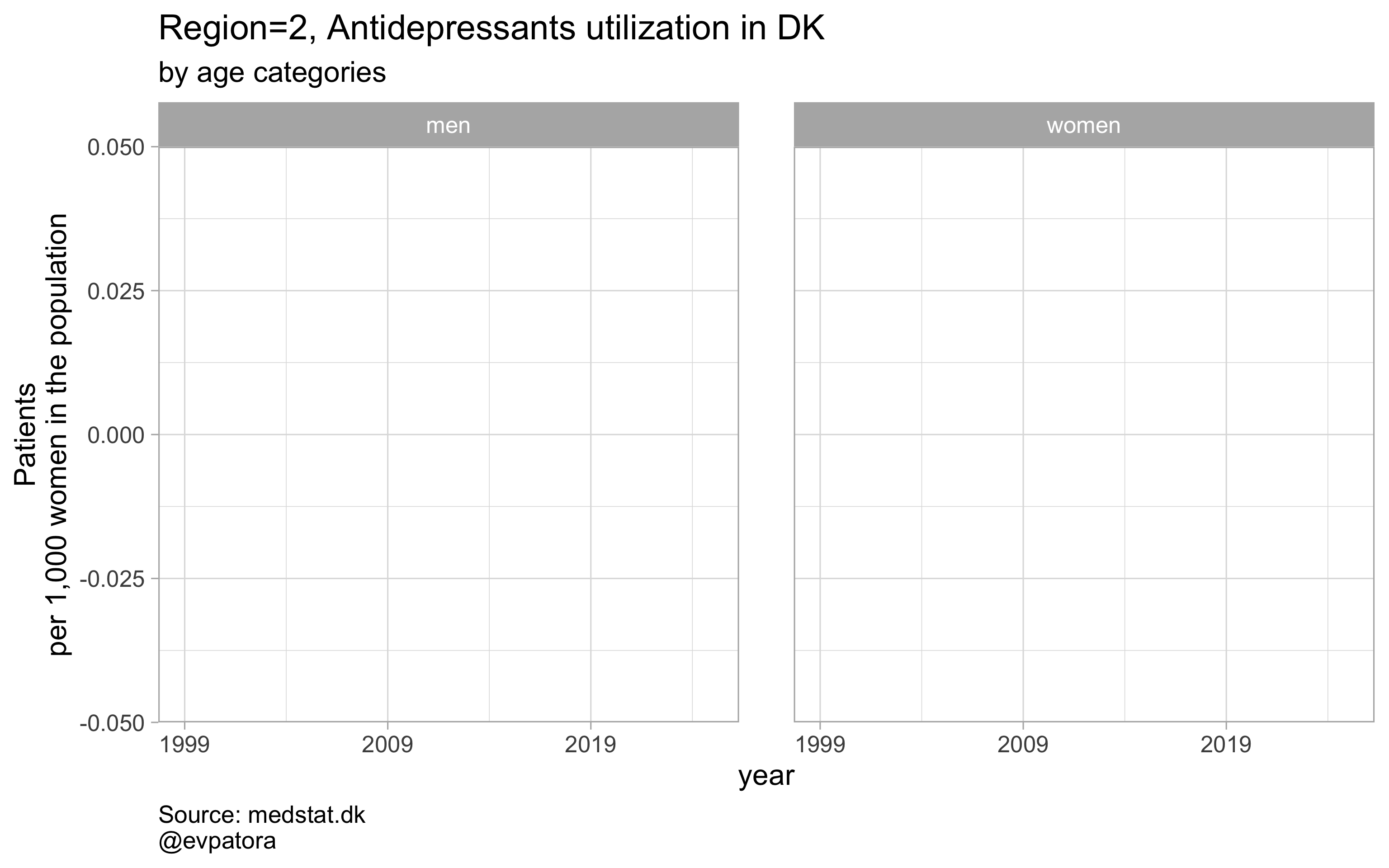##
## []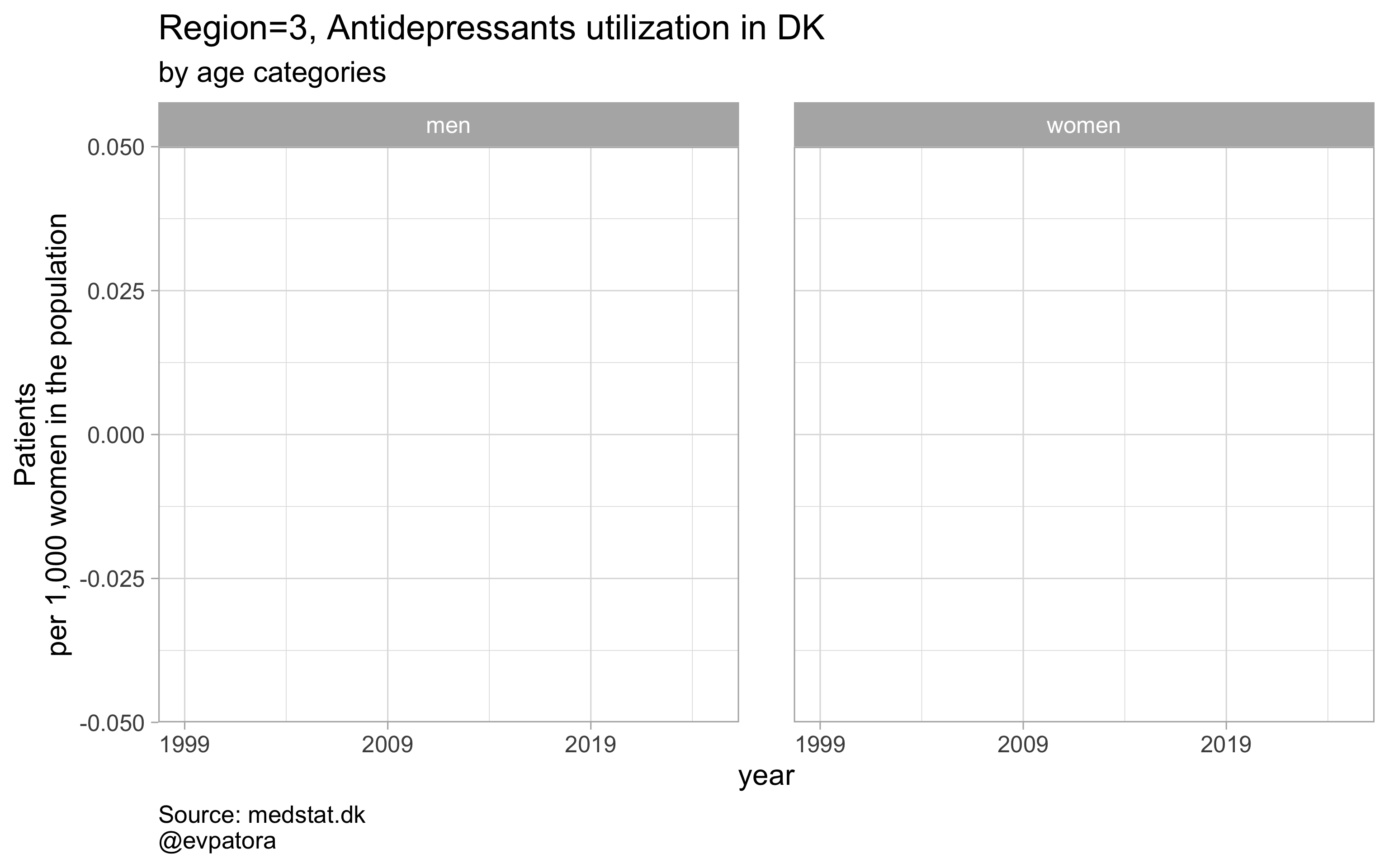##
## []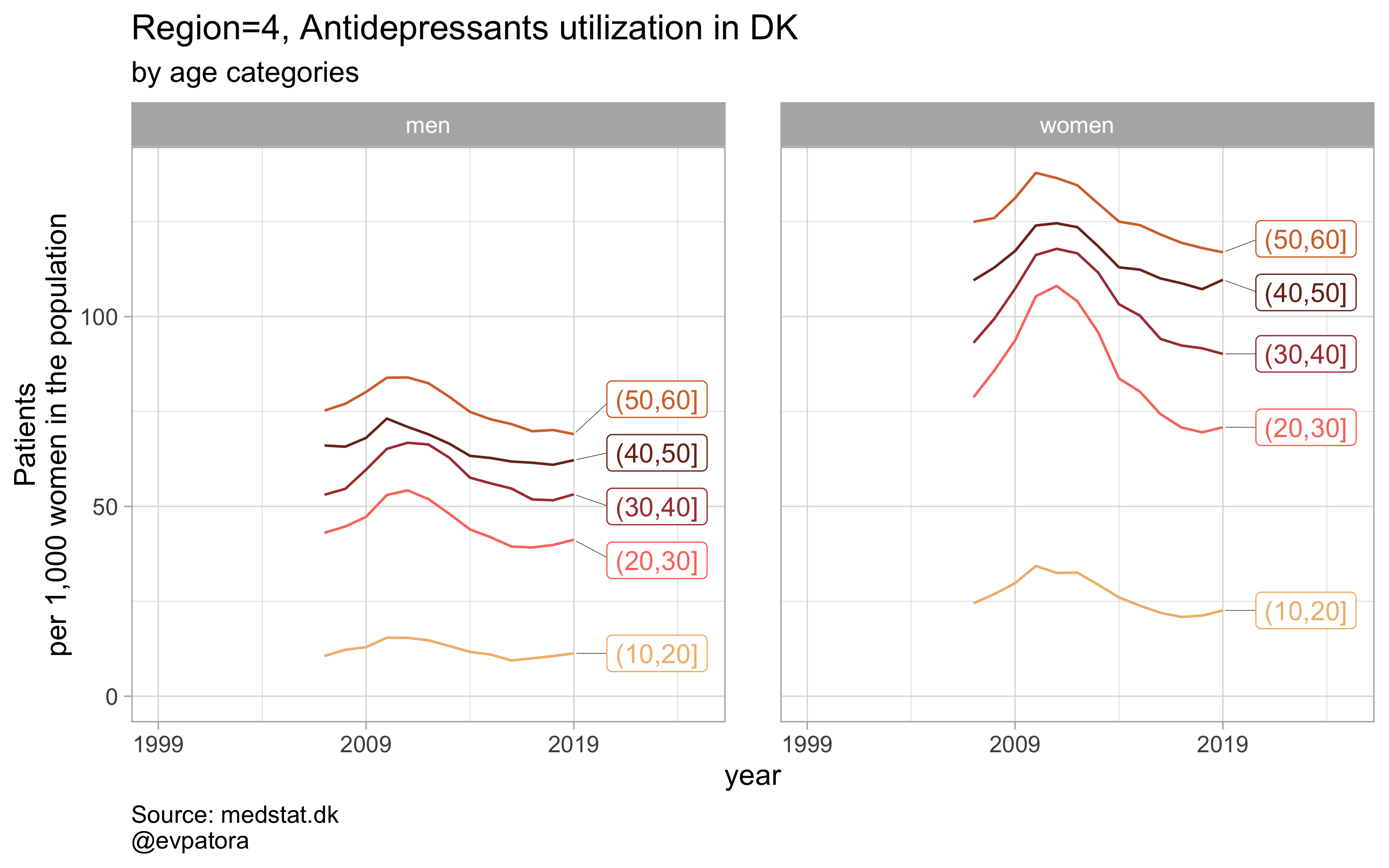##
## []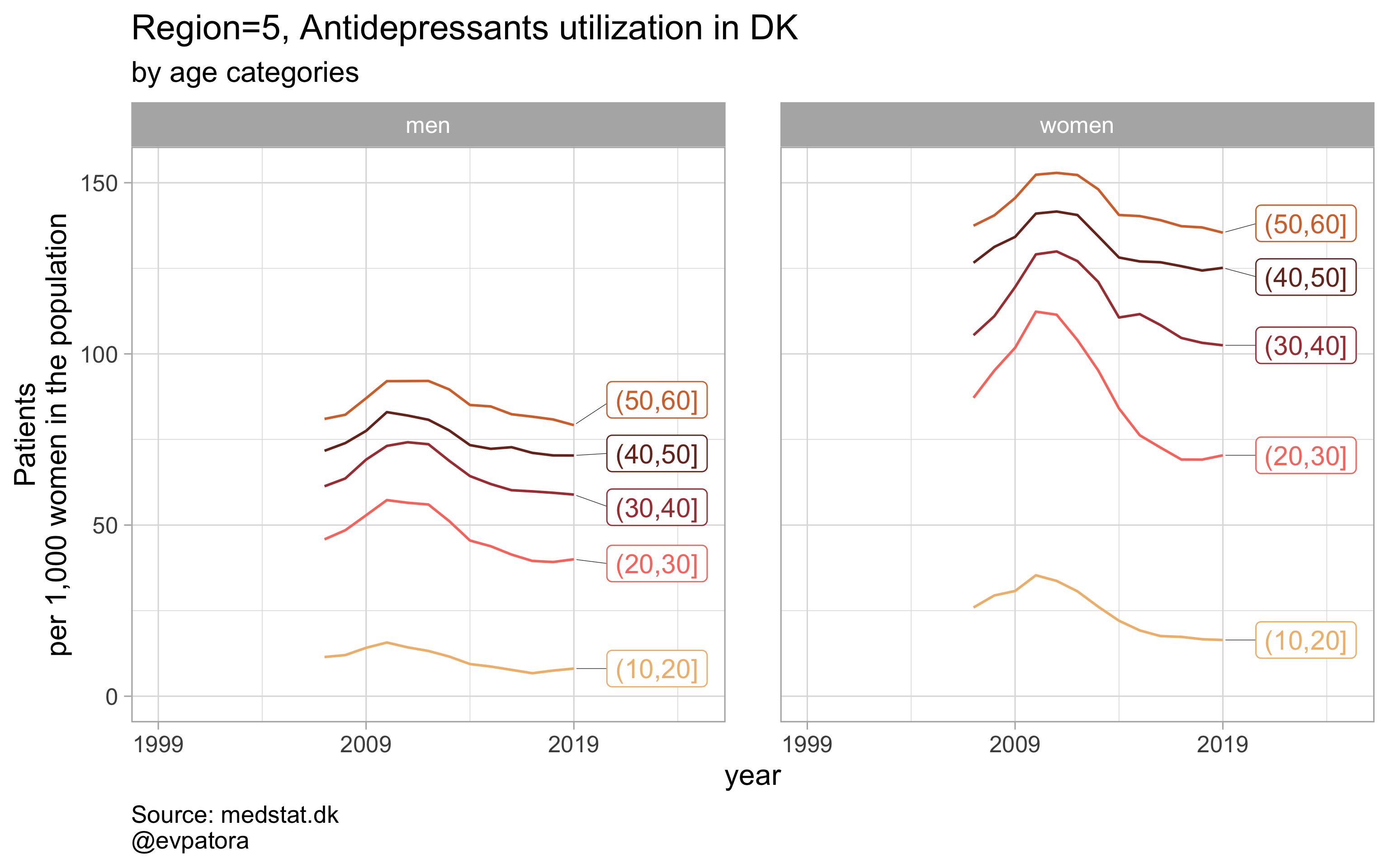##
## []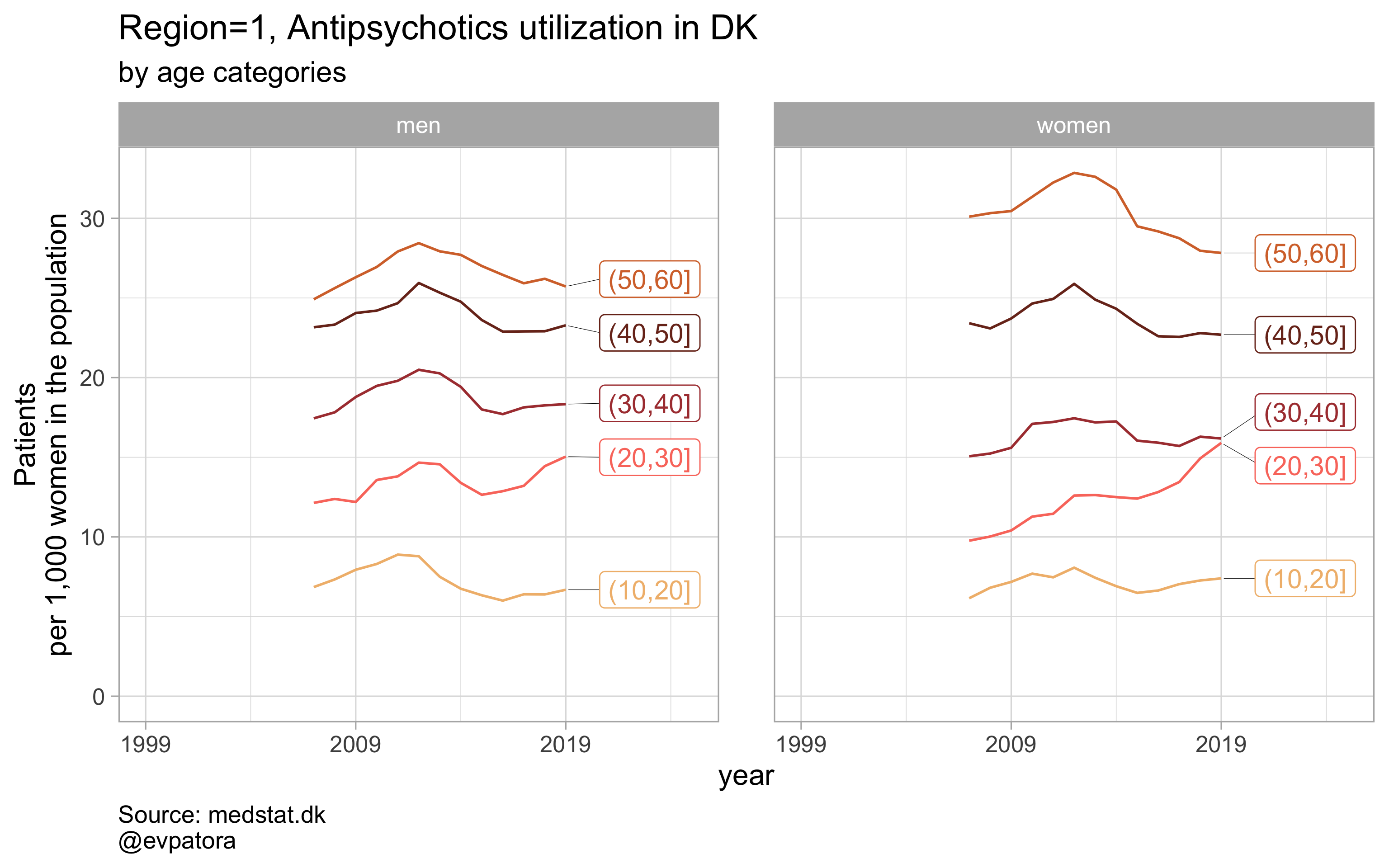##
## []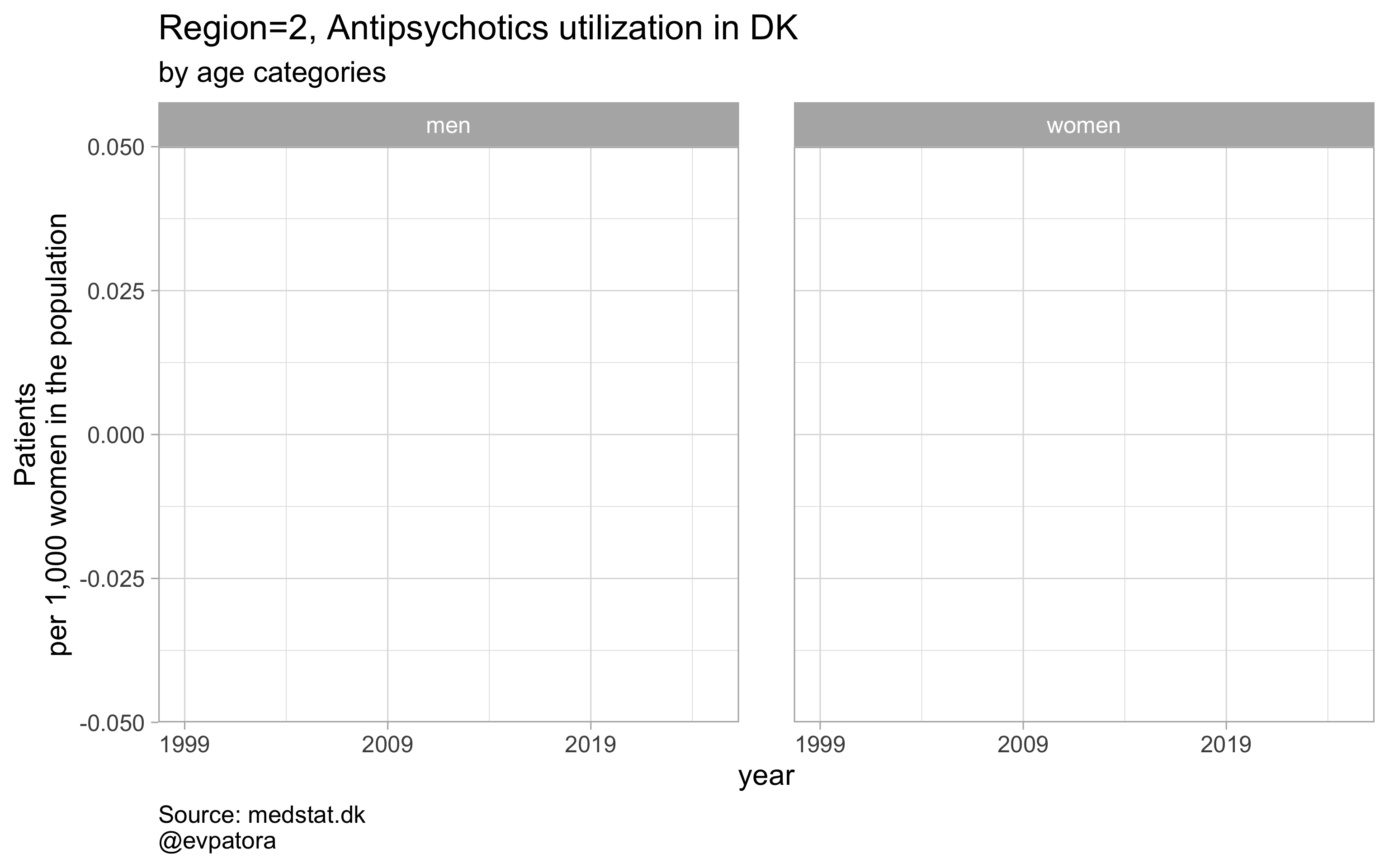##
## []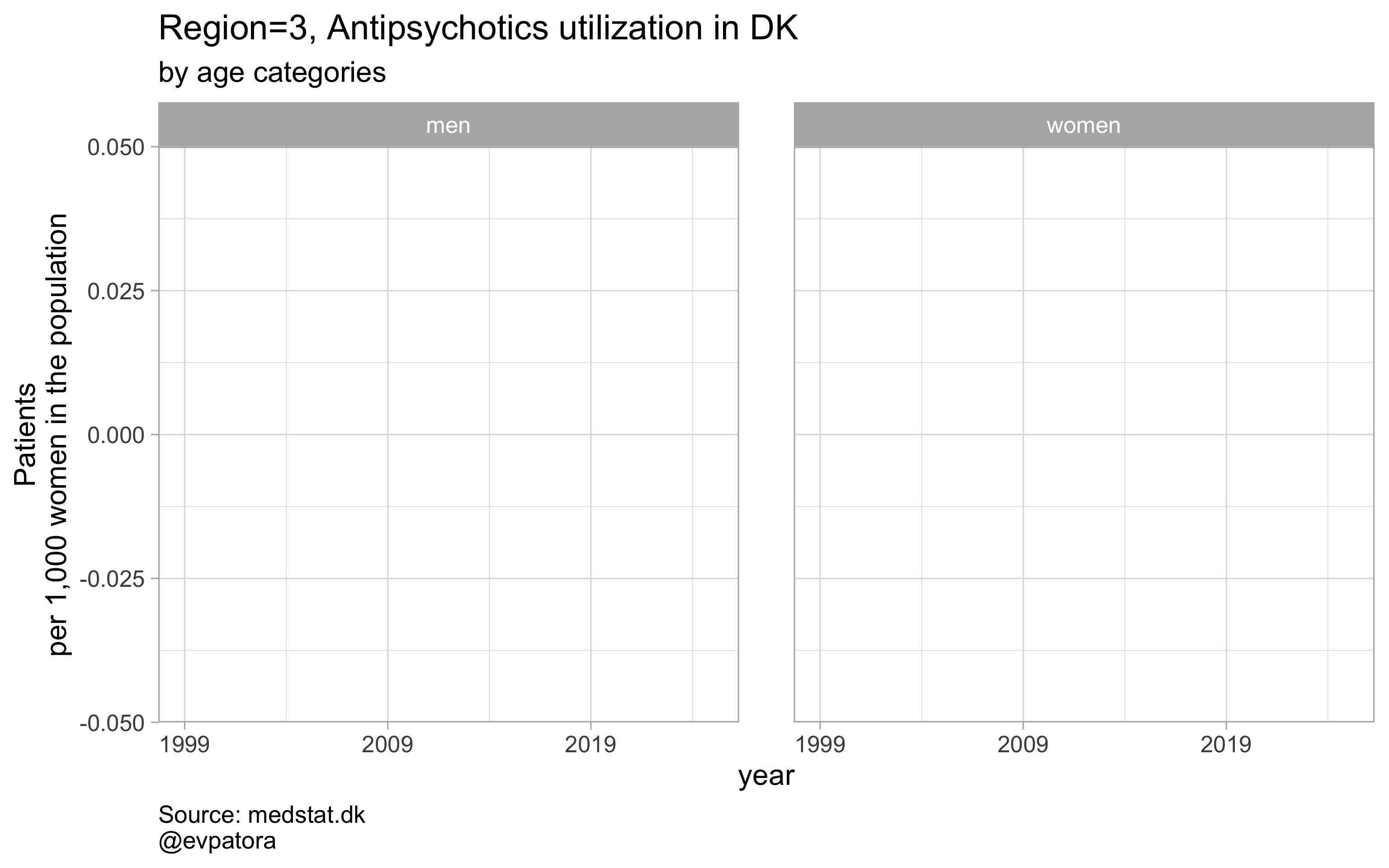##
## []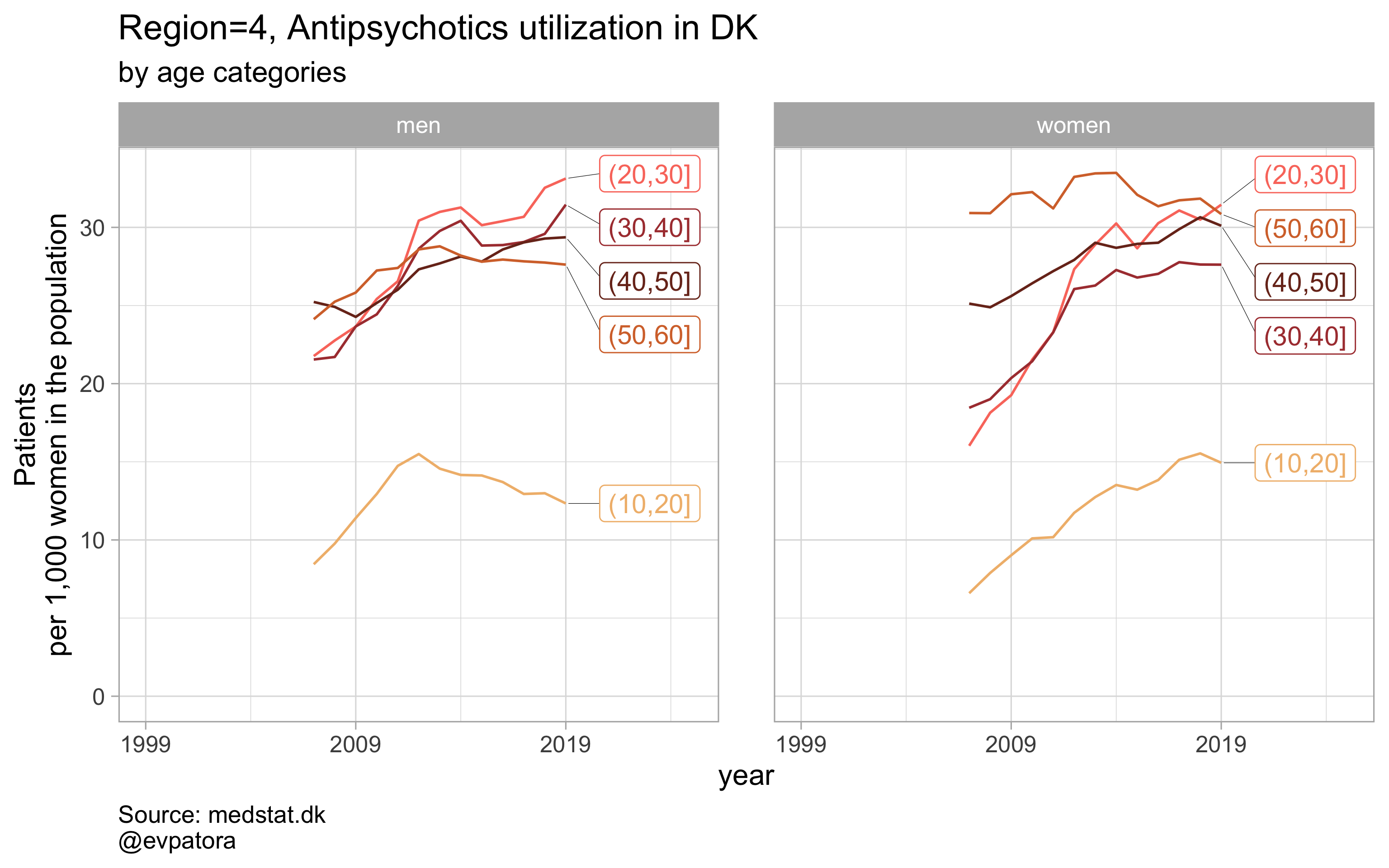##
## []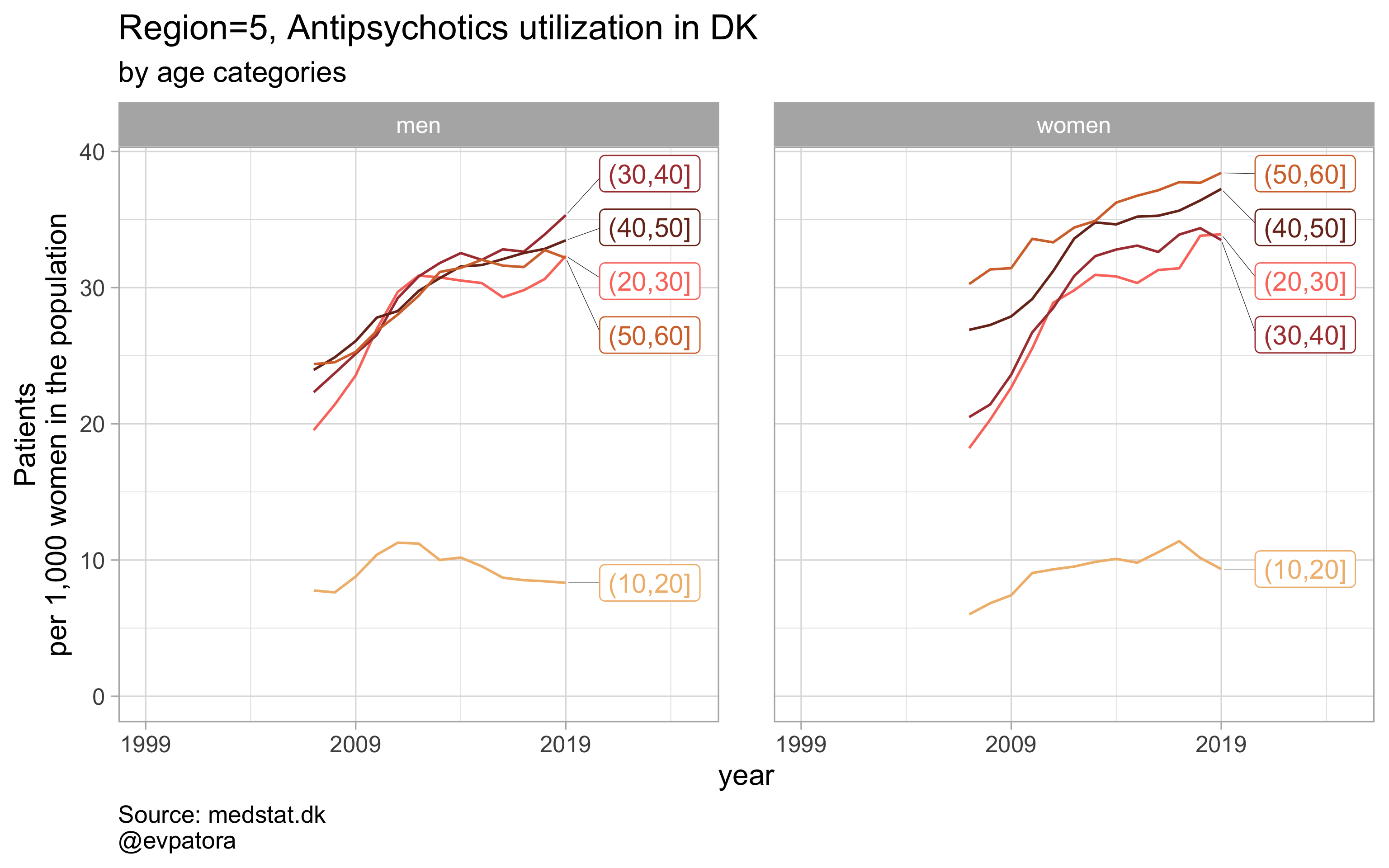##
## []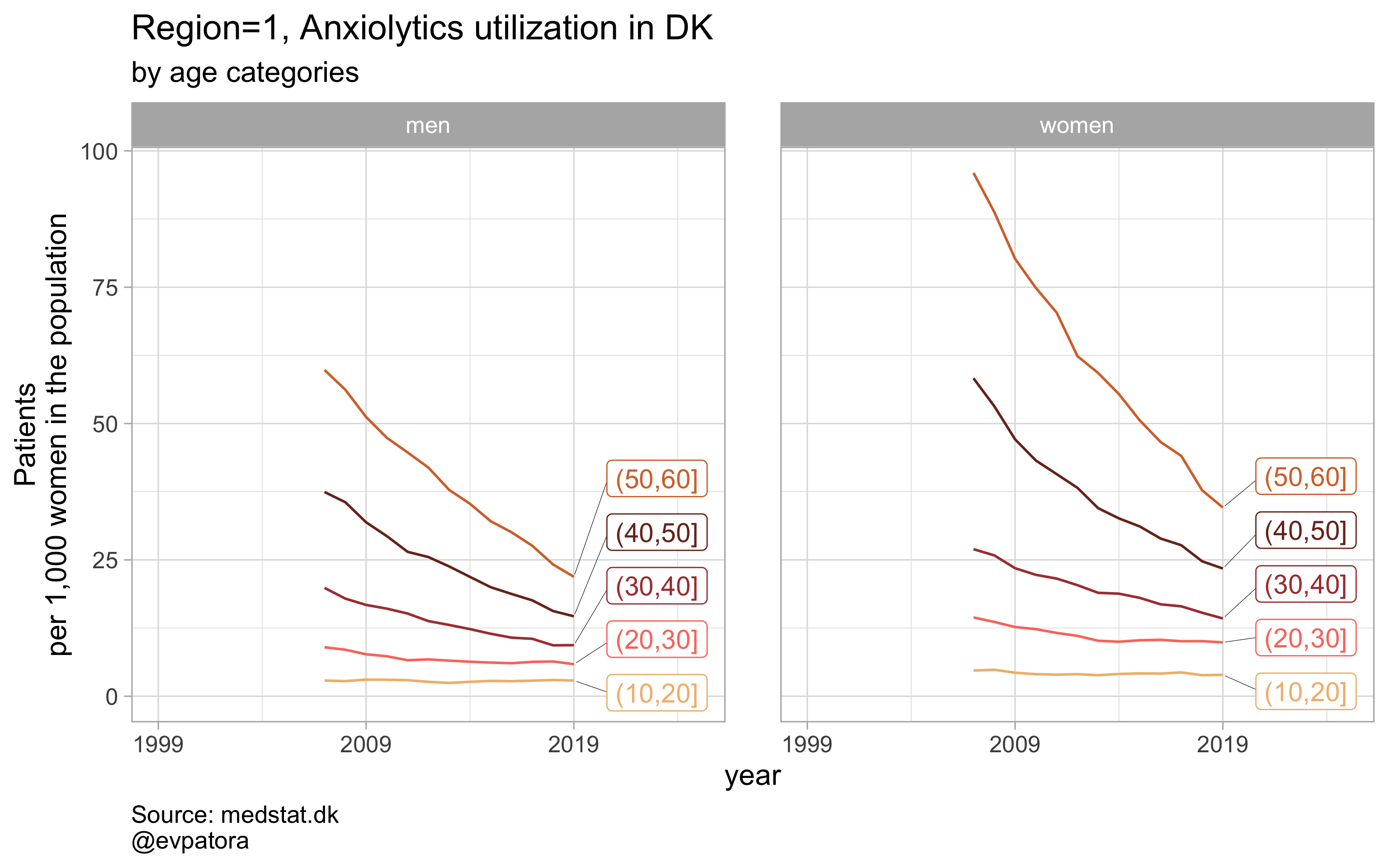##
## []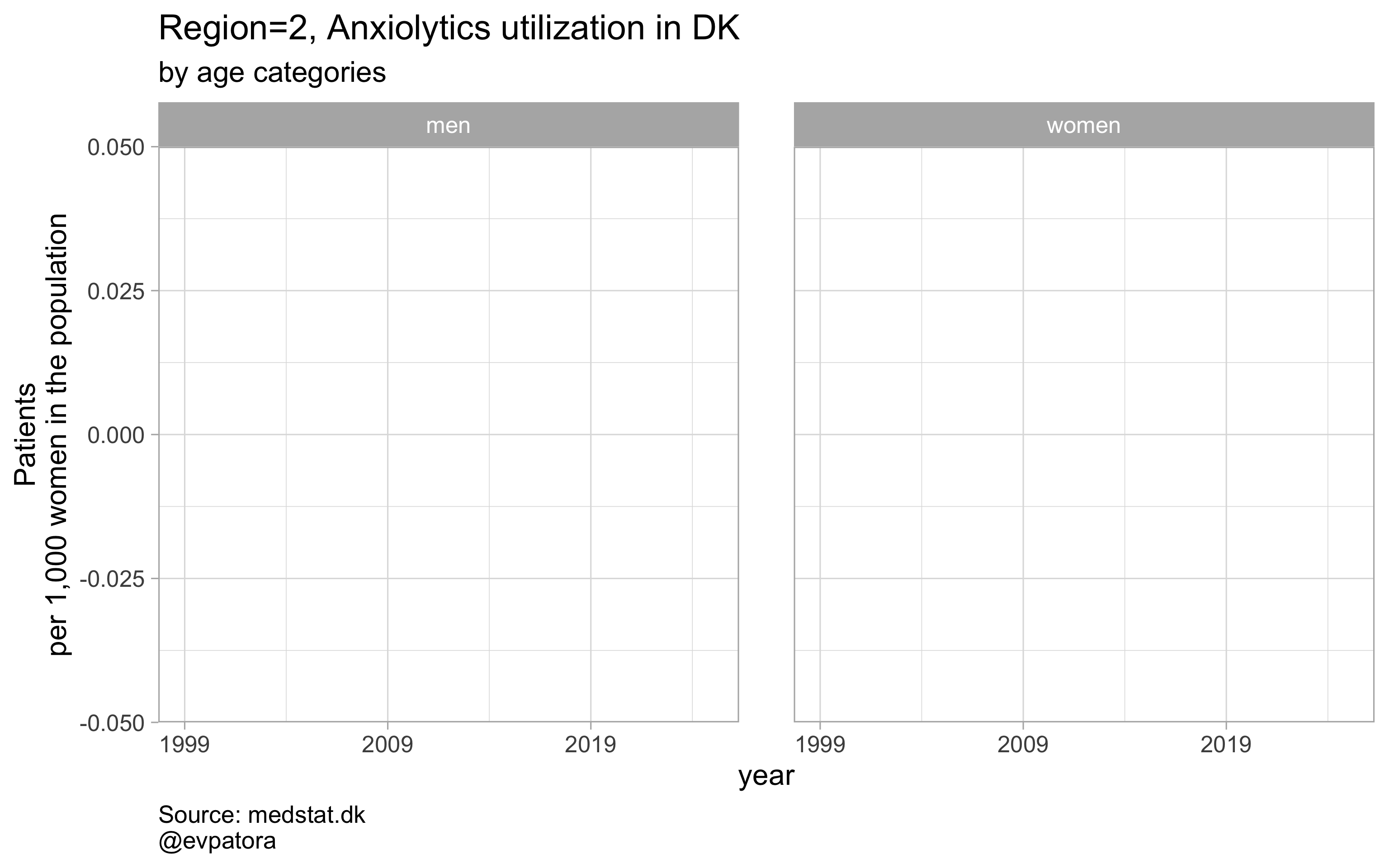##
## []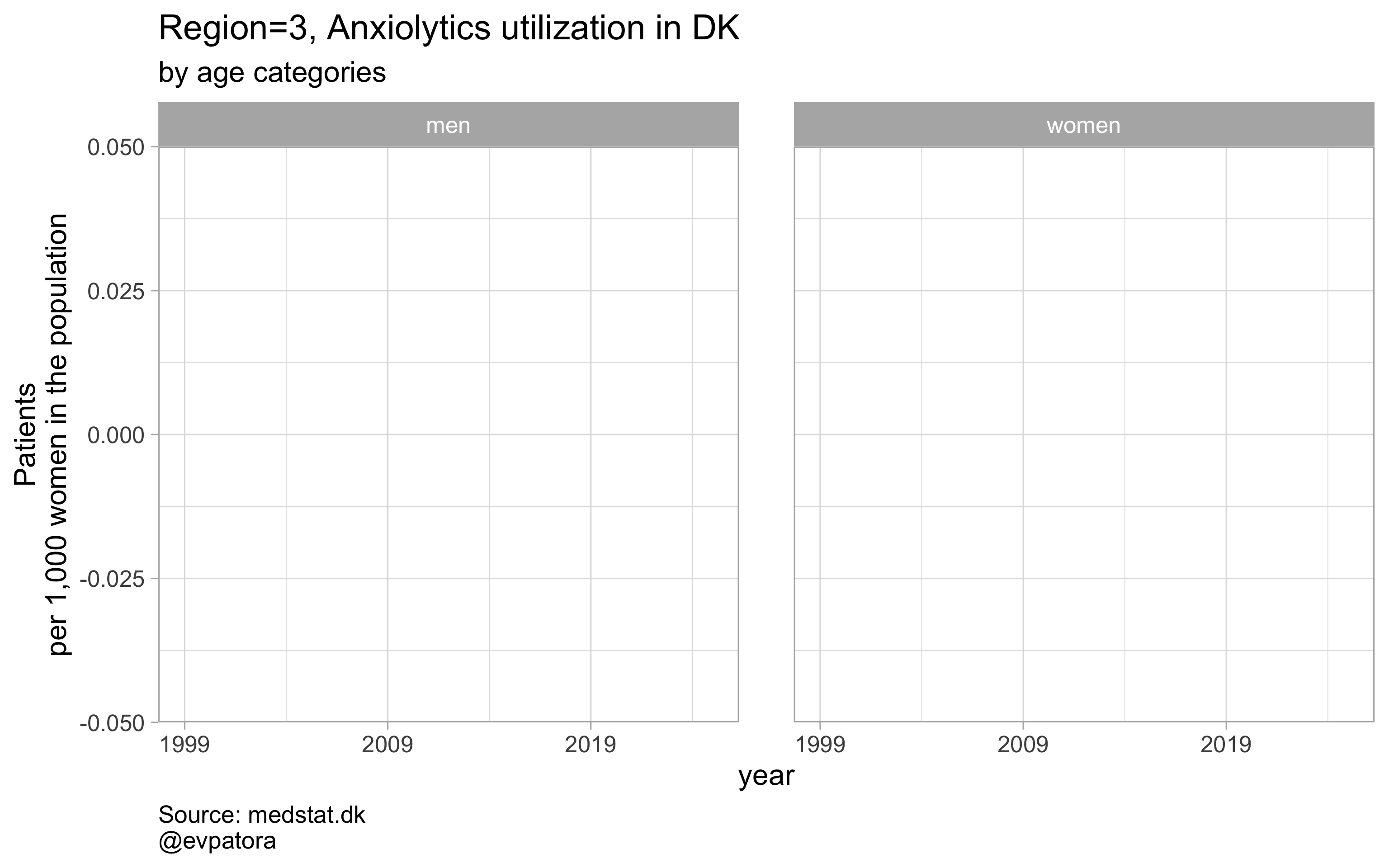##
## []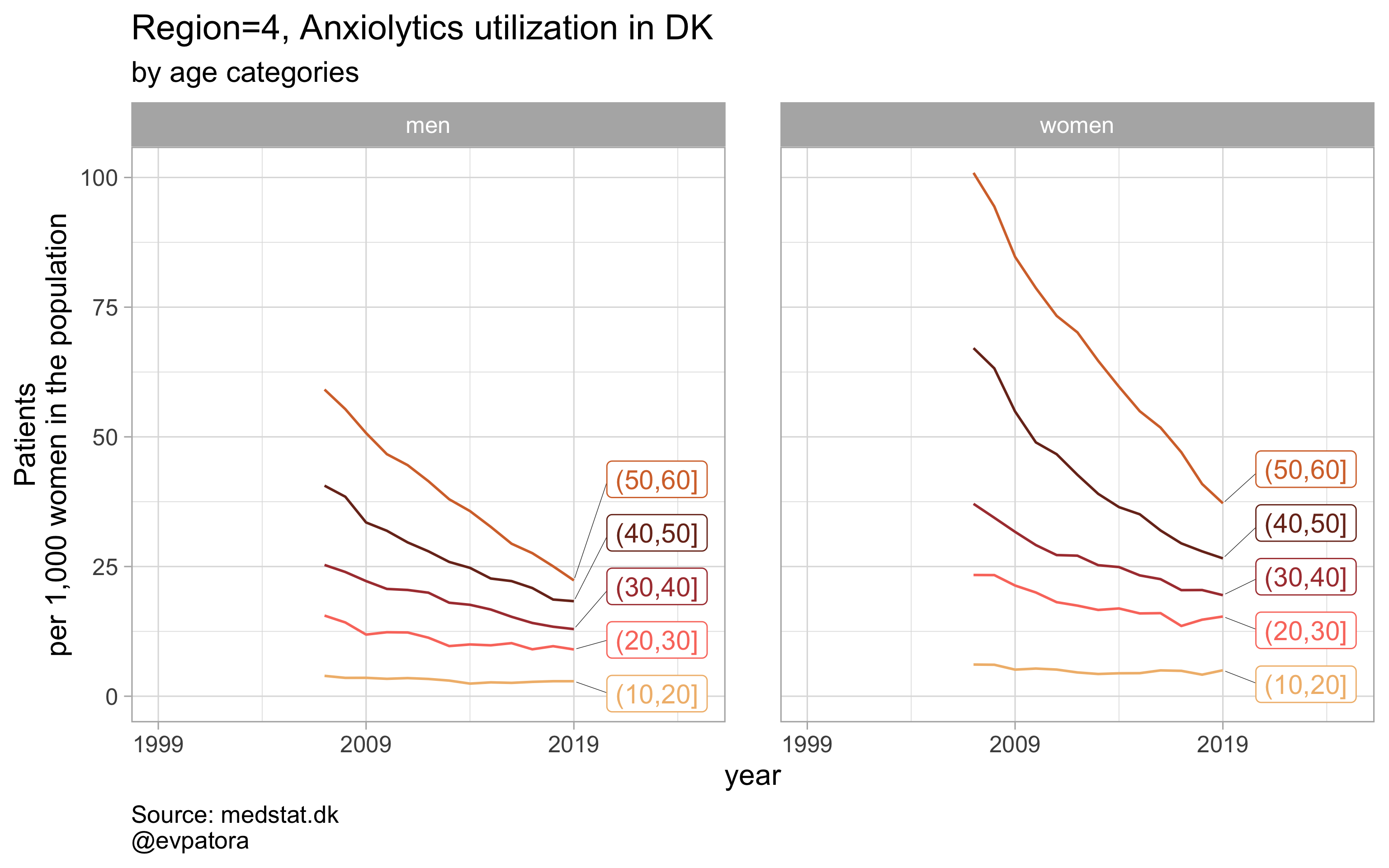##
## []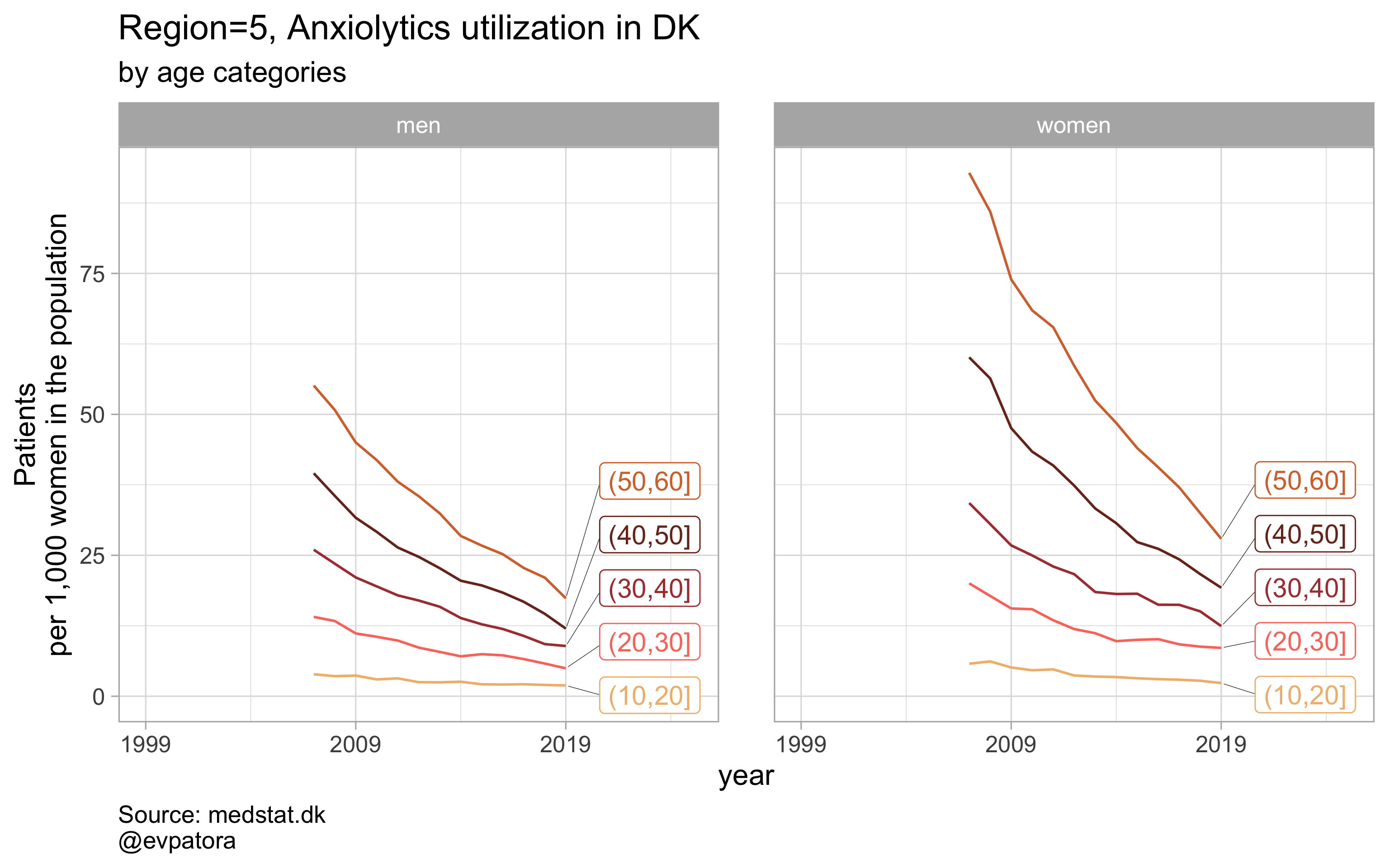##
## []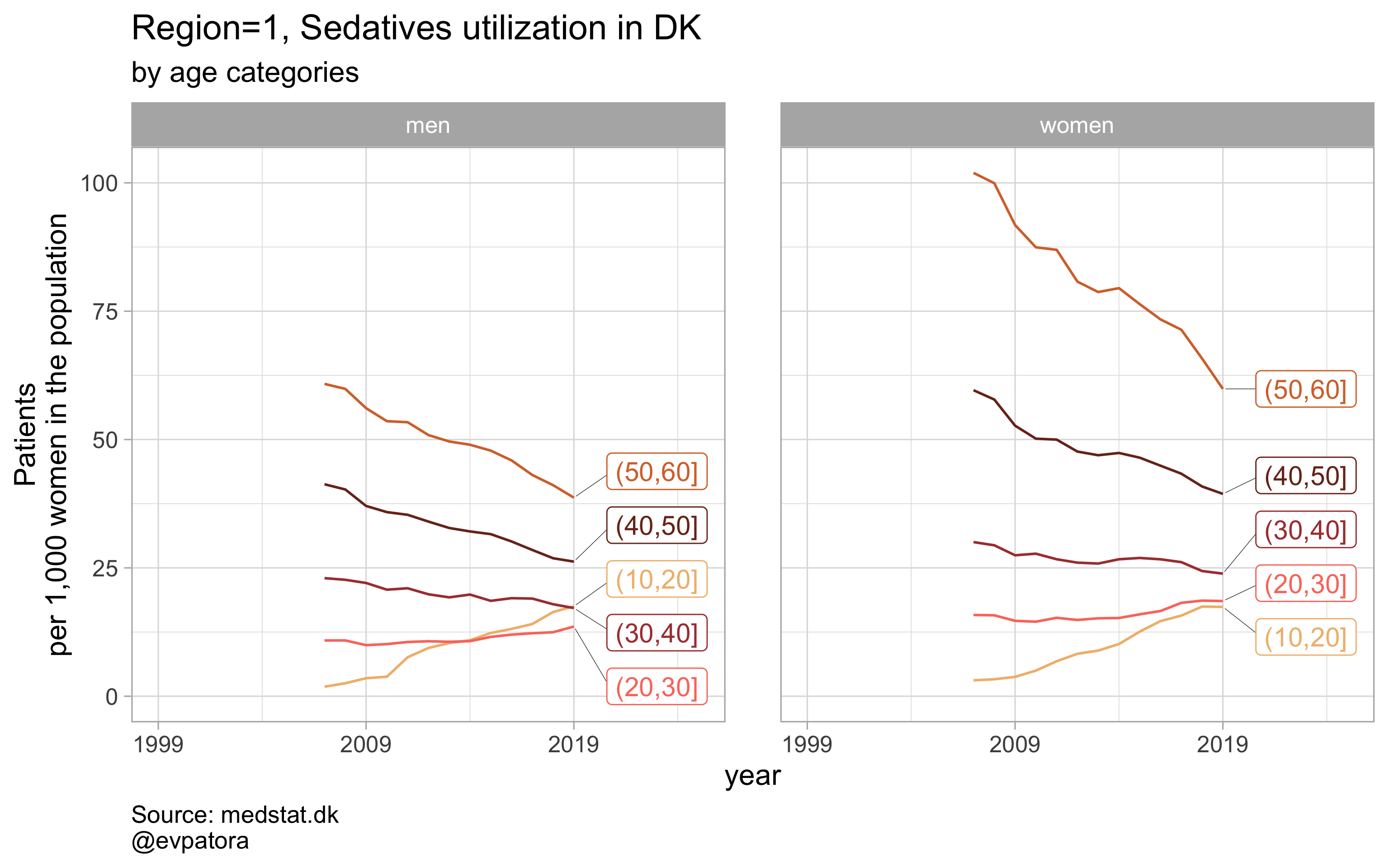##
## []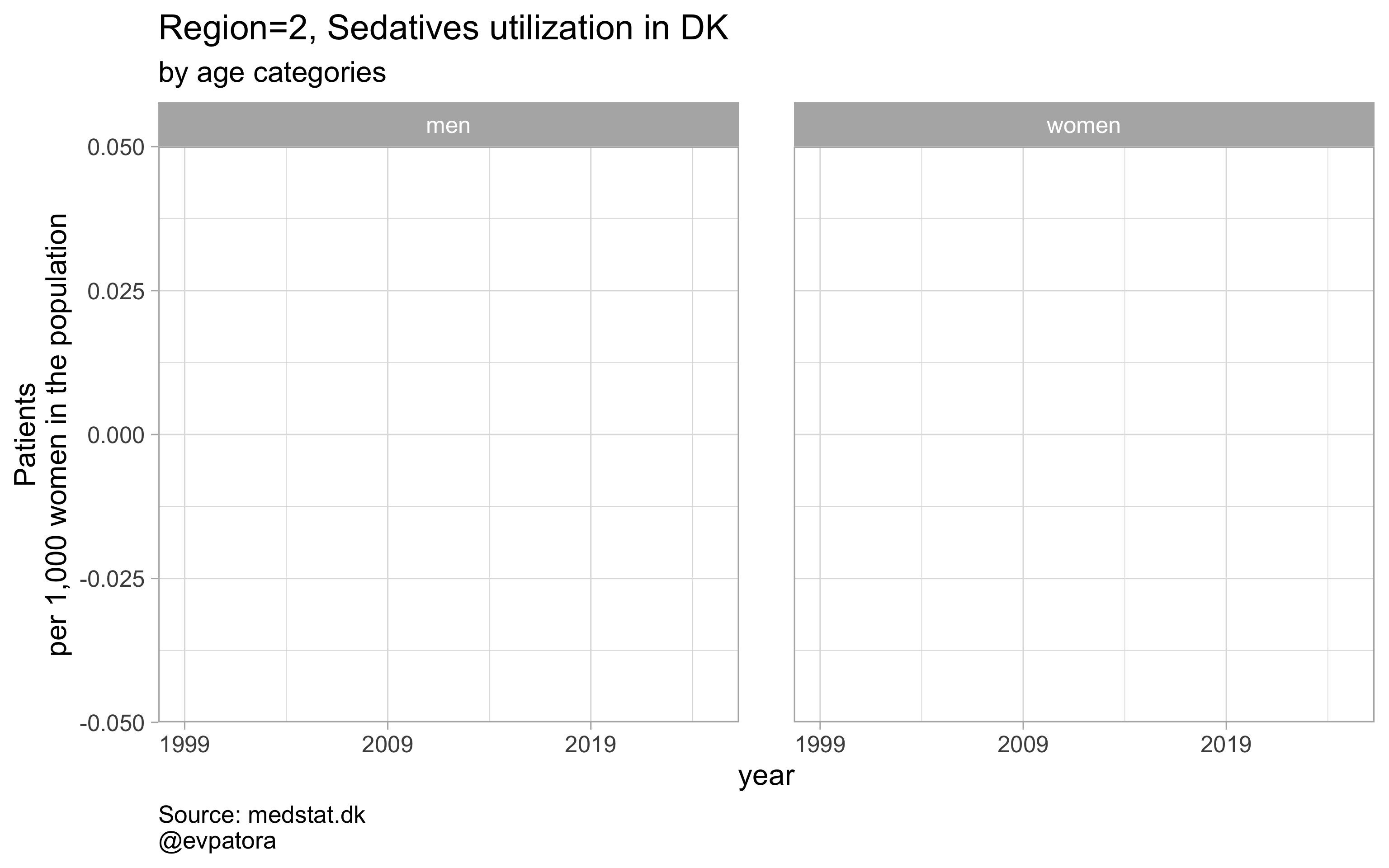##
## []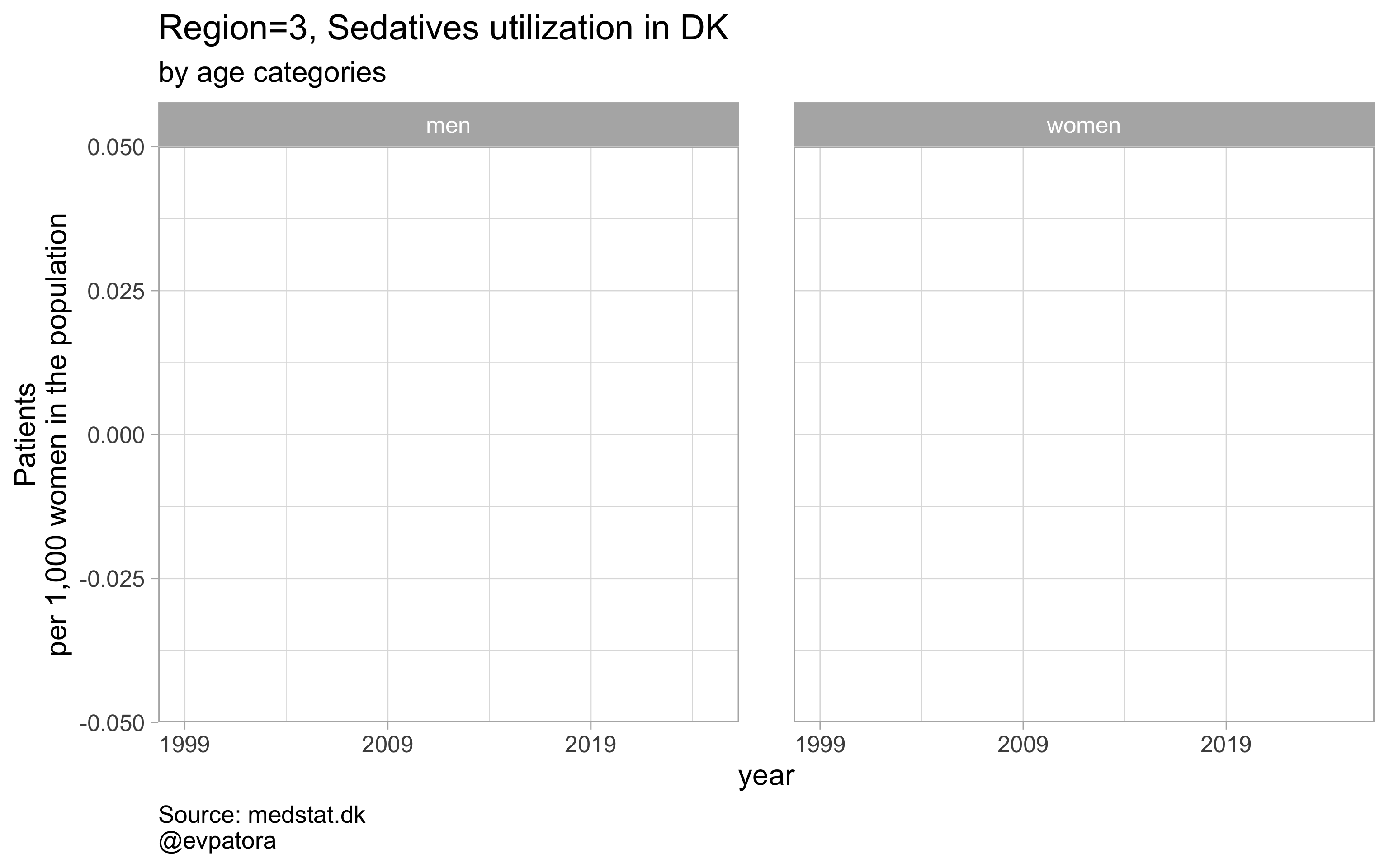##
## []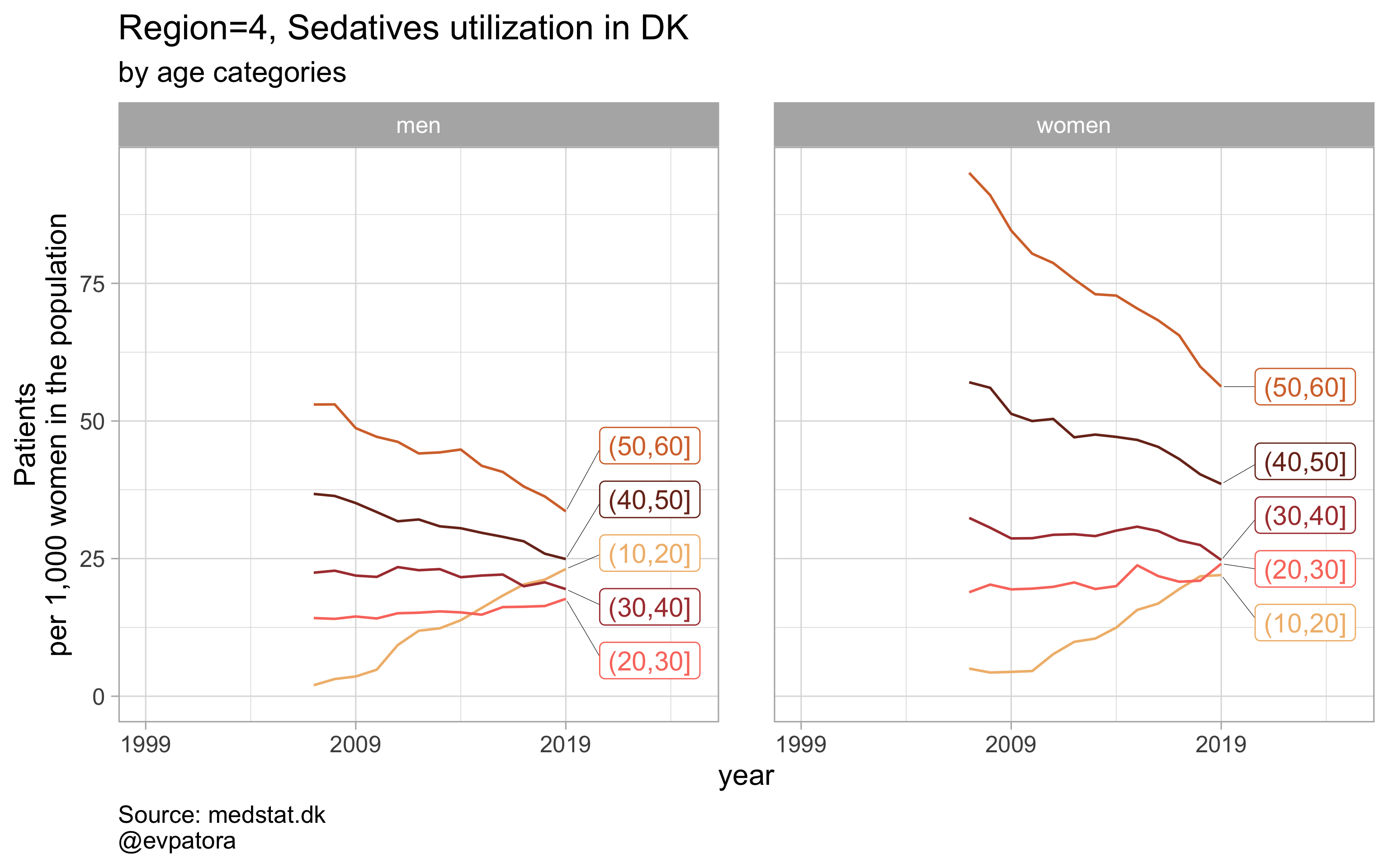##
## []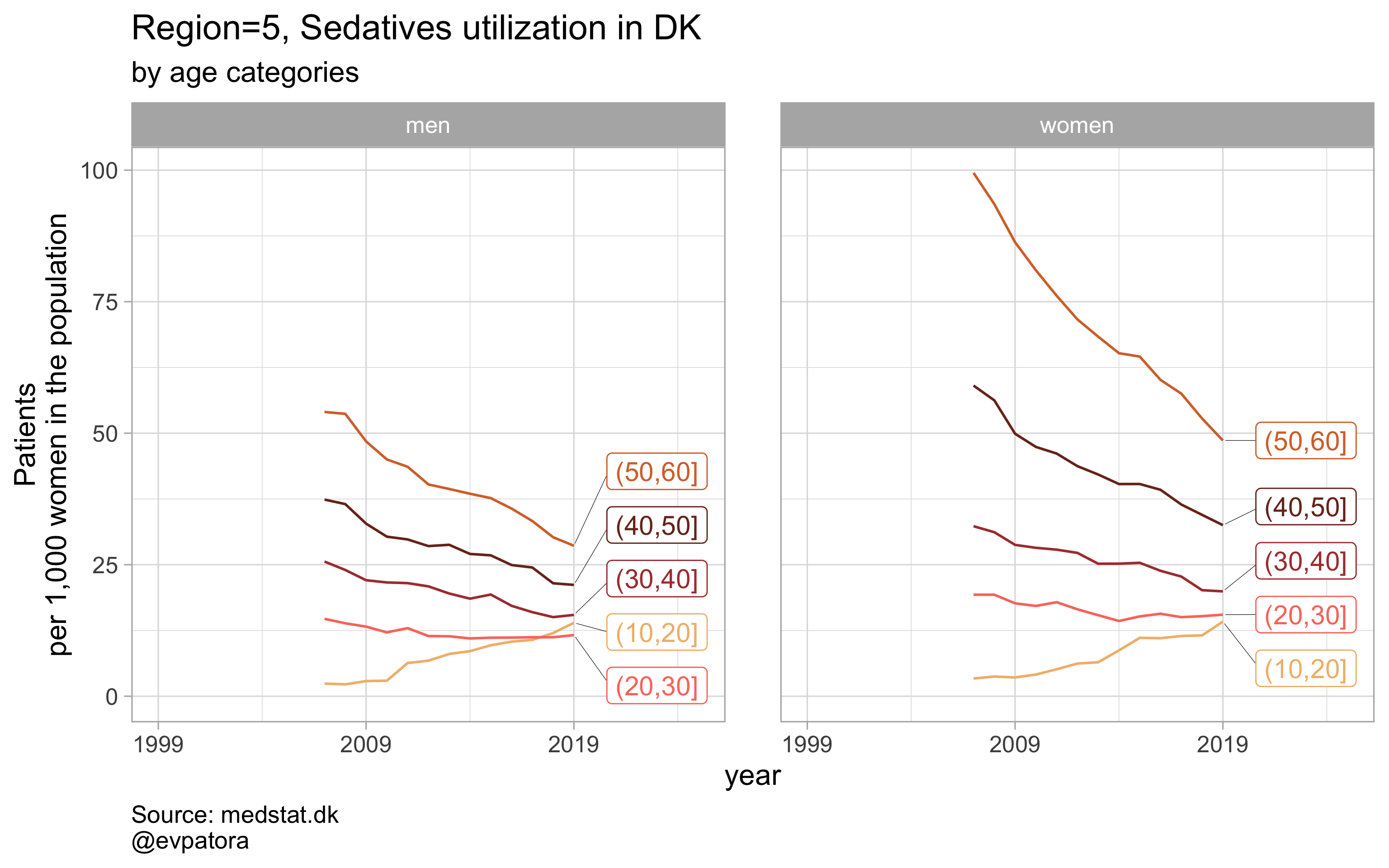# save all plots with one line: each saved plot has the name of the parameters plotted (medication & region)
walk2(.x = list_plots, .y = list_title, ~ggsave(filename = paste0(Sys.Date(), "-", .y, ".pdf"),
plot = .x, path = getwd(), device = cairo_pdf,
width = 297, height = 210, units = "mm"))


### Option 3

# I recode region_var as numeric to simplify my life on the filtering step
# for all regions & for selected age categories, region facets, no age facets
# use different color scheme for each drug with "GrandBudapest1" from {wesanderson} as a default color scheme
plot_utilization <- function(.my_data, drug_regex, atc, age_var, year_var, rate_var, sex_var, title, region_var, region_setting, age_numeric, age_setting, color_setting = "GrandBudapest1", n = 5){

.my_data %>%
mutate(
region_text := case_when(
{{ region_var }} == "0" ~ "DK",
{{ region_var }} == "1" ~ "Region Hovedstaden",
{{ region_var }} == "2" ~ "Region Midtjylland",
{{ region_var }} == "3" ~ "Region Nordjylland",
{{ region_var }} == "4" ~ "Region Sjælland",
{{ region_var }} == "5" ~ "Region Syddanmark",
T ~ NA_character_
),
{{ region_var }} := as.numeric({{ region_var }})
) %>%
# filter non-missing values on utilization rates
filter(! is.na({{ rate_var }})) %>%
filter({{ age_numeric }} %in% age_setting, {{ region_var }} %in% region_setting, str_detect({{ atc }}, drug_regex)) %>%
# make label for the year 2019
mutate(label = if_else({{ year_var }} == 2019, as.character({{ age_var }}), NA_character_)) %>%
ggplot(aes(x = {{ year_var }}, y = {{ rate_var }}, color = {{ age_var }})) +
geom_path() +
facet_grid(cols = vars({{ sex_var }}), rows = vars(region_text), scales = "fixed", drop = T) +
theme_light(base_size = 12) +
scale_x_continuous(limits = c(1999, 2025), breaks = c(seq(1999, 2019, 10))) +
expand_limits(y = 0) +
scale_color_manual(values = wes_palette(name = color_setting, type = "continuous", n = n)) +
ggrepel::geom_label_repel(aes(label = label), na.rm = TRUE, nudge_x = 4, direction = "y", segment.size = 0.1, segment.colour = "black", show.legend = F) +
theme(plot.caption = element_text(hjust = 0, size = 10),
legend.position = "none",
panel.spacing = unit(0.8, "cm")) +
labs(y = "Patients\nper 1,000 women in the population", title = paste0(title, " in DK"), subtitle = "by age categories", caption = "Regions with missing data on drug utilization rate are excluded\nSource: medstat.dk\n@evpatora")
}

# 4 drugs for 5 regions by age group
list_regex <- list(regex_antidepress, regex_antipsych, regex_anxiolyt, regex_sedat)
list_drug_name <- list("Antidepressants", "Antipsychotics", "Anxiolytics", "Sedatives")
# compile list of titles from the list of drug names and some text
list_title <- map(list_drug_name, ~paste0("Utilization rates of ", .x, " in men vs women"))
# list of colors to iterate along; each color will correspond to the drug class
list_color <- list("GrandBudapest1", "GrandBudapest2", "IsleofDogs1", "IsleofDogs2")

# iteration
list_plots <- pmap(.l = list(list_regex, list_title, list_color),
.f = ~plot_utilization(.my_data = data, atc = ATC, drug_regex = ..1, age_var = age_cat,
year_var = year, rate_var = patients_per_1000_inhabitants, sex_var = gender_text,
title = ..2, region_var = region, region_setting = 0:5, age_numeric = age,
age_setting = 10:60, color_setting = ..3))

# see some results
list_plots

## []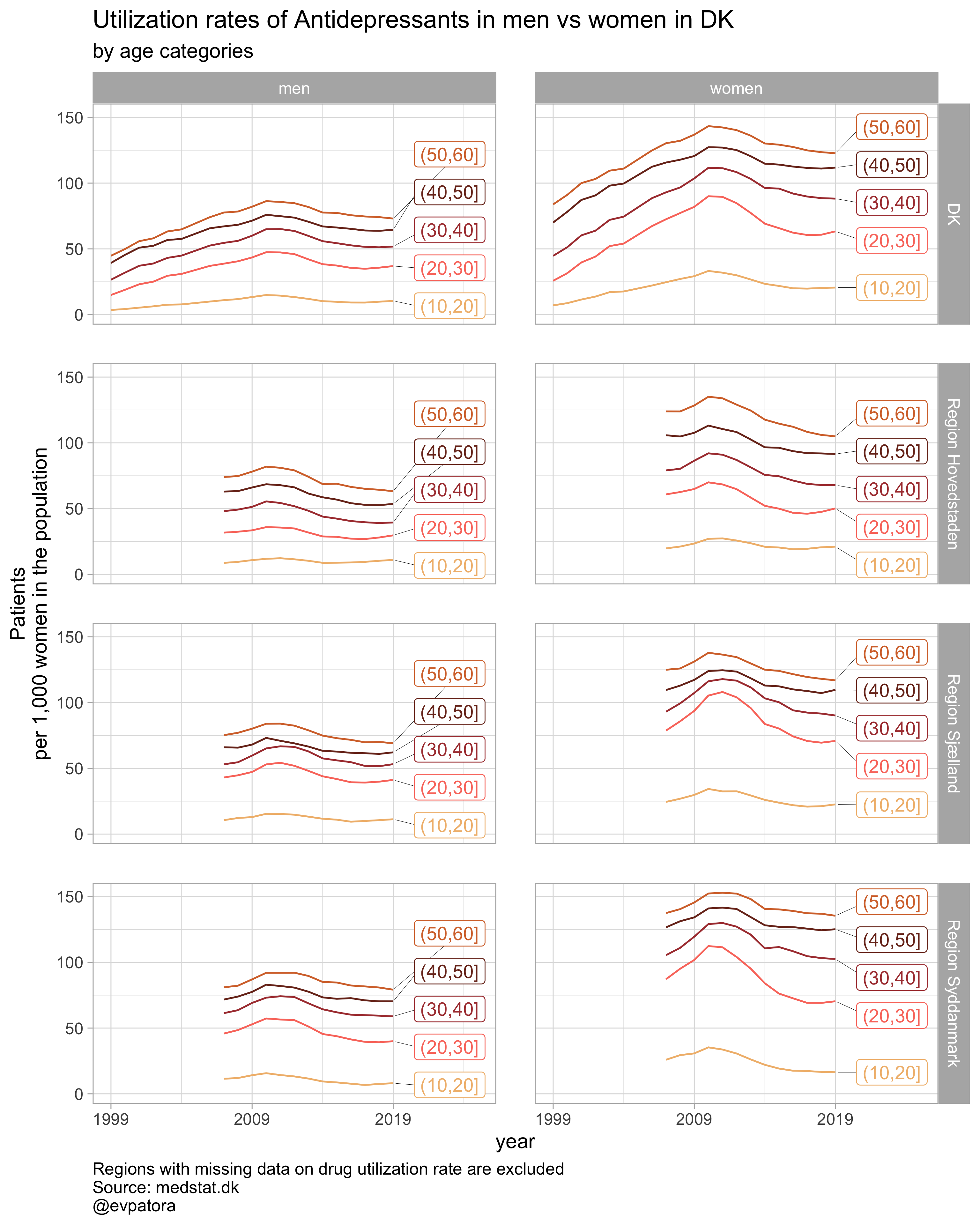##
## []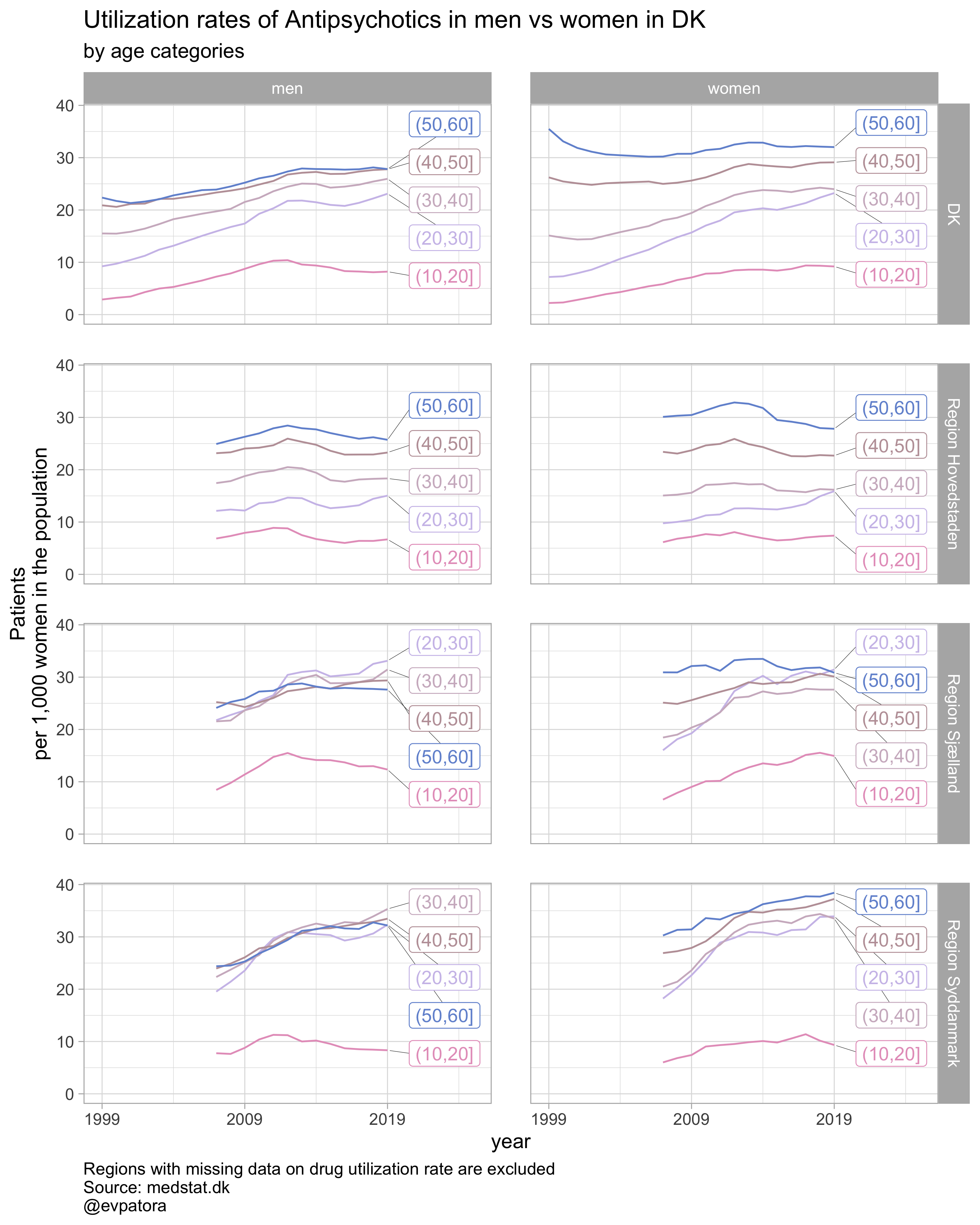##
## []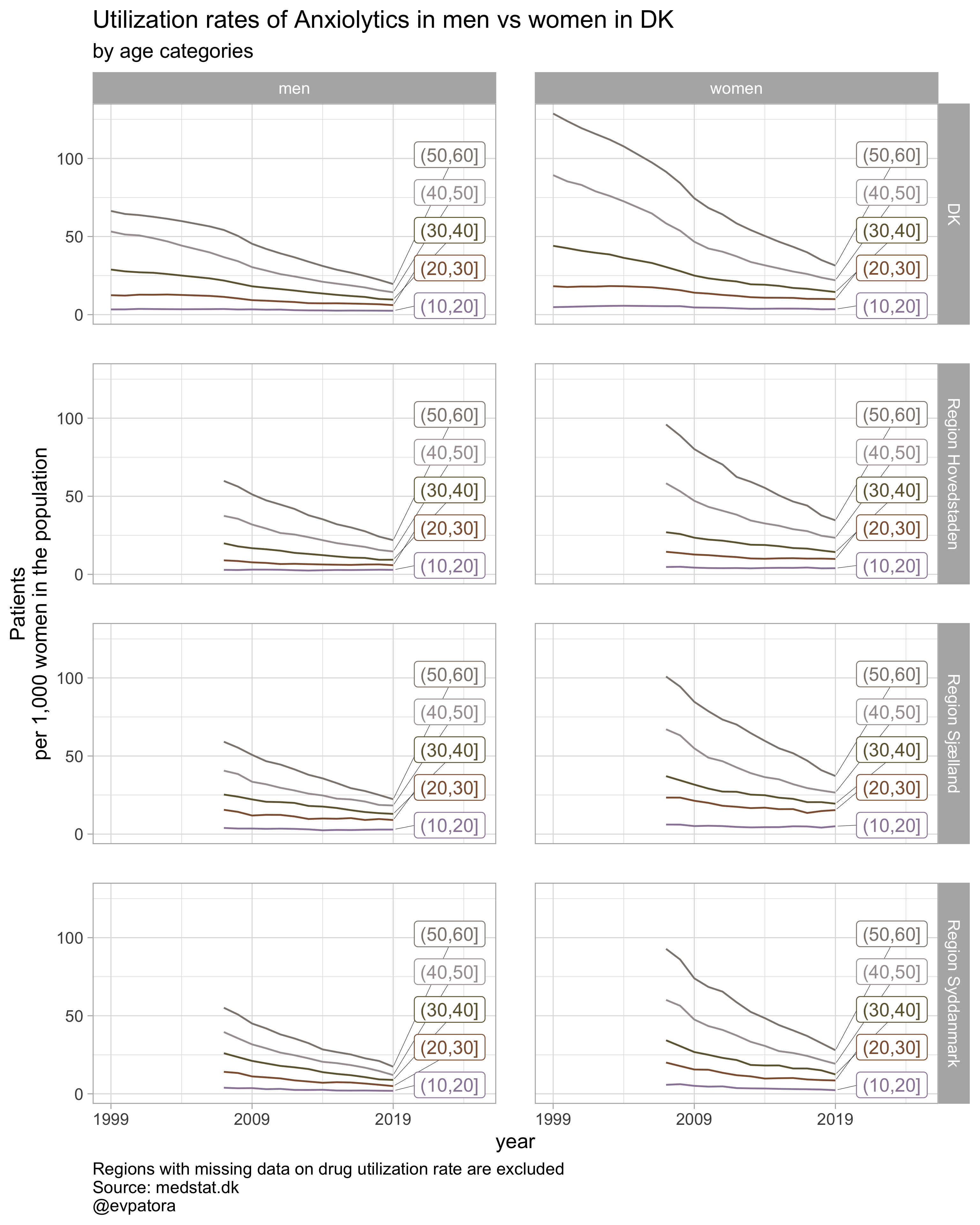##
## []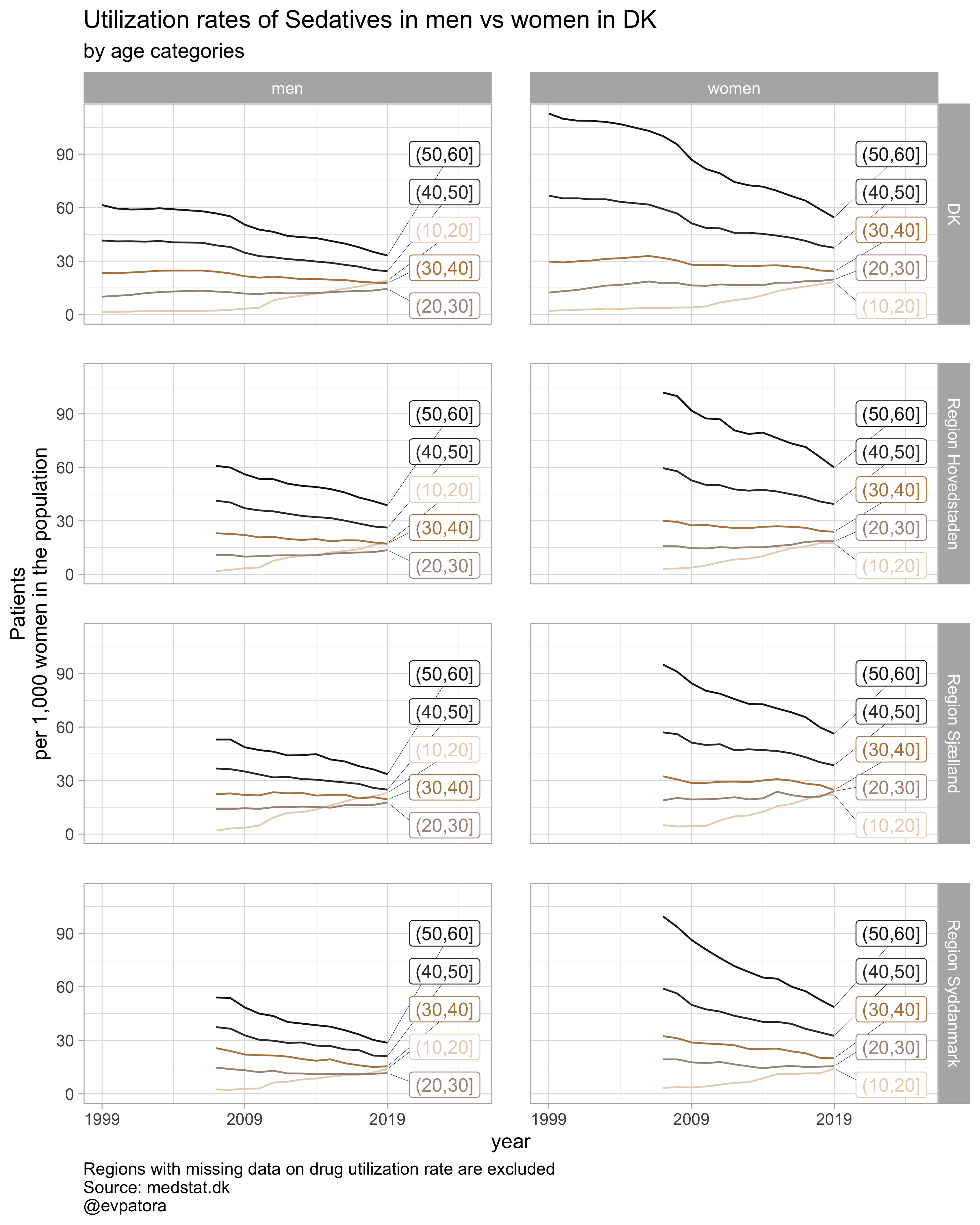# save all plots with one line: each saved plot has the name of the plotted characteristics
walk2(.x = list_plots, .y = list_title, ~ggsave(filename = paste0(Sys.Date(), "-", .y, ".pdf"),
plot = .x, path = getwd(), device = cairo_pdf,
width = 297, height = 210, units = "mm"))


## Take home messages

1. Some copy-pasting is hard to avoid
2. Developing understanding of your data will also improve your understanding of how to create more flexible custom functions
3. When optimized, visualization leaves more space to concentrate on interpretation of data

Cheers! ✌️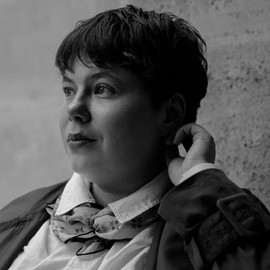##### Elena Dudukina
###### Consultant/Pharmacoepidemiologist

I am interested in women’s health, reproductive epidemiology, pharmacoepidemiology, causal inference, directed acyclic graphs, and R stats.